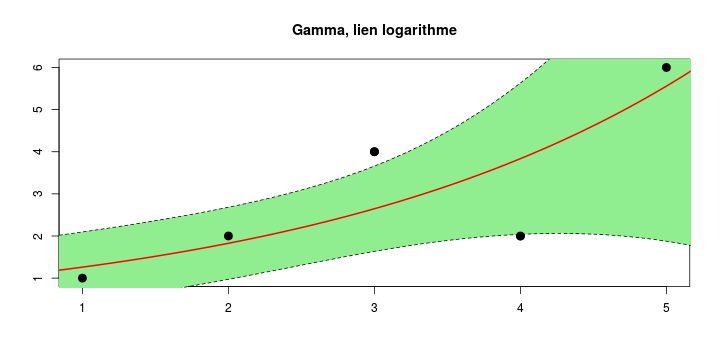# Data Science for Actuaries

Arthur Charpentier, @freakonometrics
2017

##
## Attaching package: 'dplyr'

## The following objects are masked from 'package:stats':
##
##     filter, lag

## The following objects are masked from 'package:base':
##
##     intersect, setdiff, setequal, union

## Utilities for Start-Up Messages (version 0.9.3)

## For more information see ?"startupmsg", NEWS("startupmsg")

##
## Attaching package: 'sfsmisc'

## The following object is masked from 'package:dplyr':
##
##     last

## Utilities for Sweave Together with TeX 'listings' Package (version 0.7.5)

## NOTE: Support for this package will stop soon.
## Package 'knitr' is providing the same functionality in a better way.
## Note that global options are controlled by SweaveListingoptions() ---c.f. ?"SweaveListingoptions".

## For more information see ?"SweaveListingUtils", NEWS("SweaveListingUtils")
## There is a vignette to this package; try vignette("ExampleSweaveListingUtils").

##
## Attaching package: 'SweaveListingUtils'

## The following objects are masked from 'package:base':
##
##     library, require

## Object Oriented Implementation of Distributions (version 2.6)

## Attention: Arithmetics on distribution objects are understood as operations on corresponding random variables (r.v.s); see distrARITH().
## Note that global options are controlled by distroptions() ---c.f. ?"distroptions".

## For more information see ?"distr", NEWS("distr"), as well as
##   http://distr.r-forge.r-project.org/
## Package "distrDoc" provides a vignette to this package as well as to several extension packages; try vignette("distr").

## Extensions of Package 'distr' (version 2.6)

## Note: Packages "e1071", "moments", "fBasics" should be attached /before/ package "distrEx". See distrExMASK().Note: Extreme value distribution functionality has been moved to
##       package "RobExtremes". See distrExMOVED().

## For more information see ?"distrEx", NEWS("distrEx"), as well as
##   http://distr.r-forge.r-project.org/
## Package "distrDoc" provides a vignette to this package as well as to several related packages; try vignette("distr").

## ------------------------------------------------------------------------------

## You have loaded plyr after dplyr - this is likely to cause problems.
## If you need functions from both plyr and dplyr, please load plyr first, then dplyr:
## library(plyr); library(dplyr)

## ------------------------------------------------------------------------------

## Warning: replacing previous import by 'splines::splineDesign' when loading
## 'VGAM'

## ------------------------------------------------------------------------------

## data.table + dplyr code now lives in dtplyr.

## ------------------------------------------------------------------------------


# R Crash Course

?rq

## No documentation for 'rq' in specified packages and libraries:
## you could try '??rq'

library(quantreg,verbose=FALSE,quietly=TRUE)

##
## Attaching package: 'SparseM'

## The following object is masked from 'package:base':
##
##     backsolve

require(quantreg)
?rq
rq

## function (formula, tau = 0.5, data, subset, weights, na.action,
##     method = "br", model = TRUE, contrasts = NULL, ...)
## {
##     call <- match.call()
##     mf <- match.call(expand.dots = FALSE)
##     m <- match(c("formula", "data", "subset", "weights", "na.action"),
##         names(mf), 0)
##     mf <- mf[c(1, m)]
##     mf$drop.unused.levels <- TRUE ## mf[] <- as.name("model.frame") ## mf <- eval.parent(mf) ## if (method == "model.frame") ## return(mf) ## mt <- attr(mf, "terms") ## weights <- as.vector(model.weights(mf)) ## Y <- model.response(mf) ## if (method == "sfn") { ## if (requireNamespace("MatrixModels") && requireNamespace("Matrix")) { ## X <- MatrixModels::model.Matrix(mt, data, sparse = TRUE) ## vnames <- dimnames(X)[] ## X <- as(X, "matrix.csr") ## mf$x <- X
##         }
##     }
##     else X <- model.matrix(mt, mf, contrasts)
##     eps <- .Machine$double.eps^(2/3) ## Rho <- function(u, tau) u * (tau - (u < 0)) ## if (length(tau) > 1) { ## if (any(tau < 0) || any(tau > 1)) ## stop("invalid tau: taus should be >= 0 and <= 1") ## if (any(tau == 0)) ## tau[tau == 0] <- eps ## if (any(tau == 1)) ## tau[tau == 1] <- 1 - eps ## coef <- matrix(0, ncol(X), length(tau)) ## rho <- rep(0, length(tau)) ## fitted <- resid <- matrix(0, nrow(X), length(tau)) ## for (i in 1:length(tau)) { ## z <- { ## if (length(weights)) ## rq.wfit(X, Y, tau = tau[i], weights, method, ## ...) ## else rq.fit(X, Y, tau = tau[i], method, ...) ## } ## coef[, i] <- z$coefficients
##             resid[, i] <- z$residuals ## rho[i] <- sum(Rho(z$residuals, tau[i]))
##             fitted[, i] <- Y - z$residuals ## } ## taulabs <- paste("tau=", format(round(tau, 3))) ## dimnames(coef) <- list(dimnames(X)[], taulabs) ## dimnames(resid) <- list(dimnames(X)[], taulabs) ## fit <- z ## fit$coefficients <- coef
##         fit$residuals <- resid ## fit$fitted.values <- fitted
##         if (method == "lasso")
##             class(fit) <- c("lassorqs", "rqs")
##         else if (method == "scad")
##         else class(fit) <- "rqs"
##     }
##     else {
##         process <- (tau < 0 || tau > 1)
##         if (tau == 0)
##             tau <- eps
##         if (tau == 1)
##             tau <- 1 - eps
##         fit <- {
##             if (length(weights))
##                 rq.wfit(X, Y, tau = tau, weights, method, ...)
##             else rq.fit(X, Y, tau = tau, method, ...)
##         }
##         if (process)
##             rho <- list(x = fit$sol[1, ], y = fit$sol[3, ])
##         else {
##             if (length(dim(fit$residuals))) ## dimnames(fit$residuals) <- list(dimnames(X)[],
##                   NULL)
##             rho <- sum(Rho(fit$residuals, tau)) ## } ## if (method == "lasso") ## class(fit) <- c("lassorq", "rq") ## else if (method == "scad") ## class(fit) <- c("scadrq", "rq") ## else class(fit) <- ifelse(process, "rq.process", "rq") ## } ## fit$na.action <- attr(mf, "na.action")
##     fit$formula <- formula ## fit$terms <- mt
##     fit$xlevels <- .getXlevels(mt, mf) ## fit$call <- call
##     fit$tau <- tau ## fit$weights <- weights
##     fit$residuals <- drop(fit$residuals)
##     fit$rho <- rho ## fit$method <- method
##     fit$fitted.values <- drop(fit$fitted.values)
##     attr(fit, "na.message") <- attr(m, "na.message")
##     if (model)
##         fit$model <- mf ## fit ## } ## <environment: namespace:quantreg>  detach(package:quantreg, unload=TRUE)  apropos("norm")  ##  "amlnormal" "binormal" "binormalcop" ##  "cennormal" "cens.normal" ".__C__Lnorm" ##  ".__C__LnormParameter" ".__C__Norm" ".__C__NormParameter" ##  ".__C__UniNormParameter" "dbetanorm" "dbinorm" ##  "dbinormcop" "denorm" "dfoldnorm" ##  "dlnorm" "dnorm" "dnorm2" ##  "double.cens.normal" "dposnorm" "dskewnorm" ##  "foldnormal" "Lnorm" "lognormal" ##  "lqnorm" "mix2normal" "mix2normal.control" ##  "norm" "norm" "Norm" ##  "normalizePath" "normal_print" "normal.vcm" ##  "pbetanorm" "pbinorm" "pbinormcop" ##  "p.dnorm" "penorm" "pfoldnorm" ##  "plnorm" "pnorm" "pnorm2" ##  "posnormal" "pposnorm" "qbetanorm" ##  "qenorm" "qfoldnorm" "qlnorm" ##  "qnorm" "qposnorm" "qqnorm" ##  "rbetanorm" "rbinorm" "rbinormcop" ##  "rec.normal" "rec.normal.control" "renorm" ##  "rfoldnorm" "rlnorm" "rnorm" ##  "rposnorm" "rskewnorm" "skewnormal" ##  ".__T__norm:base" "uninormal"  ?pnorm  # S3 vs S4 person3 <- function(name,age,weight,height){ characteristics<-list(name=name,age=age,weight=weight,height=height) class(characteristics)<-"person3" return(characteristics)} JohnDoe3 <- person3(name="John",age=28, weight=76, height=182) JohnDoe3$age

##  28

BMI3 <- function(object,...) {return(object$weight*1e4/object$height^2)}
BMI3(JohnDoe3)

##  22.94409


Class S3

JohnDoe3

## $name ##  "John" ## ##$age
##  28
##
## $weight ##  76 ## ##$height
##  182
##
## attr(,"class")
##  "person3"

reg3 <- lm(dist~speed,data=cars)
reg3$coefficients  ## (Intercept) speed ## -17.579095 3.932409  coef(reg3)  ## (Intercept) speed ## -17.579095 3.932409  summary(reg3)  ## ## Call: ## lm(formula = dist ~ speed, data = cars) ## ## Residuals: ## Min 1Q Median 3Q Max ## -29.069 -9.525 -2.272 9.215 43.201 ## ## Coefficients: ## Estimate Std. Error t value Pr(>|t|) ## (Intercept) -17.5791 6.7584 -2.601 0.0123 * ## speed 3.9324 0.4155 9.464 1.49e-12 *** ## --- ## Signif. codes: 0 '***' 0.001 '**' 0.01 '*' 0.05 '.' 0.1 ' ' 1 ## ## Residual standard error: 15.38 on 48 degrees of freedom ## Multiple R-squared: 0.6511, Adjusted R-squared: 0.6438 ## F-statistic: 89.57 on 1 and 48 DF, p-value: 1.49e-12  setClass("person4", representation(name="character", age="numeric", weight="numeric", height="numeric"),where=.GlobalEnv) JohnDoe4 <- new("person4",name="John",age=28, weight=76, height=182) JohnDoe4  ## An object of class "person4" ## Slot "name": ##  "John" ## ## Slot "age": ##  28 ## ## Slot "weight": ##  76 ## ## Slot "height": ##  182  Class S4 JohnDoe4@age  ##  28  setGeneric("BMI4",function(object,separator) return(standardGeneric("BMI4")), where=.GlobalEnv)  ##  "BMI4"  setMethod("BMI4", "person4",where=.GlobalEnv, function(object){return(object@weight*1e4/object@height^2)})  ##  "BMI4"  BMI4(JohnDoe4)  ##  22.94409  library(VGAM,verbose=FALSE,quietly=TRUE,warn.conflicts=FALSE) reg4 <- vglm(dist~speed,data=cars,family=gaussianff) reg4@coefficients  ## (Intercept) speed ## -17.579095 3.932409  coef(reg4)  ## (Intercept) speed ## -17.579095 3.932409  pnorm(3.65102,mean=5,sd=2)  ##  0.2499999  library(distr,verbose=FALSE,quietly=TRUE,warn.conflicts=FALSE) X <- Norm(mean=5,sd=2) distr::mean(X)  ##  5  p(X)(3.65102)  ##  0.2499999  ditribution class qnorm(0.25,mean=5,sd=2)  ##  3.65102  library(distrEx,verbose=FALSE,quietly=TRUE,warn.conflicts=FALSE) distr::q(X)  ## function (p, lower.tail = TRUE, log.p = FALSE) ## { ## qnorm(p, mean = 5, sd = 2, lower.tail = lower.tail, log.p = log.p) ## } ## <environment: 0xbe2cb78>  distr::q(X)(0.25)  ##  3.65102  X1 <- Norm(mean=5,sd=2) X2 <- Norm(mean=2,sd=1) S <- X1+X2 distr::q(S)(.5)  ##  7  plot(S)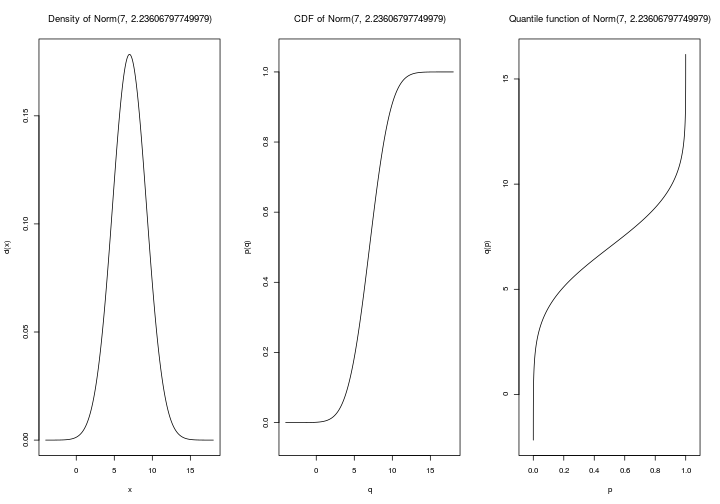# R objects : Numbers set.seed(1) U <- runif(20) U[1:4]  ##  0.2655087 0.3721239 0.5728534 0.9082078  U == 0.2655087  ##  FALSE  options(digits = 3) U[1:4]  ##  0.266 0.372 0.573 0.908  options(digits = 22) U[1:4]  ##  0.2655086631420999765396 0.3721238996367901563644 0.5728533633518964052200 ##  0.9082077899947762489319  options(digits = 3)  x <- exp(1) y <- x x <- 2 y  ##  2.72  class(x)  ##  "numeric"  0/0  ##  NaN  1/0  ##  Inf  .Machine$double.xmax

##  1.8e+308

2e+308<Inf

##  FALSE

(3/10-1/10)

##  0.2

(3/10-1/10)==(7/10-5/10)

##  FALSE

(3/10-1/10)-(7/10-5/10)

##  2.78e-17

all.equal((3/10-1/10),(7/10-5/10))

##  TRUE

sqrt(2)^2 == 2

##  FALSE

.Machine$double.eps  ##  2.22e-16  .Machine$double.xmin

##  2.23e-308

x <- rnorm(8)
names(x) <- letters[1:8]
x

##       a       b       c       d       e       f       g       h
##  1.5118  0.3898 -0.6212 -2.2147  1.1249 -0.0449 -0.0162  0.9438

x[2:4]

##      b      c      d
##  0.390 -0.621 -2.215

x[c("b","c","d")]

##      b      c      d
##  0.390 -0.621 -2.215

min(x[x>0])

##  0.39

x[-(3:8)]

##    a    b
## 1.51 0.39

# x[-3:8]
x[c(TRUE,FALSE)]

##       a       c       e       g
##  1.5118 -0.6212  1.1249 -0.0162

x[c(TRUE,NA,FALSE,TRUE)]

##      a   <NA>      d      e   <NA>      h
##  1.512     NA -2.215  1.125     NA  0.944

x

##    b
## 0.39

x[]

##  0.39

for(i in 1:2){
nom_var <- paste0("x", i)
assign(nom_var, rpois(5,7))
}
x1

##  9 4 8 6 9

x2

##  8 9 7 7 9

x <- runif(4)
x

##  0.0233 0.4772 0.7323 0.6927

x

##  0.0233 0.4772 0.7323 0.6927

library(pryr,verbose=FALSE,quietly=TRUE,warn.conflicts=FALSE)
x %<a-% runif(1)
x

##  0.478

x

##  0.861

x <- 1:2
x

##  0.245

rm("x")
x

## Error in eval(expr, envir, enclos): objet 'x' introuvable


# R objects : Integers

(x_num=c(1,6,10))

##   1  6 10

(x_int=c(1L,6L,10L))

##   1  6 10

object.size(x_num)

## 72 bytes

object.size(x_int)

## 56 bytes

typeof(x_num)

##  "double"

typeof(x_int)

##  "integer"

is.integer(x_num)

##  FALSE

is.integer(x_int)

##  TRUE

str(x_num)

##  num [1:3] 1 6 10

str(x_int)

##  int [1:3] 1 6 10

c(1,c(2,c(3,c(4,5))))

##  1 2 3 4 5


# R objects : Matrices

M<-1:24
dim(M)=c(6,4)
M

##      [,1] [,2] [,3] [,4]
## [1,]    1    7   13   19
## [2,]    2    8   14   20
## [3,]    3    9   15   21
## [4,]    4   10   16   22
## [5,]    5   11   17   23
## [6,]    6   12   18   24

str(M)

##  int [1:6, 1:4] 1 2 3 4 5 6 7 8 9 10 ...

colnames(M)=letters[1:4]
rownames(M)=LETTERS[10:15]
M

##   a  b  c  d
## J 1  7 13 19
## K 2  8 14 20
## L 3  9 15 21
## M 4 10 16 22
## N 5 11 17 23
## O 6 12 18 24

M["K",]

##  a  b  c  d
##  2  8 14 20

M[c("K","N"),]

##   a  b  c  d
## K 2  8 14 20
## N 5 11 17 23

M[c(2,5),]

##   a  b  c  d
## K 2  8 14 20
## N 5 11 17 23

M[c("K",5),]

## Error in M[c("K", 5), ]: indice hors limites

M[c(1,6),c(1,4)]

##   a  d
## J 1 19
## O 6 24

diag(M)

##   1  8 15 22

t(M)%*%M

##     a    b    c    d
## a  91  217  343  469
## b 217  559  901 1243
## c 343  901 1459 2017
## d 469 1243 2017 2791


On Windows machines

rm(list=ls())
memory.size()

## Warning: 'memory.size()' is Windows-specific

##  Inf

memory.limit()

## Warning: 'memory.limit()' is Windows-specific

##  Inf

memory.limit(size=8000)

## Warning: 'memory.limit()' is Windows-specific

##  Inf

memory.limit(size=4000)

## Warning: 'memory.limit()' is Windows-specific

##  Inf

x3 <- 1:1e3
x4 <- 1:1e4
x5 <- 1:1e5
x6 <- 1:1e6
object.size(x3)

## 4040 bytes

object.size(x4)

## 40040 bytes

object.size(x6)

## 4000040 bytes

z1 <- matrix(0,1e+06,3)
z2 <- matrix(as.integer(0),1e+06,3)
object.size(z1)

## 24000200 bytes

print(object.size(z1),units="Mb")

## 22.9 Mb

object.size(z2)

## 12000200 bytes

print(object.size(z2),units="Mb")

## 11.4 Mb

mydat_r <- matrix(rnorm(3*5e6,0,2),5e6,3)
colnames(mydat_r) <- c("first","second","third")
mydat_rdf <- as.data.frame(mydat_r)
print(object.size(mydat_rdf ),units="Mb")

## 114.4 Mb

library(biglm)

## Loading required package: DBI

z1 <- matrix(as.integer(5), 300, 400)
object.size(z1)

## 480200 bytes

library(bigmemory)

## Loading required package: bigmemory.sri

z2 <- big.matrix(300, 400, type="integer", init=5)
object.size(z2)

## 664 bytes

rm(list=ls())


# R objects : Factors

x <- c("A", "A", "B", "B", "C")
x

##  "A" "A" "B" "B" "C"

factor(x)

##  A A B B C
## Levels: A B C

relevel(factor(x),"B")

##  A A B B C
## Levels: B A C

model.matrix(~0+x)

##   xA xB xC
## 1  1  0  0
## 2  1  0  0
## 3  0  1  0
## 4  0  1  0
## 5  0  0  1
## attr(,"assign")
##  1 1 1
## attr(,"contrasts")
## attr(,"contrasts")$x ##  "contr.treatment"  n <- 10; nn <- 100 group <- factor(round(n * runif(n * nn))) x <- rnorm(n * nn) + sqrt(as.numeric(group)) tapply(x,group,mean)  ## 0 1 2 3 4 5 6 7 8 9 10 ## 1.08 1.35 1.73 2.11 2.28 2.50 2.79 2.69 3.04 3.13 3.23  aggregate(x,by=list(group),mean)  ## Group.1 x ## 1 0 1.08 ## 2 1 1.35 ## 3 2 1.73 ## 4 3 2.11 ## 5 4 2.28 ## 6 5 2.50 ## 7 6 2.79 ## 8 7 2.69 ## 9 8 3.04 ## 10 9 3.13 ## 11 10 3.23  xg <- split(x, group) sapply(xg, length)  ## 0 1 2 3 4 5 6 7 8 9 10 ## 54 101 93 105 103 101 99 100 93 107 44  sapply(xg, mean)  ## 0 1 2 3 4 5 6 7 8 9 10 ## 1.08 1.35 1.73 2.11 2.28 2.50 2.79 2.69 3.04 3.13 3.23  library(pbapply,verbose=FALSE,quietly=TRUE,warn.conflicts=FALSE) n <- 10; nn <- 1e6 group <- factor(round(n * runif(n * nn))) x <- rnorm(n * nn) + sqrt(as.numeric(group)) xg <- split(x, group) pbsapply(xg, mean)  ## 0 1 2 3 4 5 6 7 8 9 10 ## 1.00 1.41 1.73 2.00 2.24 2.45 2.65 2.83 3.00 3.16 3.32  x <- factor(c("b","a","b")) levels(x)  ##  "a" "b"  x="c"  ## Warning in [<-.factor(*tmp*, 3, value = "c"): invalid factor level, NA ## generated  x  ##  b a <NA> ## Levels: a b  factor(x,levels=c("b","a"))  ##  b a <NA> ## Levels: b a  x  ##  b ## Levels: a b  x[1,drop=TRUE]  ##  b ## Levels: b  U <- runif(20) cut(U,breaks=2)  ##  (0.515,0.966] (0.515,0.966] (0.0631,0.515] (0.0631,0.515] (0.515,0.966] ##  (0.0631,0.515] (0.0631,0.515] (0.0631,0.515] (0.515,0.966] (0.515,0.966] ##  (0.0631,0.515] (0.515,0.966] (0.0631,0.515] (0.515,0.966] (0.0631,0.515] ##  (0.515,0.966] (0.515,0.966] (0.515,0.966] (0.0631,0.515] (0.0631,0.515] ## Levels: (0.0631,0.515] (0.515,0.966]  cut(U,breaks=2,labels=c("small","large"))  ##  large large small small large small small small large large small large ##  small large small large large large small small ## Levels: small large  cut(U,breaks=c(0,.3,.8,1),labels=c("small","medium","large"))  ##  large large small medium large medium small small medium medium ##  medium medium small large medium medium medium medium small small ## Levels: small medium large  table(cut(U,breaks=c(0,.3,.8,1),labels=c("small","medium","large")))  ## ## small medium large ## 6 10 4  ## Short Quizz What would 1:3*1:5 return ? 1. 1 2 3 2. 1 2 3 2 4 6 3 6 9 4 8 12 5 10 15 3. 1 4 9 4 10 4. 1 2 3 4 5 2 4 6 8 10 3 6 9 12 15 a*b returns the componentwise product of a and b 1:3*1:5 is read (1:3)*(1:5) which returns the componentwise product of 1:3 - or c(1,2,3) - and 1:5 - or c(1,2,3,4,5). ## Short Quizz What would intersect(seq(4,28,by=7),seq (31,3,by=-2)) return ? 1. 11 25 2. 25 11 3. 3 5 7 9 11 13 15 17 19 21 23 25 27 29 31 4. FALSE See seq(4,28,by=7) %in% seq(3,31,by=2) seq(4,28,by=7)[seq(4,28,by=7) %in% seq(3,31,by=2)]. ## Short Quizz Consider function dg <- function(M) diag(M[,ncol(M):1]). Define M <- matrix(1:25,5,5). What would dg(M) return ? 1. 5 9 13 17 21 2. 21 17 13 9 5 3. 1 2 3 4 5 4. 1 7 13 19 25 See dg return the second diagonal of the matrix (watch the order). dgreturn the second diagonal of the matrix, starting from the upper right corner (21) and ending with the lower left corner (5). ## Short Quizz Define M <- matrix(1:5 ,3 ,3) What would M[,2] return ? 1. 4 5 2. 4 5 NA 3. 4 5 NULL 4. 4 5 1 Observe that M <- matrix(c(1:5,1:4),3,3). M <- matrix(c(1:5,1:4),3,3). ## Short Quizz 1. Compute $\displaystyle{\sum_{i=10}^{20}\left(i^2+\frac{4}{i}\right)}$ (closest integer) 2. Compute $\displaystyle{\sum_{i\in\{10,15,20\}}\left(i^2+\frac{4}{i}\right)}$ (closest integer) 1. 2588 2. 724 Use sum(i^2-4/i) and the round Set i=10:20 and i=c(10,15,20) and compute sum(i^2+4/i). ## Short Quizz Solve $\left\lbrace \begin{array}{l} 3x+2y-z=1 \\ 2x-2y+4z=-2 \\ -x+y/2-z=0 \end{array} \right.$ 1. $x$ 2. $y$ 3. $z$ 1. 1 2. -2 3. 0 Use solve(A,b). Set A <- matrix(c(3,2,-1,2,-2,.5,-1,4,-1),3,3) and b <- c(1,-2,0) and then compute solve(A,b). ## Short Quizz Compute $\displaystyle{\sum_{i=1}^{10}\sum_{j=i}^{10} \frac{i^2}{5+i\cdot j}}$ 1. 32.21865 2. 27.71865 3. 2.5 4. 6.5 Use a double loop for(i in 1:10) for(j in i:10). Set s <- 0 and then compute for(i in 1:10) for(j in i:10) s <- s+i^2/(5*i*j). ## Short Quizz What would sqrt(7)^2 == 7 return ? 1. TRUE 2. FALSE 3. NA 4. NULL Compute sqrt(7)^2 - 7. Use all.equal(sqrt(7)^2, 7) to avoid numerical issue like that one. ## Short Quizz Find the two roots of polynomial $x^2+x=1$ (with 3 digits ) 1. $x_1$ ($x_1<0$) 2. $x_2$ ($x_1>0$) 1. -0.618 2. 1.618 See Re(polyroot(c(1,1,-1))) or uniroot(f,c(-10,0))$root and uniroot(f,c(0,10))$root ## Short Quizz Use function recode in library(car) to change levels of x into "small" (for "a") and "large" (for "b") ## Short Quizz Use function recode in library(car) to change merge levels "small" and "medium" into "not large" for U # R objects : Strings and Characters "Be carefull of 'quotes'" 'Be carefull of "quotes"'  cities <- c("New York, NY", "Los Angeles, CA", "Boston, MA") substr(cities, nchar(cities)-1, nchar(cities))  ##  "NY" "CA" "MA"  unlist(strsplit(cities, ", "))[seq(2,6,by=2)]  ##  "NY" "CA" "MA"  library(stringr,verbose=FALSE,quietly=TRUE,warn.conflicts=FALSE) tweet = "Emerging #climate change, environment and sustainability concerns for #actuaries Apr 9 #Toronto. Register TODAY http://bit.ly/CIAClimateForum" hash="#[a-zA-Z]{1,}" str_extract(tweet,hash)  ##  "#climate"  str_extract_all(tweet,hash)  ## [] ##  "#climate" "#actuaries" "#Toronto"  str_locate_all(tweet,hash)  ## [] ## start end ## [1,] 10 17 ## [2,] 71 80 ## [3,] 88 95  urls="http://([\\da-z\\.-]+)\\.([a-z\\.]{2,6})/[a-zA-Z0-9]{1,}" str_extract_all(tweet,urls)  ## [] ##  "http://bit.ly/CIAClimateForum"  email="^([a-z0-9_\\.-]+)@([\\da-z\\.-]+)\\.([a-z\\.]{2,6})$"
grep(pattern = email, x = "charpentier.arthur@uqam.ca")

##  1

grepl(pattern = email, x = "charpentier.arthur@uqam.ca")

##  TRUE

str_detect(pattern = email, string=c("charpentier.arthur@uqam.ca","@freakonometrics"))

##   TRUE FALSE

ex_sentence = "This is 1 simple sentence, just to play with, then we'll play with 4, and that will be more difficult"
library(tm,verbose=FALSE,quietly=TRUE,warn.conflicts=FALSE)
ex_corpus <- Corpus(VectorSource(ex_sentence))
ex_corpus

## <<VCorpus>>
## Metadata:  corpus specific: 0, document level (indexed): 0
## Content:  documents: 1

inspect(ex_corpus)

## <<VCorpus>>
## Metadata:  corpus specific: 0, document level (indexed): 0
## Content:  documents: 1
##
## []
## <<PlainTextDocument>>
## Content:  chars: 101

grep("hard", ex_sentence)

## integer(0)

grep("difficult", ex_sentence)

##  1

library(stringr,verbose=FALSE,quietly=TRUE,warn.conflicts=FALSE)
word(ex_sentence,4)

##  "simple"

word(ex_sentence,1:20)

##   "This"      "is"        "1"         "simple"    "sentence," "just"
##   "to"        "play"      "with,"     "then"      "we'll"     "play"
##  "with"      "4,"        "and"       "that"      "will"      "be"
##  "more"      "difficult"

ex_words <- strsplit(ex_sentence, split=" ")[]
ex_words

##   "This"      "is"        "1"         "simple"    "sentence," "just"
##   "to"        "play"      "with,"     "then"      "we'll"     "play"
##  "with"      "4,"        "and"       "that"      "will"      "be"
##  "more"      "difficult"

grep(pattern="w",ex_words,value=TRUE)

##  "with," "we'll" "with"  "will"

str_count(ex_words,"w")

##   0 0 0 0 0 0 0 0 1 0 1 0 1 0 0 0 1 0 0 0

grep(pattern="[ai]",ex_words,value=TRUE)

##   "This"      "is"        "simple"    "play"      "with,"     "play"
##   "with"      "and"       "that"      "will"      "difficult"

grep(pattern="[[:punct:]]",ex_words,value=TRUE)

##  "sentence," "with,"     "we'll"     "4,"

require(wordcloud)
wordcloud(ex_corpus)cols<-colorRampPalette(c("lightgrey","blue"))(40)
wordcloud(words = ex_corpus, max.words = 40, random.order=FALSE, scale = c(5, 0.5), colors=cols)tdm <- TermDocumentMatrix(ex_corpus)
tdm

## <<TermDocumentMatrix (terms: 14, documents: 1)>>
## Non-/sparse entries: 14/0
## Sparsity           : 0%
## Maximal term length: 9
## Weighting          : term frequency (tf)

inspect(tdm)

## <<TermDocumentMatrix (terms: 14, documents: 1)>>
## Non-/sparse entries: 14/0
## Sparsity           : 0%
## Maximal term length: 9
## Weighting          : term frequency (tf)
##
##            Docs
## Terms       1
##   and       1
##   difficult 1
##   just      1
##   more      1
##   play      2
##   sentence, 1
##   simple    1
##   that      1
##   then      1
##   this      1
##   we'll     1
##   will      1
##   with      1
##   with,     1

inspect(ex_corpus)

## <<VCorpus>>
## Metadata:  corpus specific: 0, document level (indexed): 0
## Content:  documents: 1
##
## []
## <<PlainTextDocument>>
## Content:  chars: 101

fix_contractions <- function(doc) {
doc <- gsub("won't", "will not", doc)
doc <- gsub("n't", " not", doc)
doc <- gsub("'ll", " will", doc)
doc <- gsub("'re", " are", doc)
doc <- gsub("'ve", " have", doc)
doc <- gsub("'m", " am", doc)
doc <- gsub("'s", "", doc)
return(doc)
}

ex_corpus <- tm_map(ex_corpus, fix_contractions)
inspect(ex_corpus)

## <<VCorpus>>
## Metadata:  corpus specific: 0, document level (indexed): 0
## Content:  documents: 1
##
## []
##  This is 1 simple sentence, just to play with, then we will play with 4, and that will be more difficult

ex_corpus <- tm_map(ex_corpus, tolower)
inspect(ex_corpus)

## <<VCorpus>>
## Metadata:  corpus specific: 0, document level (indexed): 0
## Content:  documents: 1
##
## []
##  this is 1 simple sentence, just to play with, then we will play with 4, and that will be more difficult

ex_corpus <- tm_map(ex_corpus, removeNumbers)
inspect(ex_corpus)

## <<VCorpus>>
## Metadata:  corpus specific: 0, document level (indexed): 0
## Content:  documents: 1
##
## []
##  this is  simple sentence, just to play with, then we will play with , and that will be more difficult

ex_corpus <- tm_map(ex_corpus,gsub, pattern= "[[:punct:]]", replacement = " ")
inspect(ex_corpus)

## <<VCorpus>>
## Metadata:  corpus specific: 0, document level (indexed): 0
## Content:  documents: 1
##
## []
##  this is  simple sentence  just to play with  then we will play with   and that will be more difficult

stopwords("en")[sample(1:length(stopwords("en")),size=10)]

##   "that's"    "as"        "too"       "shouldn't" "doesn't"   "does"
##   "few"       "what's"    "yourself"  "we're"

inspect(ex_corpus)

## <<VCorpus>>
## Metadata:  corpus specific: 0, document level (indexed): 0
## Content:  documents: 1
##
## []
##  this is  simple sentence  just to play with  then we will play with   and that will be more difficult

library(SnowballC,verbose=FALSE,quietly=TRUE,warn.conflicts=FALSE)
ex_corpus <- tm_map(ex_corpus, stemDocument)
inspect(ex_corpus)

## <<VCorpus>>
## Metadata:  corpus specific: 0, document level (indexed): 0
## Content:  documents: 1
##
## []
##  this is  simple sentence  just to play with  then we will play with   and that will be more difficult

wordcloud(ex_corpus[])wordcloud(words = ex_corpus[], max.words = 40, random.order=FALSE, scale = c(5, 0.5),colors=cols)## Short Quizz

What would grep("ab",c("abc","b","a","ba","cab")) return ?

1. TRUE
2. 1 5
3. abc cab
4. TRUE FALSE FALSE FALSE TRUE

Use ?grep.

only two elements contain ab in that order.

## Short Quizz

What would the following command return ?

1. paste("a",c("b c","d"",sep="")
2. paste("a",c("b c","d"",collapse="")
2. "a b ca d"

See ?paste

# R objects : Dates

some.dates <- as.Date(c("16/10/12","19/11/12"),format="%d/%m/%y")
diff(some.dates)

## Time difference of 34 days

sequence.date <- seq(from=some.dates,to=some.dates,by=7)
format(sequence.date,"%b")

##  "oct." "oct." "oct." "nov." "nov."

months(sequence.date)

##  "octobre"  "octobre"  "octobre"  "novembre" "novembre"

weekdays(some.dates)

##  "mardi" "lundi"

substr(as.POSIXct(some.dates), 1, 4)

##  "2012" "2012"

strftime(some.dates,"%Y")

##  "2012" "2012"

Sys.setlocale("LC_TIME", "fr_FR")

## Warning in Sys.setlocale("LC_TIME", "fr_FR"): la requête OS pour spécifier la
## localisation à "fr_FR" n'a pas pu être honorée

##  ""

months(sequence.date)

##  "octobre"  "octobre"  "octobre"  "novembre" "novembre"


Dates with R

# R objects : Lists

x <- list(1:5,c(1,2,3,4,5),a="test",
b=c(TRUE,FALSE),rpois(5,8))
x

## []
##  1 2 3 4 5
##
## []
##  1 2 3 4 5
##
## $a ##  "test" ## ##$b
##   TRUE FALSE
##
## []
##  7 7 8 7 8

str(x)

## List of 5
##  $: int [1:5] 1 2 3 4 5 ##$  : num [1:5] 1 2 3 4 5
##  $a: chr "test" ##$ b: logi [1:2] TRUE FALSE
##  $: int [1:5] 7 7 8 7 8  x <- list( list(1:5,c(1,2,3,4,5)),a="test", b=c(TRUE,FALSE),rpois(5,8)) x  ## [] ## [][] ##  1 2 3 4 5 ## ## [][] ##  1 2 3 4 5 ## ## ##$a
##  "test"
##
## $b ##  TRUE FALSE ## ## [] ##  8 9 6 5 3  names(x)  ##  "" "a" "b" ""  str(x)  ## List of 4 ##$  :List of 2
##   ..$: int [1:5] 1 2 3 4 5 ## ..$ : num [1:5] 1 2 3 4 5
##  $a: chr "test" ##$ b: logi [1:2] TRUE FALSE
##  $: int [1:5] 8 9 6 5 3  is.list(x)  ##  TRUE  is.recursive(x)  ##  TRUE  x[]  ##  TRUE FALSE  x[["b"]]  ##  TRUE FALSE  x[]  ## [] ##  1 2 3 4 5 ## ## [] ##  1 2 3 4 5  x[][]  ##  1 2 3 4 5  ## Short Quizz What would length(x) return ? 1. NULL 2. 1 3. 4 4. 5 Use ?list. one can get x[] up to x[] (but not x[]) f <- function(x) { return(x*(1-x)) } optim.f <- optimize(f, interval=c(0, 1), maximum=TRUE) str(optim.f)  ## List of 2 ##$ maximum  : num 0.5
##  $objective: num 0.25  list(abc=1)$a

##  1

list(abc=1,a=2)$a  ##  2  list(bac=1)$a

## NULL

list(abc=1,b=2)$a  ##  1  # R objects : Functions factorial  ## function (x) ## gamma(x + 1) ## <bytecode: 0x13e51a90> ## <environment: namespace:base>  gamma  ## function (x) .Primitive("gamma")  ?gamma  # If Condition set.seed(1) u <- runif(1) if(u>.5) {("greater than 50%")} else {("smaller than 50%")}  ##  "smaller than 50%"  ifelse(u>.5,("greater than 50%"),("smaller than 50%"))  ##  "smaller than 50%"  u  ##  0.266  u <- runif(3) if(u>.5) {print("greater than 50%")} else {("smaller than 50%")}  ## Warning in if (u > 0.5) {: la condition a une longueur > 1 et seul le premier ## élément est utilisé  ##  "smaller than 50%"  ifelse(u>.5,("greater than 50%"),("smaller than 50%"))  ##  "smaller than 50%" "greater than 50%" "greater than 50%"  u  ##  0.372 0.573 0.908  # Loops v_x <- runif(1e5) sqrt_x <- NULL for(x in v_x) sqrt_x <- c(sqrt_x,sqrt(x)) sqrt_x <- NULL system.time(for(x in v_x) sqrt_x <- c(sqrt_x,sqrt(x)))  ## user system elapsed ## 13.916 0.084 14.001  sqrt_x <- numeric(length(v_x)) for(i in seq_along(v_x)) sqrt_x[i] <- sqrt(v_x[i]) sqrt_x <- numeric(length(v_x)) system.time(for(i in seq_along(v_x)) sqrt_x[i] <- sqrt(v_x[i]))  ## user system elapsed ## 0.128 0.000 0.130  system.time(Vectorize(sqrt)(v_x))  ## user system elapsed ## 0.004 0.000 0.002  sqrt_x <- sapply(v_x,sqrt) sqrt_x <- unlist(lapply(v_x,sqrt)) system.time(unlist(lapply(v_x,sqrt)))  ## user system elapsed ## 0.040 0.000 0.037  library(parallel,verbose=FALSE,quietly=TRUE,warn.conflicts=FALSE) (allcores <- detectCores(all.tests=TRUE))  ##  4  sqrt_x <- unlist(mclapply(v_x,sqrt,mc.cores=4)) system.time(unlist(mclapply(v_x,sqrt,mc.cores=4)))  ## user system elapsed ## 0.096 0.116 0.077  f<-function(x) dlnorm(x) integrate(f,0,Inf)  ## 1 with absolute error < 2.5e-07  integrate(f,0,1e5)  ## 1.82e-05 with absolute error < 3.6e-05  integrate(f,0,1e3)$value+integrate(f,1e3,1e5)$value  ##  1  # Apply see data camp on apply rN.Poisson <- function(n) rpois(n,5) rX.Exponential <- function(n) rexp(n,2) set.seed(1) rN.Poisson(5)  ##  4 4 5 8 3  rX.Exponential(3)  ##  0.229 0.129 1.447  rcpd1 <- function(n,rN=rN.Poisson,rX=rX.Exponential){ V <- rep(0,n) for(i in 1:n){ N <- rN(1) if(N>0){V[i] <- sum(rX(N))} } return(V)} rcpd1(3)  ##  1.52 3.88 0.79  rcpd1 <- function(n,rN=rN.Poisson,rX=rX.Exponential){ V <- rep(0,n) for (i in 1:n) V[i] <- sum(rX(rN(1))) return(V)} rcpd1(3)  ##  0.893 3.368 3.023  rcpd2 <- function(n,rN=rN.Poisson,rX=rX.Exponential){ N <- rN(n) X <- rX(sum(N)) I <- factor(rep(1:n,N),levels=1:n) return(as.numeric(xtabs(X ~ I)))} rcpd2(3)  ##  2.29 2.06 5.46  rcpd3 <- function(n,rN=rN.Poisson,rX=rX.Exponential){ N <- rN(n) X <- rX(sum(N)) I <- factor(rep(1:n,N),levels=1:n) V <- tapply(X,I,sum) V[is.na(V)] <- 0 return(as.numeric(V))} rcpd3(3)  ##  2.22 6.03 4.33  rcpd4 <- function(n,rN=rN.Poisson,rX=rX.Exponential){ return(sapply(rN(n), function(x) sum(rX(x))))} rcpd4(3)  ##  2.83 4.09 2.99  rcpd5 <- function(n,rN=rN.Poisson,rX=rX.Exponential){ return(sapply(Vectorize(rX)(rN(n)),sum))} rcpd5(3)  ##  1.81 3.69 3.60  rcpd6 <- function(n,rN=rN.Poisson,rX=rX.Exponential){ return(unlist(lapply(lapply(t(rN(n)),rX),sum)))} rcpd6(3)  ##  0.291 1.344 2.948  n <- 100 library(microbenchmark,verbose=FALSE,quietly=TRUE,warn.conflicts=FALSE) options(digits=1) microbenchmark(rcpd1(n),rcpd2(n),rcpd3(n),rcpd4(n),rcpd5(n),rcpd6(n))  ## Unit: microseconds ## expr min lq mean median uq max neval cld ## rcpd1(n) 480 530 605 610 665 1001 100 b ## rcpd2(n) 1002 1106 1217 1196 1268 2133 100 c ## rcpd3(n) 477 528 584 565 605 1277 100 b ## rcpd4(n) 308 367 393 399 418 551 100 a ## rcpd5(n) 387 456 603 524 561 8790 100 b ## rcpd6(n) 313 357 399 407 431 592 100 a  options(digits=5)  # Vectorization f <- function(x,m=0,s=1){ H<-function(t) 1-pnorm(t,m,s) integral<-integrate(H,lower=x,upper=Inf)$value
res<-H(x)/integral
return(res)
}
f(0)

##  1.2533

f(0:1)

## Warning in if (is.finite(lower)) {: la condition a une longueur > 1 et seul le
## premier élément est utilisé

##  1.25331 0.39769

y <- rep(NA,2)
x <- 0:1
for(i in 1:2) y[i] <- f(x[i])
y

##  1.2533 1.9043

sapply(x,"f")

##  1.2533 1.9043

Vectorize(f)(x)

##  1.2533 1.9043

binorm <- function(x1,x2,r=0){
exp(-(x1^2+x2^2-2*r*x1*x2)/(2*(1-r^2)))/(2*pi*sqrt(1-r^2))
}
u <- seq(-2,2)
binorm(u,u)

##  0.002915 0.058550 0.159155 0.058550 0.002915

outer(u,u,binorm)

##          [,1]     [,2]     [,3]     [,4]     [,5]
## [1,] 0.002915 0.013064 0.021539 0.013064 0.002915
## [2,] 0.013064 0.058550 0.096532 0.058550 0.013064
## [3,] 0.021539 0.096532 0.159155 0.096532 0.021539
## [4,] 0.013064 0.058550 0.096532 0.058550 0.013064
## [5,] 0.002915 0.013064 0.021539 0.013064 0.002915

(uv<-expand.grid(u,u))

##    Var1 Var2
## 1    -2   -2
## 2    -1   -2
## 3     0   -2
## 4     1   -2
## 5     2   -2
## 6    -2   -1
## 7    -1   -1
## 8     0   -1
## 9     1   -1
## 10    2   -1
## 11   -2    0
## 12   -1    0
## 13    0    0
## 14    1    0
## 15    2    0
## 16   -2    1
## 17   -1    1
## 18    0    1
## 19    1    1
## 20    2    1
## 21   -2    2
## 22   -1    2
## 23    0    2
## 24    1    2
## 25    2    2

matrix(binorm(uv$Var1,uv$Var2),5,5)

##          [,1]     [,2]     [,3]     [,4]     [,5]
## [1,] 0.002915 0.013064 0.021539 0.013064 0.002915
## [2,] 0.013064 0.058550 0.096532 0.058550 0.013064
## [3,] 0.021539 0.096532 0.159155 0.096532 0.021539
## [4,] 0.013064 0.058550 0.096532 0.058550 0.013064
## [5,] 0.002915 0.013064 0.021539 0.013064 0.002915

u <- seq(-2,2,length=31)
persp(u,u,outer(u,u,binorm),theta=25)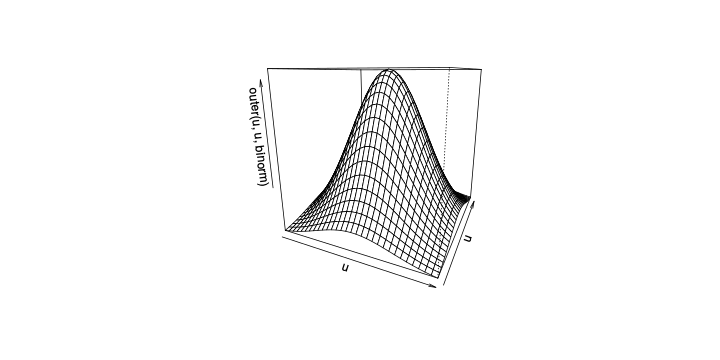# Computational Efficiency

library(microbenchmark,verbose=FALSE,quietly=TRUE,warn.conflicts=FALSE)
n <- 1000
A<-matrix(seq(1,n^2),n,n)
B<-matrix(seq(1,n^2),n,n)
C<-1:n
microbenchmark((A%*%B)%*%C,A%*%(B%*%C),replications=1)

## Unit: nanoseconds
##             expr       min        lq       mean    median        uq       max
##  (A %*% B) %*% C 868088560 881443798 8.9133e+08 888537215 896653321 972622868
##  A %*% (B %*% C)   9199145   9315150 1.0849e+07   9473058  11095620  22345401
##     replications         5        17 2.6341e+02       270       342      1179
##  neval cld
##    100   c
##    100  b
##    100 a

m <- 1000
n <- 50
X <- matrix(rnorm(m * n, mean = 10, sd = 3), nrow = m)
group <- rep(c("A","B"), each = n / 2)

system.time(for(i in 1:m) t.test(X[i, ] ~ group)$stat)  ## user system elapsed ## 0.936 0.000 0.939  system.time(for(i in 1:m) t.test(X[i, group == "A"], X[i, group == "B"])$stat)

##    user  system elapsed
##   0.196   0.000   0.195

t.test_1 <- function(x, grp){
t.test(x[ group == "A"], x[ group == "B"])$stat } system.time(t1 <- apply(X, 1, t.test_1, grp = grp))  ## user system elapsed ## 0.204 0.000 0.204  t.test_2 <- function(x, grp) { t_stat <- function(x) { m <- mean(x) n <- length(x) var <- sum((x - m) ^ 2) / (n - 1) list(m = m, n = n, var = var) } g1 <- t_stat(x[grp == "A"]) g2 <- t_stat(x[grp == "B"]) se_total <- sqrt(g1$var / g1$n + g2$var / g2$n) (g1$m - g2$m) / se_total } system.time(apply(X, 1, t.test_2, grp = group))  ## user system elapsed ## 0.04 0.00 0.04  t.test_3 <- function(X, grp){ t_stat <- function(X) { m <- rowMeans(X) n <- ncol(X) var <- rowSums((X - m) ^ 2) / (n - 1) list(m = m, n = n, var = var) } g1 <- t_stat(X[, grp == "A"]) g2 <- t_stat(X[, grp == "B"]) se_total <- sqrt(g1$var / g1$n + g2$var / g2$n) (g1$m - g2$m) / se_total } system.time( t.test_3(X, group))  ## user system elapsed ## 0.004 0.000 0.002  "%pm%" <- function(x,s) x + c(qnorm(.05),qnorm(.95))*s 100 %pm% 10  ##  83.551 116.449  'second<-' <- function(x, value) { x <- value return(x) } x <- 1:10 second(x) <- 5 x  ##  1 5 3 4 5 6 7 8 9 10  library(pryr,verbose=FALSE,quietly=TRUE,warn.conflicts=FALSE) x <- 1:10 address(x)  ##  "0x16c817a8"  second(x) <- 6 address(x)  ##  "0xe5a7790"  ls(globalenv())  ##  "A" "allcores" "B" "binorm" ##  "C" "cities" "cols" "email" ##  "ex_corpus" "ex_sentence" "ex_words" "f" ##  "fix_contractions" "group" "hash" "i" ##  "m" "n" "nn" "optim.f" ##  "%pm%" "rcpd1" "rcpd2" "rcpd3" ##  "rcpd4" "rcpd5" "rcpd6" "rN.Poisson" ##  "rX.Exponential" "second<-" "sequence.date" "some.dates" ##  "sqrt_x" "t1" "tdm" "t.test_1" ##  "t.test_2" "t.test_3" "tweet" "u" ##  "U" "urls" "uv" "v_x" ##  "x" "X" "xg" "y"  find("x")  ## Error: is_osmar(object) n'est pas TRUE  find("pi")  ## Error: is_osmar(object) n'est pas TRUE  environment(sd)  ## <environment: 0x52dd5e8>  f <- function(x) x^2 f  ## function(x) x^2  formals(f)  ##$x

body(f)

## x^2

e <- new.env()
e$d <- 1 e$f <- 1:5
e$g <- 1:5 ls(e)  ##  "d" "f" "g"  str(e)  ## <environment: 0xe411780>  identical(globalenv(),e)  ##  FALSE  search()  ##  ".GlobalEnv" "package:microbenchmark" ##  "package:parallel" "package:SnowballC" ##  "package:stringr" "package:pbapply" ##  "package:bigmemory" "package:bigmemory.sri" ##  "package:biglm" "package:DBI" ##  "package:SparseM" "package:osmar" ##  "package:geosphere" "package:sp" ##  "package:RCurl" "package:bitops" ##  "package:XML" "package:data.table" ##  "package:VGAM" "package:splines" ##  "package:stats4" "package:tm" ##  "package:NLP" "package:wordcloud" ##  "package:RColorBrewer" "package:plyr" ##  "package:pryr" "package:distrEx" ##  "package:distr" "package:SweaveListingUtils" ##  "package:sfsmisc" "package:startupmsg" ##  "package:leaflet" "package:dplyr" ##  "package:htmlwidgets" "package:knitr" ##  "package:stats" "package:graphics" ##  "package:grDevices" "package:utils" ##  "package:datasets" "package:methods" ##  "Autoloads" "package:base"  f1 <- function(x1){ f2 <- function(x2){ f3 <- function(x3){ x1+x2+x3 } f3(3) } f2(2) } f1(1)  ##  6  f <- function(x) log(x) f("x")  ## Error in log(x): argument non numérique pour une fonction mathématique  try(f("x")) inherits(try(f("x")), "try-error")  ##  TRUE  inherits(try(f("x"), silent=TRUE), "try-error")  ##  TRUE  a=0 try(a<-f("x"), silent=TRUE) a  ##  0  try(a<-f(x), silent=TRUE) a  ##  0.0000 1.7918 1.0986 1.3863 1.6094 1.7918 1.9459 2.0794 2.1972 2.3026  x=1:10 g=function(f) f(x) g(mean)  ##  5.5  fibonacci <- function(n){ if(n<2) return(1) fibonacci(n-2)+fibonacci(n-1) } fibonacci(20)  ##  10946  system.time(fibonacci(30))  ## user system elapsed ## 1.964 0.000 1.964  library(memoise,verbose=FALSE,quietly=TRUE,warn.conflicts=FALSE) fibonacci <- memoise(function(n){ if(n<2) return(1) fibonacci(n-2)+fibonacci(n-1) }) system.time(fibonacci(30))  ## user system elapsed ## 0.004 0.000 0.006  set.seed(1) x<-rexp(6) sum(x)  ##  5.5534  .Primitive("sum")(x)  ##  5.5534  library(Rcpp,verbose=FALSE,quietly=TRUE,warn.conflicts=FALSE) cppFunction('double sum_C(NumericVector x) { int n = x.size(); double total = 0; for(int i = 0; i < n; ++i) { total += x[i]; } return total; }') sum_C(x)  ##  5.5534  Rcpp package. library(microbenchmark,verbose=FALSE,quietly=TRUE,warn.conflicts=FALSE) x <- runif(1e6) microbenchmark( sum(x), .Primitive("sum")(x), sum_C(x))  ## Unit: milliseconds ## expr min lq mean median uq max neval cld ## sum(x) 1.1850 1.2090 1.2256 1.2176 1.2316 1.4749 100 a ## .Primitive("sum")(x) 1.1880 1.2112 1.2354 1.2191 1.2344 2.0989 100 a ## sum_C(x) 1.1787 1.1928 1.2225 1.2025 1.2174 1.9491 100 a  names_list <- function(...){ names(list(...)) } names_list(a=5,b=7)  ##  "a" "b"  power <- function(exponent) { function(x) { x ^ exponent } } square <- power(2) square(4)  ##  16  cube <- power(3) cube(4)  ##  64  cube  ## function(x) { ## x ^ exponent ## } ## <environment: 0x13a4a7f0>  total <- 20 pb <- txtProgressBar(min = 0, max = total, style = 3)  ## | | | 0%  for(i in 1:(10*total)){ Sys.sleep(0.01) if(i %%10 == 0) setTxtProgressBar(pb, i%/%10) }  ## | |==== | 5% | |======= | 10% | |========== | 15% | |============== | 20% | |================== | 25% | |===================== | 30% | |======================== | 35% | |============================ | 40% | |================================ | 45% | |=================================== | 50% | |====================================== | 55% | |========================================== | 60% | |============================================== | 65% | |================================================= | 70% | |==================================================== | 75% | |======================================================== | 80% | |============================================================ | 85% | |=============================================================== | 90% | |================================================================== | 95% | |======================================================================| 100%  close(pb)  # R objects : Data Frames df <- data.frame(x=1:3,y=letters[1:3]) str(df)  ## 'data.frame': 3 obs. of 2 variables: ##$ x: int  1 2 3
##  $y: Factor w/ 3 levels "a","b","c": 1 2 3  typeof(df)  ##  "list"  class(df)  ##  "data.frame"  cbind(df,z=9:7)  ## x y z ## 1 1 a 9 ## 2 2 b 8 ## 3 3 c 7  df$z<-5:3
df

##   x y z
## 1 1 a 5
## 2 2 b 4
## 3 3 c 3

df

##   x
## 1 1
## 2 2
## 3 3

df[,1,drop=FALSE]

##   x
## 1 1
## 2 2
## 3 3

df[,1,drop=TRUE]

##  1 2 3

df[]

##  1 2 3

dfx  ##  1 2 3  df[,"x"]  ##  1 2 3  df[["x"]]  ##  1 2 3  set.seed(1) df[sample(nrow(df)),]  ## x y z ## 1 1 a 5 ## 3 3 c 3 ## 2 2 b 4  set.seed(1) df[sample(nrow(df),nrow(df)*2,replace=TRUE),]  ## x y z ## 1 1 a 5 ## 2 2 b 4 ## 2.1 2 b 4 ## 3 3 c 3 ## 1.1 1 a 5 ## 3.1 3 c 3  subset(df, x>1)  ## x y z ## 2 2 b 4 ## 3 3 c 3  set.seed(123) df <- data.frame(Y=rnorm(50), X1=as.factor(sample(LETTERS[1:4],size=50, replace=TRUE)), X2=as.factor(sample(1:3,size=50,replace=TRUE))) tail(df)  ## Y X1 X2 ## 45 1.207962 D 3 ## 46 -1.123109 A 2 ## 47 -0.402885 A 2 ## 48 -0.466655 A 2 ## 49 0.779965 A 1 ## 50 -0.083369 C 2  reg <- lm(Y~X1+X2,data=df) model.matrix(reg)[45:50,]  ## (Intercept) X1B X1C X1D X22 X23 ## 45 1 0 0 1 0 1 ## 46 1 0 0 0 1 0 ## 47 1 0 0 0 1 0 ## 48 1 0 0 0 1 0 ## 49 1 0 0 0 0 0 ## 50 1 0 1 0 1 0  reg <- lm(Y~(X1=="A")+X2,data=df) model.matrix(reg)[45:50,]  ## (Intercept) X1 == "A"TRUE X22 X23 ## 45 1 0 0 1 ## 46 1 1 1 0 ## 47 1 1 1 0 ## 48 1 1 1 0 ## 49 1 1 0 0 ## 50 1 0 1 0  reg <- lm(Y~X1*X2,data=df) model.matrix(reg)[45:50,]  ## (Intercept) X1B X1C X1D X22 X23 X1B:X22 X1C:X22 X1D:X22 X1B:X23 X1C:X23 ## 45 1 0 0 1 0 1 0 0 0 0 0 ## 46 1 0 0 0 1 0 0 0 0 0 0 ## 47 1 0 0 0 1 0 0 0 0 0 0 ## 48 1 0 0 0 1 0 0 0 0 0 0 ## 49 1 0 0 0 0 0 0 0 0 0 0 ## 50 1 0 1 0 1 0 0 1 0 0 0 ## X1D:X23 ## 45 1 ## 46 0 ## 47 0 ## 48 0 ## 49 0 ## 50 0  reg <- lm(Y~X1+X2%in%X1,data=df) model.matrix(reg)[45:50,]  ## (Intercept) X1B X1C X1D X1A:X22 X1B:X22 X1C:X22 X1D:X22 X1A:X23 X1B:X23 ## 45 1 0 0 1 0 0 0 0 0 0 ## 46 1 0 0 0 1 0 0 0 0 0 ## 47 1 0 0 0 1 0 0 0 0 0 ## 48 1 0 0 0 1 0 0 0 0 0 ## 49 1 0 0 0 0 0 0 0 0 0 ## 50 1 0 1 0 0 0 1 0 0 0 ## X1C:X23 X1D:X23 ## 45 0 1 ## 46 0 0 ## 47 0 0 ## 48 0 0 ## 49 0 0 ## 50 0 0  download.file("http://freakonometrics.free.fr/superheroes.RData","superheroes.RData") load("superheroes.RData") superheroes  ## name alignment gender publisher ## 1 Magneto bad male Marvel ## 2 Storm good female Marvel ## 3 Mystique bad female Marvel ## 4 Batman good male DC ## 5 Joker bad male DC ## 6 Catwoman bad female DC ## 7 Hellboy good male Dark Horse Comics  publishers  ## publisher yr_founded ## 1 DC 1934 ## 2 Marvel 1939 ## 3 Image 1992  library(dplyr,verbose=FALSE,quietly=TRUE,warn.conflicts=FALSE) inner_join(superheroes, publishers)  ## Joining, by = "publisher"  ## Warning in inner_join_impl(x, y, byx, by$y, suffix$x, suffixy): joining ## factors with different levels, coercing to character vector  ## name alignment gender publisher yr_founded ## 1 Magneto bad male Marvel 1939 ## 2 Storm good female Marvel 1939 ## 3 Mystique bad female Marvel 1939 ## 4 Batman good male DC 1934 ## 5 Joker bad male DC 1934 ## 6 Catwoman bad female DC 1934  inner_join(publishers, superheroes)  ## Joining, by = "publisher"  ## Warning in inner_join_impl(x, y, byx, by$y, suffix$x, suffixy): joining ## factors with different levels, coercing to character vector  ## publisher yr_founded name alignment gender ## 1 DC 1934 Batman good male ## 2 DC 1934 Joker bad male ## 3 DC 1934 Catwoman bad female ## 4 Marvel 1939 Magneto bad male ## 5 Marvel 1939 Storm good female ## 6 Marvel 1939 Mystique bad female  dplyr package. merge(superheroes, publishers, all = TRUE)  ## publisher name alignment gender yr_founded ## 1 Dark Horse Comics Hellboy good male NA ## 2 DC Batman good male 1934 ## 3 DC Joker bad male 1934 ## 4 DC Catwoman bad female 1934 ## 5 Marvel Magneto bad male 1939 ## 6 Marvel Storm good female 1939 ## 7 Marvel Mystique bad female 1939 ## 8 Image <NA> <NA> <NA> 1992  semi_join(superheroes, publishers)  ## Joining, by = "publisher"  ## name alignment gender publisher ## 1 Batman good male DC ## 2 Joker bad male DC ## 3 Catwoman bad female DC ## 4 Magneto bad male Marvel ## 5 Storm good female Marvel ## 6 Mystique bad female Marvel  semi_join(publishers, superheroes)  ## Joining, by = "publisher"  ## publisher yr_founded ## 1 Marvel 1939 ## 2 DC 1934  left_join(superheroes, publishers)  ## Joining, by = "publisher"  ## Warning in left_join_impl(x, y, byx, by$y, suffix$x, suffixy): joining factors ## with different levels, coercing to character vector  ## name alignment gender publisher yr_founded ## 1 Magneto bad male Marvel 1939 ## 2 Storm good female Marvel 1939 ## 3 Mystique bad female Marvel 1939 ## 4 Batman good male DC 1934 ## 5 Joker bad male DC 1934 ## 6 Catwoman bad female DC 1934 ## 7 Hellboy good male Dark Horse Comics NA  left_join(publishers, superheroes)  ## Joining, by = "publisher"  ## Warning in left_join_impl(x, y, byx, by$y, suffix$x, suffixy): joining factors ## with different levels, coercing to character vector  ## publisher yr_founded name alignment gender ## 1 DC 1934 Batman good male ## 2 DC 1934 Joker bad male ## 3 DC 1934 Catwoman bad female ## 4 Marvel 1939 Magneto bad male ## 5 Marvel 1939 Storm good female ## 6 Marvel 1939 Mystique bad female ## 7 Image 1992 <NA> <NA> <NA>  anti_join(superheroes, publishers)  ## Joining, by = "publisher"  ## name alignment gender publisher ## 1 Hellboy good male Dark Horse Comics  anti_join(publishers, superheroes)  ## Joining, by = "publisher"  ## publisher yr_founded ## 1 Image 1992  download.file("http://freakonometrics.free.fr/gapminderDataFiveYear.txt", "gapminderDataFiveYear.txt") gdf <- read.delim("gapminderDataFiveYear.txt") head(gdf,4)  ## country year pop continent lifeExp gdpPercap ## 1 Afghanistan 1952 8425333 Asia 28.801 779.45 ## 2 Afghanistan 1957 9240934 Asia 30.332 820.85 ## 3 Afghanistan 1962 10267083 Asia 31.997 853.10 ## 4 Afghanistan 1967 11537966 Asia 34.020 836.20  str(gdf)  ## 'data.frame': 1704 obs. of 6 variables: ## country  : Factor w/ 142 levels "Afghanistan",..: 1 1 1 1 1 1 1 1 1 1 ...
##  $year : int 1952 1957 1962 1967 1972 1977 1982 1987 1992 1997 ... ##$ pop      : num  8425333 9240934 10267083 11537966 13079460 ...
##  $continent: Factor w/ 5 levels "Africa","Americas",..: 3 3 3 3 3 3 3 3 3 3 ... ##$ lifeExp  : num  28.8 30.3 32 34 36.1 ...
##  $gdpPercap: num 779 821 853 836 740 ...  library(dplyr,verbose=FALSE,quietly=TRUE,warn.conflicts=FALSE) gtbl <- tbl_df(gdf) library(data.table,verbose=FALSE,quietly=TRUE,warn.conflicts=FALSE) gdfdt <- data.table(gdf) gdfdt  ## country year pop continent lifeExp gdpPercap ## 1: Afghanistan 1952 8425333 Asia 28.801 779.45 ## 2: Afghanistan 1957 9240934 Asia 30.332 820.85 ## 3: Afghanistan 1962 10267083 Asia 31.997 853.10 ## 4: Afghanistan 1967 11537966 Asia 34.020 836.20 ## 5: Afghanistan 1972 13079460 Asia 36.088 739.98 ## --- ## 1700: Zimbabwe 1987 9216418 Africa 62.351 706.16 ## 1701: Zimbabwe 1992 10704340 Africa 60.377 693.42 ## 1702: Zimbabwe 1997 11404948 Africa 46.809 792.45 ## 1703: Zimbabwe 2002 11926563 Africa 39.989 672.04 ## 1704: Zimbabwe 2007 12311143 Africa 43.487 469.71  subset(gdf, lifeExp < 30)  ## country year pop continent lifeExp gdpPercap ## 1 Afghanistan 1952 8425333 Asia 28.801 779.45 ## 1293 Rwanda 1992 7290203 Africa 23.599 737.07  gdf[lifeExp < 30,]  ## Error in [.data.frame(gdf, lifeExp < 30, ): objet 'lifeExp' introuvable  gdf[gdf$lifeExp < 30,]

##          country year     pop continent lifeExp gdpPercap
## 1    Afghanistan 1952 8425333      Asia  28.801    779.45
## 1293      Rwanda 1992 7290203    Africa  23.599    737.07

filter(gtbl, lifeExp < 30)

## # A tibble: 2 × 6
##       country  year     pop continent lifeExp gdpPercap
##        <fctr> <int>   <dbl>    <fctr>   <dbl>     <dbl>
## 1 Afghanistan  1952 8425333      Asia  28.801    779.45
## 2      Rwanda  1992 7290203    Africa  23.599    737.07

subset(gdfdt, lifeExp < 30)

##        country year     pop continent lifeExp gdpPercap
## 1: Afghanistan 1952 8425333      Asia  28.801    779.45
## 2:      Rwanda 1992 7290203    Africa  23.599    737.07

gdfdt[lifeExp < 30,]

##        country year     pop continent lifeExp gdpPercap
## 1: Afghanistan 1952 8425333      Asia  28.801    779.45
## 2:      Rwanda 1992 7290203    Africa  23.599    737.07

gdf[gdf$country == "Italy", c("year", "lifeExp")]  ## year lifeExp ## 769 1952 65.940 ## 770 1957 67.810 ## 771 1962 69.240 ## 772 1967 71.060 ## 773 1972 72.190 ## 774 1977 73.480 ## 775 1982 74.980 ## 776 1987 76.420 ## 777 1992 77.440 ## 778 1997 78.820 ## 779 2002 80.240 ## 780 2007 80.546  gdfdt[country == "Italy",cbind(year, lifeExp)]  ## year lifeExp ## [1,] 1952 65.940 ## [2,] 1957 67.810 ## [3,] 1962 69.240 ## [4,] 1967 71.060 ## [5,] 1972 72.190 ## [6,] 1977 73.480 ## [7,] 1982 74.980 ## [8,] 1987 76.420 ## [9,] 1992 77.440 ## [10,] 1997 78.820 ## [11,] 2002 80.240 ## [12,] 2007 80.546  setkey(gdfdt,country) gdfdt["Italy",cbind(year, lifeExp)]  ## year lifeExp ## [1,] 1952 65.940 ## [2,] 1957 67.810 ## [3,] 1962 69.240 ## [4,] 1967 71.060 ## [5,] 1972 72.190 ## [6,] 1977 73.480 ## [7,] 1982 74.980 ## [8,] 1987 76.420 ## [9,] 1992 77.440 ## [10,] 1997 78.820 ## [11,] 2002 80.240 ## [12,] 2007 80.546  data table presentations # Data frames (and other format) library(dplyr,verbose=FALSE,quietly=TRUE,warn.conflicts=FALSE) ldf <- tbl_df(gdf) glimpse(ldf)  ## Observations: 1,704 ## Variables: 6 ##$ country   <fctr> Afghanistan, Afghanistan, Afghanistan, Afghanistan, Afgh...
## $year <int> 1952, 1957, 1962, 1967, 1972, 1977, 1982, 1987, 1992, 199... ##$ pop       <dbl> 8425333, 9240934, 10267083, 11537966, 13079460, 14880372,...
## $continent <fctr> Asia, Asia, Asia, Asia, Asia, Asia, Asia, Asia, Asia, As... ##$ lifeExp   <dbl> 28.801, 30.332, 31.997, 34.020, 36.088, 38.438, 39.854, 4...
## $gdpPercap <dbl> 779.45, 820.85, 853.10, 836.20, 739.98, 786.11, 978.01, 8...  ldf[4:5,]  ## # A tibble: 2 × 6 ## country year pop continent lifeExp gdpPercap ## <fctr> <int> <dbl> <fctr> <dbl> <dbl> ## 1 Afghanistan 1967 11537966 Asia 34.020 836.20 ## 2 Afghanistan 1972 13079460 Asia 36.088 739.98  slice(ldf,4:5)  ## # A tibble: 2 × 6 ## country year pop continent lifeExp gdpPercap ## <fctr> <int> <dbl> <fctr> <dbl> <dbl> ## 1 Afghanistan 1967 11537966 Asia 34.020 836.20 ## 2 Afghanistan 1972 13079460 Asia 36.088 739.98  filter(ldf,lifeExp<30 | gdpPercap<300)  ## # A tibble: 6 × 6 ## country year pop continent lifeExp gdpPercap ## <fctr> <int> <dbl> <fctr> <dbl> <dbl> ## 1 Afghanistan 1952 8425333 Asia 28.801 779.45 ## 2 Congo, Dem. Rep. 2002 55379852 Africa 44.966 241.17 ## 3 Congo, Dem. Rep. 2007 64606759 Africa 46.462 277.55 ## 4 Guinea-Bissau 1952 580653 Africa 32.500 299.85 ## 5 Lesotho 1952 748747 Africa 42.138 298.85 ## 6 Rwanda 1992 7290203 Africa 23.599 737.07  library(data.table,verbose=FALSE,quietly=TRUE,warn.conflicts=FALSE) dt <- data.table(gdf) is.data.frame(dt)  ##  TRUE  object.size(gdf)  ## 72184 bytes  object.size(ldf)  ## 72304 bytes  object.size(dt)  ## 72880 bytes  df[ldf$lifeExp<30,]

##           Y   X1   X2
## 1  -0.56048    C    3
## NA       NA <NA> <NA>

subset(ldf, lifeExp < 30)

## # A tibble: 2 × 6
##       country  year     pop continent lifeExp gdpPercap
##        <fctr> <int>   <dbl>    <fctr>   <dbl>     <dbl>
## 1 Afghanistan  1952 8425333      Asia  28.801    779.45
## 2      Rwanda  1992 7290203    Africa  23.599    737.07

filter(ldf, lifeExp < 30)

## # A tibble: 2 × 6
##       country  year     pop continent lifeExp gdpPercap
##        <fctr> <int>   <dbl>    <fctr>   <dbl>     <dbl>
## 1 Afghanistan  1952 8425333      Asia  28.801    779.45
## 2      Rwanda  1992 7290203    Africa  23.599    737.07

subset(dt, lifeExp < 30)

##        country year     pop continent lifeExp gdpPercap
## 1: Afghanistan 1952 8425333      Asia  28.801    779.45
## 2:      Rwanda 1992 7290203    Africa  23.599    737.07

dt[lifeExp<30]

##        country year     pop continent lifeExp gdpPercap
## 1: Afghanistan 1952 8425333      Asia  28.801    779.45
## 2:      Rwanda 1992 7290203    Africa  23.599    737.07

setkey(dt, country)
dt["Italy"]

##     country year      pop continent lifeExp gdpPercap
##  1:   Italy 1952 47666000    Europe  65.940    4931.4
##  2:   Italy 1957 49182000    Europe  67.810    6248.7
##  3:   Italy 1962 50843200    Europe  69.240    8243.6
##  4:   Italy 1967 52667100    Europe  71.060   10022.4
##  5:   Italy 1972 54365564    Europe  72.190   12269.3
##  6:   Italy 1977 56059245    Europe  73.480   14256.0
##  7:   Italy 1982 56535636    Europe  74.980   16537.5
##  8:   Italy 1987 56729703    Europe  76.420   19207.2
##  9:   Italy 1992 56840847    Europe  77.440   22013.6
## 10:   Italy 1997 57479469    Europe  78.820   24675.0
## 11:   Italy 2002 57926999    Europe  80.240   27968.1
## 12:   Italy 2007 58147733    Europe  80.546   28569.7

dt["Italy",mult="first"]

##    country year      pop continent lifeExp gdpPercap
## 1:   Italy 1952 47666000    Europe   65.94    4931.4

dt["Italy",max(lifeExp)]

##  80.546

max(ldf$lifeExp[ldf$country=="Italy"])

##  80.546

setkey(dt, country, lifeExp)
dt["Italy"]

##     country year      pop continent lifeExp gdpPercap
##  1:   Italy 1952 47666000    Europe  65.940    4931.4
##  2:   Italy 1957 49182000    Europe  67.810    6248.7
##  3:   Italy 1962 50843200    Europe  69.240    8243.6
##  4:   Italy 1967 52667100    Europe  71.060   10022.4
##  5:   Italy 1972 54365564    Europe  72.190   12269.3
##  6:   Italy 1977 56059245    Europe  73.480   14256.0
##  7:   Italy 1982 56535636    Europe  74.980   16537.5
##  8:   Italy 1987 56729703    Europe  76.420   19207.2
##  9:   Italy 1992 56840847    Europe  77.440   22013.6
## 10:   Italy 1997 57479469    Europe  78.820   24675.0
## 11:   Italy 2002 57926999    Europe  80.240   27968.1
## 12:   Italy 2007 58147733    Europe  80.546   28569.7

dt[.("Italy",77.44)]

##    country year      pop continent lifeExp gdpPercap
## 1:   Italy 1992 56840847    Europe   77.44     22014

dt[.("Italy",77)]

##    country year pop continent lifeExp gdpPercap
## 1:   Italy   NA  NA        NA      77        NA

dt[.("Italy",77),roll="nearest"]

##    country year      pop continent lifeExp gdpPercap
## 1:   Italy 1992 56840847    Europe      77     22014

ldf[ldf$country == "Italy", c("year", "lifeExp")]  ## # A tibble: 12 × 2 ## year lifeExp ## <int> <dbl> ## 1 1952 65.940 ## 2 1957 67.810 ## 3 1962 69.240 ## 4 1967 71.060 ## 5 1972 72.190 ## 6 1977 73.480 ## 7 1982 74.980 ## 8 1987 76.420 ## 9 1992 77.440 ## 10 1997 78.820 ## 11 2002 80.240 ## 12 2007 80.546  ldf %>% filter(country == "Italy") %>% select(year, lifeExp)  ## # A tibble: 12 × 2 ## year lifeExp ## <int> <dbl> ## 1 1952 65.940 ## 2 1957 67.810 ## 3 1962 69.240 ## 4 1967 71.060 ## 5 1972 72.190 ## 6 1977 73.480 ## 7 1982 74.980 ## 8 1987 76.420 ## 9 1992 77.440 ## 10 1997 78.820 ## 11 2002 80.240 ## 12 2007 80.546  small_df <- ldf[ldf$country %in% c("France","Italy","Spain"), c("country","year", "lifeExp")]

library(tidyr,verbose=FALSE,quietly=TRUE,warn.conflicts=FALSE)
str(small_df)

## Classes 'tbl_df', 'tbl' and 'data.frame':    36 obs. of  3 variables:
##  $country: Factor w/ 142 levels "Afghanistan",..: 45 45 45 45 45 45 45 45 45 45 ... ##$ year   : int  1952 1957 1962 1967 1972 1977 1982 1987 1992 1997 ...
##  $lifeExp: num 67.4 68.9 70.5 71.5 72.4 ...  table_df=spread(small_df,"country","lifeExp") table_df  ## # A tibble: 12 × 4 ## year France Italy Spain ## * <int> <dbl> <dbl> <dbl> ## 1 1952 67.410 65.940 64.940 ## 2 1957 68.930 67.810 66.660 ## 3 1962 70.510 69.240 69.690 ## 4 1967 71.550 71.060 71.440 ## 5 1972 72.380 72.190 73.060 ## 6 1977 73.830 73.480 74.390 ## 7 1982 74.890 74.980 76.300 ## 8 1987 76.340 76.420 76.900 ## 9 1992 77.460 77.440 77.570 ## 10 1997 78.640 78.820 78.770 ## 11 2002 79.590 80.240 79.780 ## 12 2007 80.657 80.546 80.941  data_df=gather(table_df,"country","lifeExp",2:4) data_df  ## # A tibble: 36 × 3 ## year country lifeExp ## <int> <chr> <dbl> ## 1 1952 France 67.41 ## 2 1957 France 68.93 ## 3 1962 France 70.51 ## 4 1967 France 71.55 ## 5 1972 France 72.38 ## 6 1977 France 73.83 ## 7 1982 France 74.89 ## 8 1987 France 76.34 ## 9 1992 France 77.46 ## 10 1997 France 78.64 ## # ... with 26 more rows  library(reshape2,verbose=FALSE,quietly=TRUE,warn.conflicts=FALSE) dcast(small_df, formula = country~year, value.var="lifeExp")  ## country 1952 1957 1962 1967 1972 1977 1982 1987 1992 1997 2002 ## 1 France 67.41 68.93 70.51 71.55 72.38 73.83 74.89 76.34 77.46 78.64 79.59 ## 2 Italy 65.94 67.81 69.24 71.06 72.19 73.48 74.98 76.42 77.44 78.82 80.24 ## 3 Spain 64.94 66.66 69.69 71.44 73.06 74.39 76.30 76.90 77.57 78.77 79.78 ## 2007 ## 1 80.657 ## 2 80.546 ## 3 80.941  table_df= dcast(small_df, formula = year~country, value.var="lifeExp") table_df  ## year France Italy Spain ## 1 1952 67.410 65.940 64.940 ## 2 1957 68.930 67.810 66.660 ## 3 1962 70.510 69.240 69.690 ## 4 1967 71.550 71.060 71.440 ## 5 1972 72.380 72.190 73.060 ## 6 1977 73.830 73.480 74.390 ## 7 1982 74.890 74.980 76.300 ## 8 1987 76.340 76.420 76.900 ## 9 1992 77.460 77.440 77.570 ## 10 1997 78.640 78.820 78.770 ## 11 2002 79.590 80.240 79.780 ## 12 2007 80.657 80.546 80.941  melt(table_df, id.vars=c("year"), value.name = "lifeExp", variable.name="country")  ## year country lifeExp ## 1 1952 France 67.410 ## 2 1957 France 68.930 ## 3 1962 France 70.510 ## 4 1967 France 71.550 ## 5 1972 France 72.380 ## 6 1977 France 73.830 ## 7 1982 France 74.890 ## 8 1987 France 76.340 ## 9 1992 France 77.460 ## 10 1997 France 78.640 ## 11 2002 France 79.590 ## 12 2007 France 80.657 ## 13 1952 Italy 65.940 ## 14 1957 Italy 67.810 ## 15 1962 Italy 69.240 ## 16 1967 Italy 71.060 ## 17 1972 Italy 72.190 ## 18 1977 Italy 73.480 ## 19 1982 Italy 74.980 ## 20 1987 Italy 76.420 ## 21 1992 Italy 77.440 ## 22 1997 Italy 78.820 ## 23 2002 Italy 80.240 ## 24 2007 Italy 80.546 ## 25 1952 Spain 64.940 ## 26 1957 Spain 66.660 ## 27 1962 Spain 69.690 ## 28 1967 Spain 71.440 ## 29 1972 Spain 73.060 ## 30 1977 Spain 74.390 ## 31 1982 Spain 76.300 ## 32 1987 Spain 76.900 ## 33 1992 Spain 77.570 ## 34 1997 Spain 78.770 ## 35 2002 Spain 79.780 ## 36 2007 Spain 80.941  aggregate(small_df$lifeExp,FUN=max,by=list(small_df$country))  ## Group.1 x ## 1 France 80.657 ## 2 Italy 80.546 ## 3 Spain 80.941  tapply(small_df$lifeExp,factor(small_df$country),max)  ## France Italy Spain ## 80.657 80.546 80.941  small_ldf = filter(ldf,country %in% c("France","Italy","Spain")) group_by(small_ldf,country) %>% summarize(max(lifeExp))  ## max(lifeExp) ## 1 80.941  filter(ldf,country %in% c("France","Italy","Spain")) %>% group_by(country) %>% summarize(max(lifeExp))  ## max(lifeExp) ## 1 80.941  filter(ldf,country %in% c("France","Italy","Spain")) %>% group_by(country) %>% summarize(max(lifeExp))  ## max(lifeExp) ## 1 80.941  plot(ldf[,"gdpPercap"][],as.numeric(ldf[,"lifeExp"][]))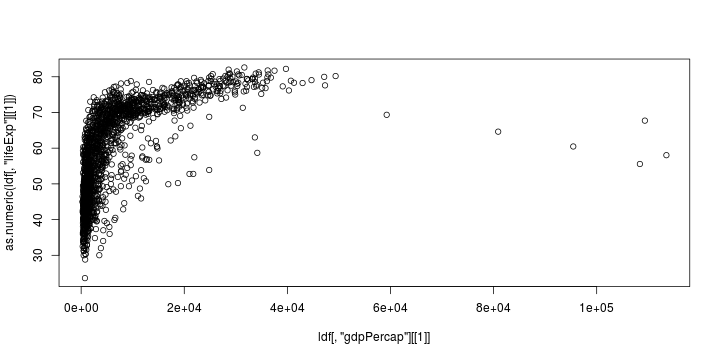setkey(dt, country) dt[c("France","Italy","Spain"),max(lifeExp),by="country"]  ## country V1 ## 1: France 80.657 ## 2: Italy 80.546 ## 3: Spain 80.941  dt[c("France","Italy","Spain"),max(lifeExp),by="country"]  ## country V1 ## 1: France 80.657 ## 2: Italy 80.546 ## 3: Spain 80.941  head(ldf[with(ldf,order(-lifeExp)),])  ## # A tibble: 6 × 6 ## country year pop continent lifeExp gdpPercap ## <fctr> <int> <dbl> <fctr> <dbl> <dbl> ## 1 Japan 2007 127467972 Asia 82.603 31656 ## 2 Hong Kong, China 2007 6980412 Asia 82.208 39725 ## 3 Japan 2002 127065841 Asia 82.000 28605 ## 4 Iceland 2007 301931 Europe 81.757 36181 ## 5 Switzerland 2007 7554661 Europe 81.701 37506 ## 6 Hong Kong, China 2002 6762476 Asia 81.495 30209  head(dt[order(-lifeExp)])  ## country year pop continent lifeExp gdpPercap ## 1: Japan 2007 127467972 Asia 82.603 31656 ## 2: Hong Kong, China 2007 6980412 Asia 82.208 39725 ## 3: Japan 2002 127065841 Asia 82.000 28605 ## 4: Iceland 2007 301931 Europe 81.757 36181 ## 5: Switzerland 2007 7554661 Europe 81.701 37506 ## 6: Hong Kong, China 2002 6762476 Asia 81.495 30209  head(setorder(dt,-lifeExp))  ## country year pop continent lifeExp gdpPercap ## 1: Japan 2007 127467972 Asia 82.603 31656 ## 2: Hong Kong, China 2007 6980412 Asia 82.208 39725 ## 3: Japan 2002 127065841 Asia 82.000 28605 ## 4: Iceland 2007 301931 Europe 81.757 36181 ## 5: Switzerland 2007 7554661 Europe 81.701 37506 ## 6: Hong Kong, China 2002 6762476 Asia 81.495 30209  arrange(ldf,-lifeExp)  ## # A tibble: 1,704 × 6 ## country year pop continent lifeExp gdpPercap ## <fctr> <int> <dbl> <fctr> <dbl> <dbl> ## 1 Japan 2007 127467972 Asia 82.603 31656 ## 2 Hong Kong, China 2007 6980412 Asia 82.208 39725 ## 3 Japan 2002 127065841 Asia 82.000 28605 ## 4 Iceland 2007 301931 Europe 81.757 36181 ## 5 Switzerland 2007 7554661 Europe 81.701 37506 ## 6 Hong Kong, China 2002 6762476 Asia 81.495 30209 ## 7 Australia 2007 20434176 Oceania 81.235 34435 ## 8 Spain 2007 40448191 Europe 80.941 28821 ## 9 Sweden 2007 9031088 Europe 80.884 33860 ## 10 Israel 2007 6426679 Asia 80.745 25523 ## # ... with 1,694 more rows  ldf$gdp=ldf$pop*ldf$gdpPercap
ldf = within(ldf,gdp = pop*gdpPercap)

## Error in eval(expr, envir, enclos): argument manquant, sans valeur associée par défaut

ldf = within(ldf,gdp <- pop*gdpPercap)
dt[,gdp:=pop*gdpPercap]

##             country year       pop continent lifeExp gdpPercap        gdp
## 1:            Japan 2007 127467972      Asia  82.603     31656 4.0351e+12
## 2: Hong Kong, China 2007   6980412      Asia  82.208     39725 2.7730e+11
## 3:            Japan 2002 127065841      Asia  82.000     28605 3.6347e+12
## 4:          Iceland 2007    301931    Europe  81.757     36181 1.0924e+10
## 5:      Switzerland 2007   7554661    Europe  81.701     37506 2.8335e+11
## 6: Hong Kong, China 2002   6762476      Asia  81.495     30209 2.0429e+11

mutate(ldf,gdp=pop*gdpPercap)

## # A tibble: 1,704 × 7
##        country  year      pop continent lifeExp gdpPercap        gdp
##         <fctr> <int>    <dbl>    <fctr>   <dbl>     <dbl>      <dbl>
## 1  Afghanistan  1952  8425333      Asia  28.801    779.45 6.5671e+09
## 2  Afghanistan  1957  9240934      Asia  30.332    820.85 7.5854e+09
## 3  Afghanistan  1962 10267083      Asia  31.997    853.10 8.7589e+09
## 4  Afghanistan  1967 11537966      Asia  34.020    836.20 9.6480e+09
## 5  Afghanistan  1972 13079460      Asia  36.088    739.98 9.6786e+09
## 6  Afghanistan  1977 14880372      Asia  38.438    786.11 1.1698e+10
## 7  Afghanistan  1982 12881816      Asia  39.854    978.01 1.2599e+10
## 8  Afghanistan  1987 13867957      Asia  40.822    852.40 1.1821e+10
## 9  Afghanistan  1992 16317921      Asia  41.674    649.34 1.0596e+10
## 10 Afghanistan  1997 22227415      Asia  41.763    635.34 1.4122e+10
## # ... with 1,694 more rows

countries=read.csv2("http://freakonometrics.free.fr/countries.csv",header=TRUE)
countries = countries[-1,]

##       Country Interational.Direct.Dial..IDD..Code ISO.3166 Car.Code
## 2 Afghanistan                                  93       AF
## 3     Albania                                 355       AL
## 4     Algeria                                 213       DZ
## 5     Andorra                              33 628       AD
## 6      Angola                                 244       AO
## 7    Anguilla                               1 809       AI
##         Capital              Currency ISO.Currency Digraph  ICAO   Language
## 2         Kabul               Afghani          AFA      AF   YA-     Pashto
## 3        Tirana                   Lek          ALL      AL   ZA-   Albanian
## 4       Algiers                 Dinar          DZD      DZ   7T-     Arabic
## 5 And. La Vella          French Franc          FRF      AD   C3-    Catalan
## 6        Luanda           New Irwanza          AON      AO   D2- Portuguese
## 7               East Caribbean Dollar          XCD      AI VP-LA
##   ISO.639
## 2      PS
## 3      SQ
## 4      AR
## 5      CA
## 6      PT
## 7

df2 = countries[,c("Country","Capital")]
names(df2)=c("countries","capital")

df1 = aggregate(ldf$lifeExp,FUN=max,by=list(ldf$country))
names(df1)=c("countries","lifeExp")
dim(df1)

##  142   2

dim(df2)

##  263   2

tail(merge(df1,df2))

##          countries lifeExp    capital
## 122 United Kingdom  79.425     London
## 123        Uruguay  76.384 Montevideo
## 124      Venezuela  73.747    Caracas
## 125        Vietnam  74.249      Hanoi
## 126         Zambia  51.821     Lusaka
## 127       Zimbabwe  62.351     Harare

tail(merge(df1,df2,all.x=TRUE))

##              countries lifeExp capital
## 137          Venezuela  73.747 Caracas
## 138            Vietnam  74.249   Hanoi
## 139 West Bank and Gaza  73.422    <NA>
## 140        Yemen, Rep.  62.698    <NA>
## 141             Zambia  51.821  Lusaka
## 142           Zimbabwe  62.351  Harare

tail(merge(df1,df2,all.y=TRUE))

##                     countries lifeExp  capital
## 258 Wallis and Futuna Islands      NA
## 259                 West Bank      NA
## 260                     World      NA
## 261                     Yemen      NA   Sana'a
## 263                     Zaire      NA Kinshasa

ldf1 = group_by(ldf, country) %>% summarize(max(lifeExp))
names(ldf1)=c("countries","lifeExp")

## Error in names(ldf1) = c("countries", "lifeExp"): attribut 'names'  doit être de même longueur que le vecteur 

ldf2 <- tbl_df(df2)
inner_join(ldf1,ldf2)

## Error: No common variables. Please specify by param.

left_join(ldf1,ldf2)

## Error: No common variables. Please specify by param.

ldf1 %>% inner_join(ldf2)

## Error: No common variables. Please specify by param.

dt1 = dt[,max(lifeExp),by="country"]
setnames(dt1,c("countries","lifeExp"))
dt2 <- data.table(df2)
setkey(dt1,"countries")
setkey(dt2,"countries")
dt1[dt2,nomatch=0]

##        countries lifeExp      capital
##   1: Afghanistan  43.828        Kabul
##   2:     Albania  76.423       Tirana
##   3:     Algeria  72.301      Algiers
##   4:      Angola  42.731       Luanda
##   5:   Argentina  75.320 Buenos Aires
##  ---
## 123:     Uruguay  76.384   Montevideo
## 124:   Venezuela  73.747      Caracas
## 125:     Vietnam  74.249        Hanoi
## 126:      Zambia  51.821       Lusaka
## 127:    Zimbabwe  62.351       Harare

dt1[dt2]

##        countries lifeExp       capital
##   1: Afghanistan  43.828         Kabul
##   2:     Albania  76.423        Tirana
##   3:     Algeria  72.301       Algiers
##   4:     Andorra      NA And. La Vella
##   5:      Angola  42.731        Luanda
##  ---
## 259:       Yemen      NA        Sana'a
## 261:       Zaire      NA      Kinshasa
## 262:      Zambia  51.821        Lusaka
## 263:    Zimbabwe  62.351        Harare

dt2[dt1]

##               countries      capital lifeExp
##   1:        Afghanistan        Kabul  43.828
##   2:            Albania       Tirana  76.423
##   3:            Algeria      Algiers  72.301
##   4:             Angola       Luanda  42.731
##   5:          Argentina Buenos Aires  75.320
##  ---
## 138:            Vietnam        Hanoi  74.249
## 139: West Bank and Gaza           NA  73.422
## 140:        Yemen, Rep.           NA  62.698
## 141:             Zambia       Lusaka  51.821
## 142:           Zimbabwe       Harare  62.351

library(stringr,verbose=FALSE,quietly=TRUE,warn.conflicts=FALSE)
library(lubridate,verbose=FALSE,quietly=TRUE,warn.conflicts=FALSE)
library(data.table,verbose=FALSE,quietly=TRUE,warn.conflicts=FALSE)
library(LaF,verbose=FALSE,quietly=TRUE,warn.conflicts=FALSE)
loc="http://freakonometrics.free.fr/gapminderDataFiveYear.txt"
ssn <- laf_open_csv("temp",sep="\t",
column_types=c("character","integer","numeric","character","numeric","numeric"),
column_names=names(ldf)[1:6])
object.size(ssn)

## 2888 bytes


LaF (large ASCII files)

str(ssn)

## Formal class 'laf' [package "LaF"] with 8 slots
##   ..@ file_id      : int 0
##   ..@ filename     : chr "temp"
##   ..@ file_type    : chr "csv"
##   ..@ column_names : chr [1:6] "country" "year" "pop" "continent" ...
##   ..@ column_types : int [1:6] 3 1 0 3 0 0
##   ..@ column_widths: int(0)
##   ..@ levels       : list()
##   ..@ options      :List of 4
##   .. ..$sep : chr "\t" ## .. ..$ dec : chr "."
##   .. ..$skip: int 0 ## .. ..$ trim: logi FALSE

nrow(ssn)

##  1705

go_through <- which(ssn[1:(nrow(ssn)-1),'country'] != ssn[2:nrow(ssn),'country'])+1
if(go_through[length(go_through)] != nrow(ssn)) go_through <- c(go_through, nrow(ssn))
go_through <- cbind(go_through[-length(go_through)], c(go_through[-c(1, length(go_through))]-1, go_through[length(go_through)]))

worst_life_exp <- function(s){
data <- ssn[go_through[s,1]:go_through[s,2],c("country","year","lifeExp")]
data[which.min(data$lifeExp),] } worst_life_exp(10)  ## country year lifeExp ## 1 Belgium 1952 68  s <- 10 ldf[go_through[s,1]:go_through[s,2]-1,]  ## # A tibble: 12 × 7 ## country year pop continent lifeExp gdpPercap gdp ## <fctr> <int> <dbl> <fctr> <dbl> <dbl> <dbl> ## 1 Belgium 1952 8730405 Europe 68.000 8343.1 7.2839e+10 ## 2 Belgium 1957 8989111 Europe 69.240 9715.0 8.7329e+10 ## 3 Belgium 1962 9218400 Europe 70.250 10991.2 1.0132e+11 ## 4 Belgium 1967 9556500 Europe 70.940 13149.0 1.2566e+11 ## 5 Belgium 1972 9709100 Europe 71.440 16672.1 1.6187e+11 ## 6 Belgium 1977 9821800 Europe 72.800 19118.0 1.8777e+11 ## 7 Belgium 1982 9856303 Europe 73.930 20979.8 2.0678e+11 ## 8 Belgium 1987 9870200 Europe 75.350 22525.6 2.2233e+11 ## 9 Belgium 1992 10045622 Europe 76.460 25575.6 2.5692e+11 ## 10 Belgium 1997 10199787 Europe 77.530 27561.2 2.8112e+11 ## 11 Belgium 2002 10311970 Europe 78.320 30485.9 3.1437e+11 ## 12 Belgium 2007 10392226 Europe 79.441 33692.6 3.5014e+11  # Graphs with ggplot2 library(gamair,verbose=FALSE,quietly=TRUE,warn.conflicts=FALSE) data(chicago) head(chicago)  ## death pm10median pm25median o3median so2median time tmpd ## 1 130 -7.43354 NA -19.592 1.92804 -2556.5 31.5 ## 2 150 NA NA -19.039 -0.98556 -2555.5 33.0 ## 3 101 -0.82653 NA -20.217 -1.89142 -2554.5 33.0 ## 4 135 5.56646 NA -19.676 6.13934 -2553.5 29.0 ## 5 126 NA NA -19.217 2.27846 -2552.5 32.0 ## 6 130 6.56646 NA -17.634 9.85858 -2551.5 40.0  ggplot2 documentation base=data.frame(death=chicago$death,
temp_F=chicago$tmpd, o3=chicago$o3median,
date=seq(as.Date("1987-01-01"),
as.Date("2000-12-31"),by=1))
base$temp_C <- (base$temp_F-32)/1.8
base$year <- substring(base$date,1,4)

date2season <- function(date){
m <- as.numeric(format(as.Date(date, format = "%d/%m/%Y"), "%m"))
d <- as.numeric(format(as.Date(date, format = "%d/%m/%Y"), "%d"))
s <- NA
if(m %in% c(1,2) | ((m==12)&(d>=21)) |  ((m==3)&(d<21))) s <- "winter"
if(m %in% c(4,5) | ((m==3)&(d>=21)) |  ((m==6)&(d<21))) s <- "spring"
if(m %in% c(7,8) | ((m==6)&(d>=21)) |  ((m==9)&(d<21))) s <- "summer"
if(m %in% c(10,11) | ((m==9)&(d>=21)) |  ((m==12)&(d<21))) s <- "autumn"
return(s)}
base$season <- sapply(base$date,date2season)

##   death temp_F      o3       date   temp_C year season
## 1   130   31.5 -19.592 1987-01-01 -0.27778 1987 winter
## 2   150   33.0 -19.039 1987-01-02  0.55556 1987 winter
## 3   101   33.0 -20.217 1987-01-03  0.55556 1987 winter
## 4   135   29.0 -19.676 1987-01-04 -1.66667 1987 winter
## 5   126   32.0 -19.217 1987-01-05  0.00000 1987 winter
## 6   130   40.0 -17.634 1987-01-06  4.44444 1987 winter

plot(base[,c("date", "temp_C")])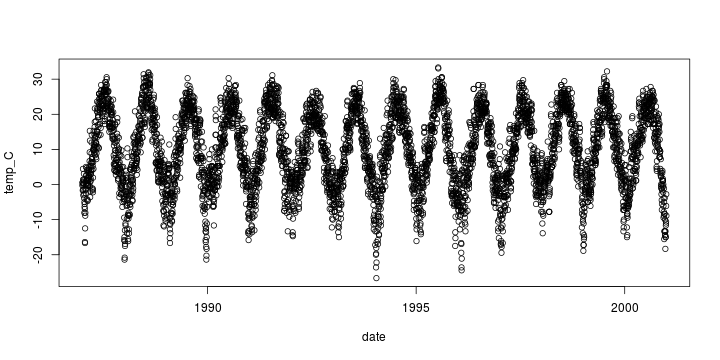library(ggplot2,verbose=FALSE,quietly=TRUE,warn.conflicts=FALSE)
g <- ggplot(base, aes(date, temp_C)) +
geom_point()
g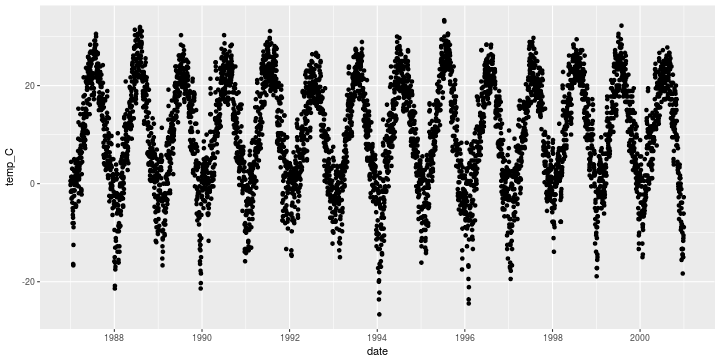ggsave(file="figure.pdf", scale=3)

## Saving 30 x 15 in image

g <- g+labs(title='Temperature')
g <- g+ggtitle('Temperature')
gg+theme(plot.title = element_text(size=20, face="bold", vjust=2, color="red"))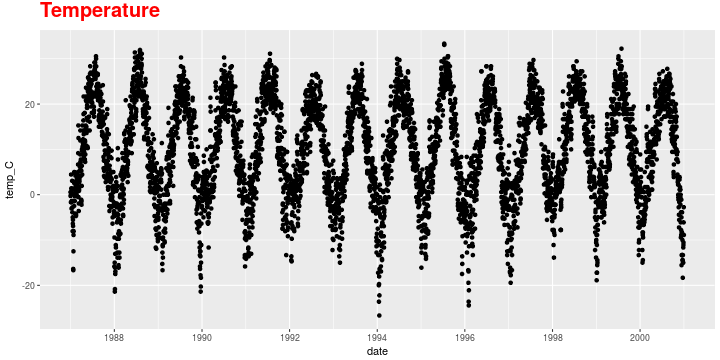g<-g+labs(
x="Date",
y=expression(paste("Temperature ( ", degree ~ C, " )")),
title="Temperature in Chicago")
g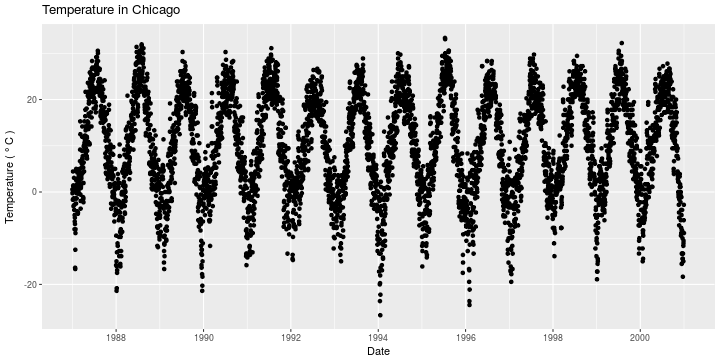g+ theme(
axis.title.x = element_text(color="blue"),
axis.title.y = element_text(color="blue")
)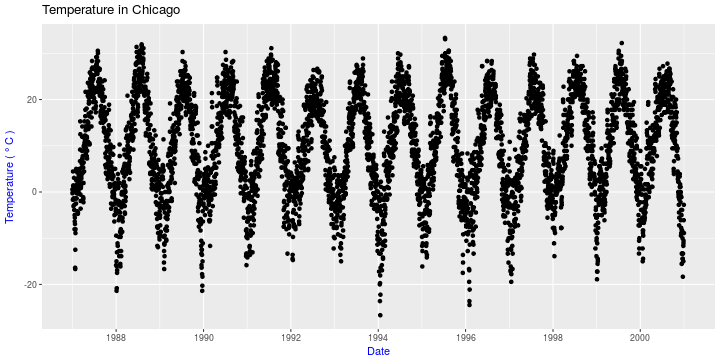g + ylim(c(-20,60))

## Warning: Removed 10 rows containing missing values (geom_point).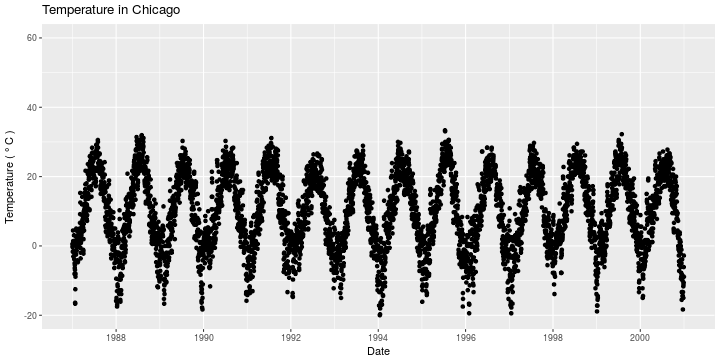g <- g + xlim(as.Date(c("1995-01-01","1999-01-01")))
g

## Warning: Removed 3652 rows containing missing values (geom_point).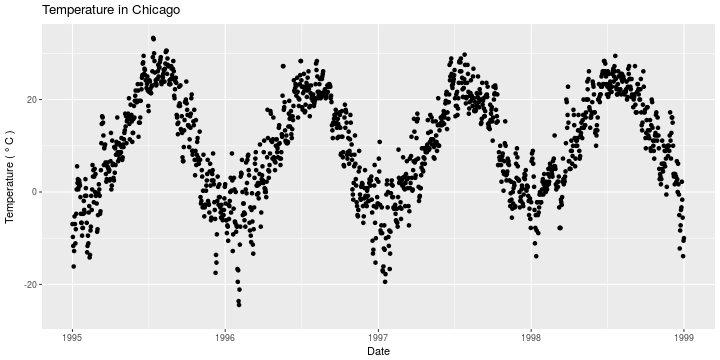base2=data.frame(
today=base$temp_C[1:5113],tomorrow=base$temp_C[2:5114])
ggplot(base2, aes(today, tomorrow))+geom_point()+ coord_equal()g <- ggplot(base, aes(date, temp_C, color=factor(season)))+
xlim(as.Date(c("1995-01-01","1999-01-01")))+
geom_point()
g+theme(legend.title=element_blank())+
guides(colour = guide_legend(override.aes = list(size=4)))

## Warning: Removed 3652 rows containing missing values (geom_point).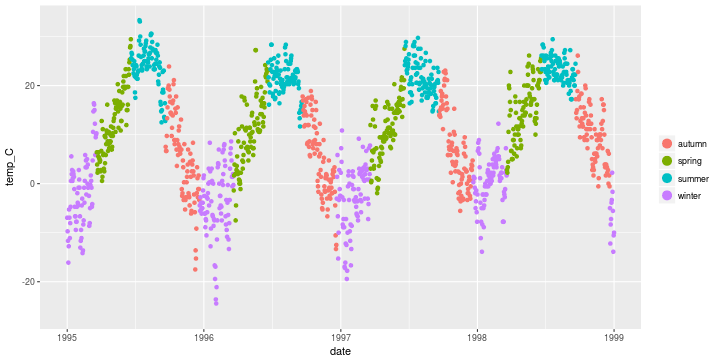g<-ggplot(base, aes(date, temp_C))+
xlim(as.Date(c("1995-01-01","1999-01-01")))
g+geom_line(color="grey")

## Warning: Removed 3652 rows containing missing values (geom_path).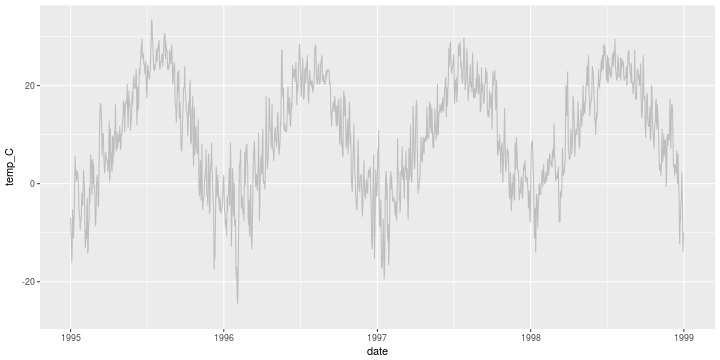g+geom_line(aes(color="temperature"))+
scale_colour_manual(name='', values=c('temperature'='grey'))

## Warning: Removed 3652 rows containing missing values (geom_path).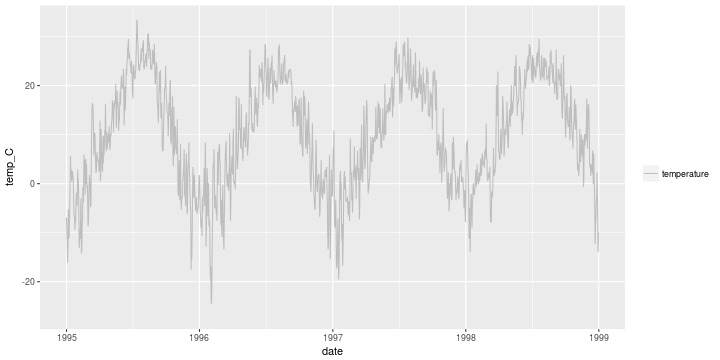ggplot(base, aes(date, temp_C, color=factor(season)))+
geom_point() +
scale_color_manual(values=
c("dodgerblue4", "darkolivegreen4",
"darkorchid3", "goldenrod1"))ggplot(base, aes(date, temp_C, color=factor(season)))+
geom_point() +
scale_color_brewer(palette="Set1")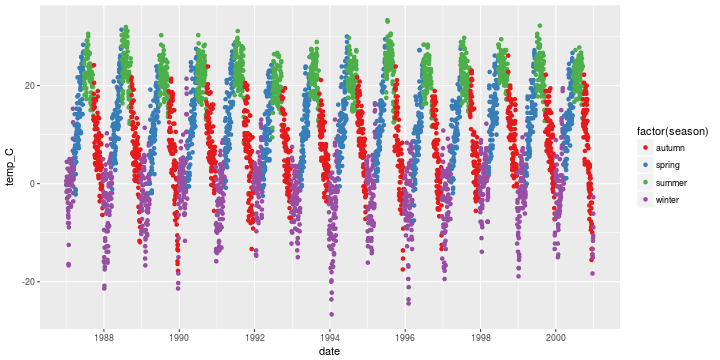ggplot(base, aes(date, temp_C, color=temp_C))+geom_point()+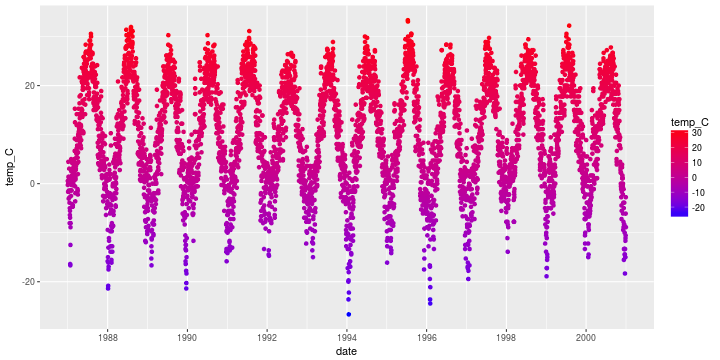ggplot(base, aes(date, temp_C, color=temp_C))+geom_point()+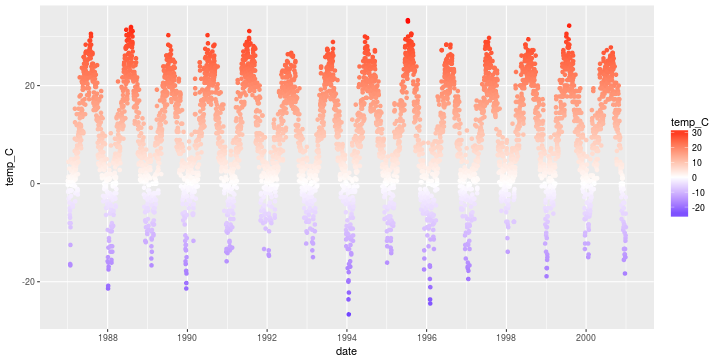ggplot(base, aes(date, temp_C))+geom_point(color="firebrick")+
theme(panel.background = element_rect(fill = 'light yellow'))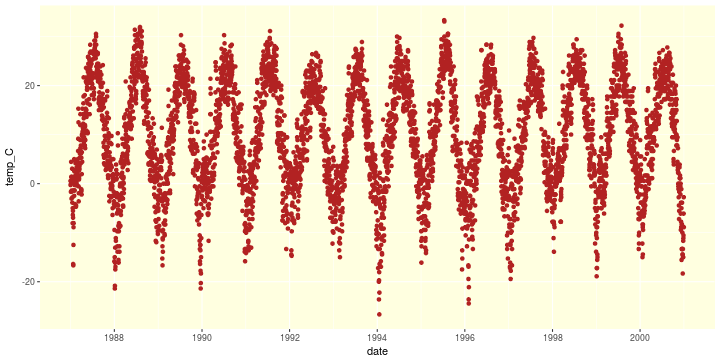ggplot(base, aes(date,temp_C))+geom_point(color="firebrick")+
facet_wrap(~year, ncol=2, scales="free")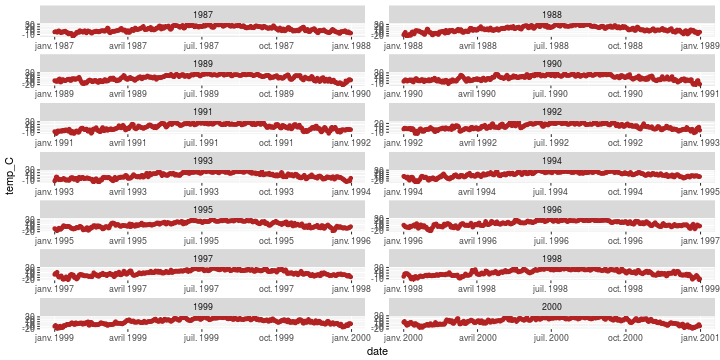library(ggthemes)
ggplot(base, aes(date, temp_C, color=factor(season)))+
geom_point()+labs(x="Date", y=expression(paste("Temperature ( ", degree ~ C, " )")), title="This plot looks like MS Excel")+
theme_excel()+scale_colour_excel()+
theme(legend.title=element_blank())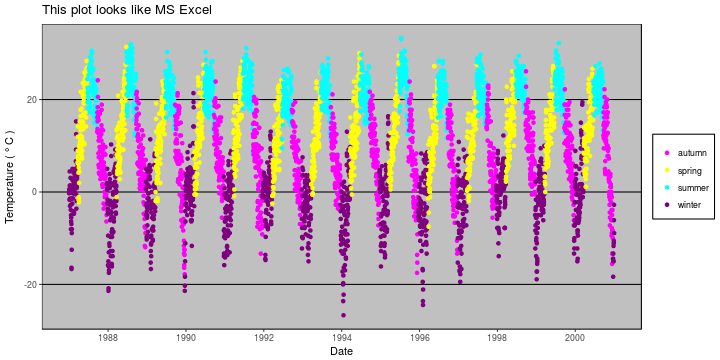library(ggthemes)
ggplot(base, aes(date, temp_C, color=factor(season)))+
geom_point()+labs(x="Date", y=expression(paste("Temperature ( ", degree ~ C, " )")), title="This plot looks like 'The Economist'")+
theme_economist()+theme(legend.title=element_blank())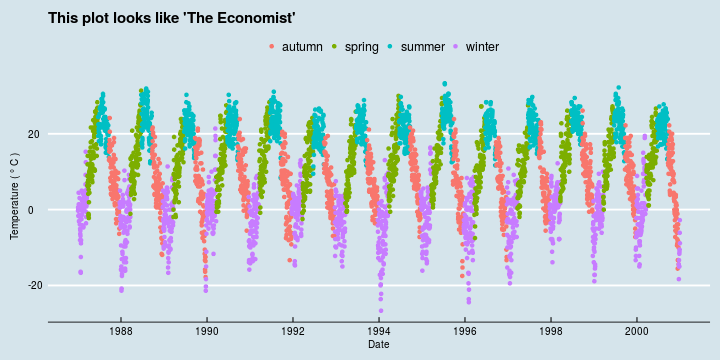# OpenStreetMap

library(OpenStreetMap,verbose=FALSE,quietly=TRUE,warn.conflicts=FALSE)
map <- openmap(c(lat= 51.516, lon= -.141), c(lat= 51.511, lon= -.133))
map <- openproj(map, projection = "+init=epsg:27700")
plot(map)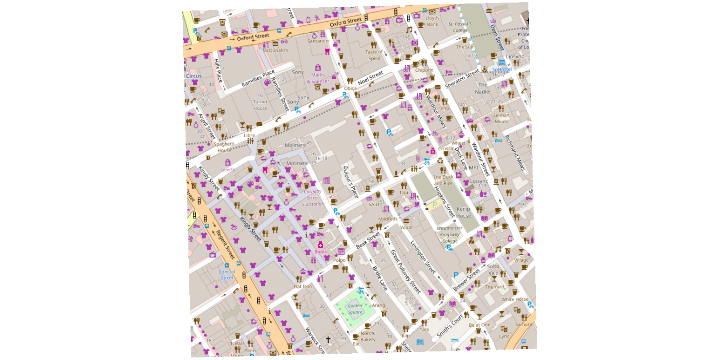loc="http://freakonometrics.free.fr/cholera.zip"
unzip("cholera.zip", exdir="./")
library(maptools,verbose=FALSE,quietly=TRUE,warn.conflicts=FALSE)

## Checking rgeos availability: TRUE

deaths<-readShapePoints("Cholera_Deaths")

##   coords.x1 coords.x2
## 0    529309    181031
## 1    529312    181025
## 2    529314    181020
## 3    529317    181014
## 4    529321    181008
## 5    529337    181006


documentation on shapefiles

plot(map)
points(deaths@coords,col="red", pch=19, cex=.7 )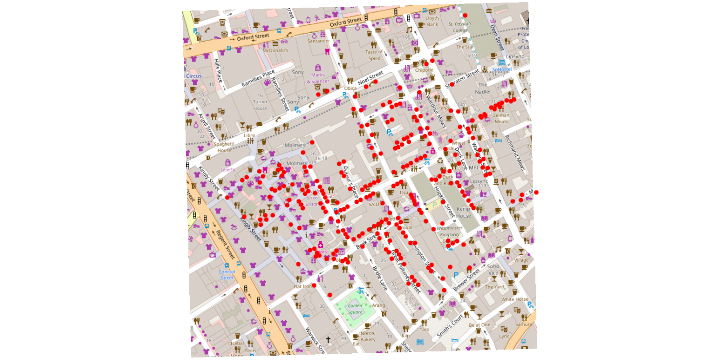X <- deaths@coords
library(KernSmooth)

## KernSmooth 2.23 loaded
## Copyright M. P. Wand 1997-2009

kde2d <- bkde2D(X, bandwidth=c(bw.ucv(X[,1]),bw.ucv(X[,2])))

## Warning in bw.ucv(X[, 1]): minimum occurred at one end of the range

library(grDevices,verbose=FALSE,quietly=TRUE,warn.conflicts=FALSE)
clrs <- colorRampPalette(c(rgb(0,0,1,0), rgb(0,0,1,1)), alpha = TRUE)(20)

plot(map)
points(deaths@coords,col="red", pch=19, cex=.7 )
image(x=kde2d$x1, y=kde2d$x2,z=kde2d$fhat, add=TRUE,col=clrs) contour(x=kde2d$x1, y=kde2d$x2,z=kde2d$fhat, add=TRUE)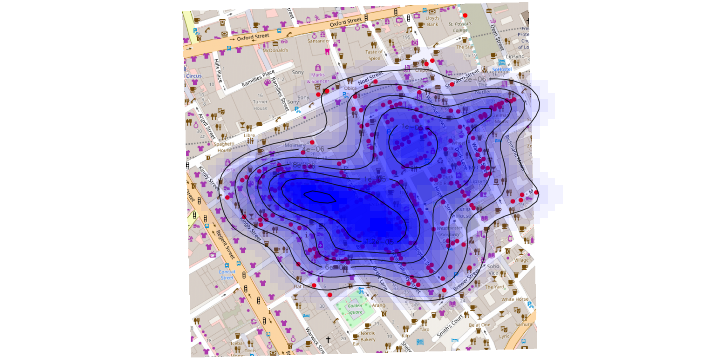library(ggmap,verbose=FALSE,quietly=TRUE,warn.conflicts=FALSE)
get_london <- get_map(c(-.137,51.513), zoom=17)

## Map from URL : http://maps.googleapis.com/maps/api/staticmap?center=51.513,-0.137&zoom=17&size=640x640&scale=2&maptype=terrain&language=en-EN&sensor=false

london <- ggmap(get_london)


# OpenStreetMap

london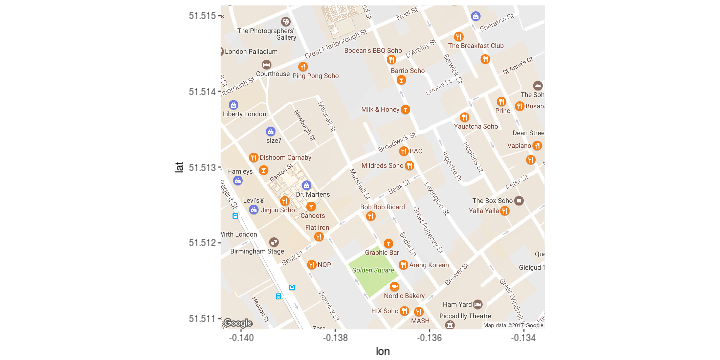df_deaths <- data.frame(X)
library(sp,verbose=FALSE,quietly=TRUE,warn.conflicts=FALSE)
library(rgdal,verbose=FALSE,quietly=TRUE,warn.conflicts=FALSE)

## rgdal: version: 1.2-4, (SVN revision 643)
##  Geospatial Data Abstraction Library extensions to R successfully loaded
##  Loaded GDAL runtime: GDAL 1.11.3, released 2015/09/16
##  Path to GDAL shared files: /usr/share/gdal/1.11
##  Loaded PROJ.4 runtime: Rel. 4.9.2, 08 September 2015, [PJ_VERSION: 492]
##  Path to PROJ.4 shared files: (autodetected)
##  Linking to sp version: 1.2-3

coordinates(df_deaths)=~coords.x1+coords.x2
proj4string(df_deaths)=CRS("+init=epsg:27700")
df_deaths = spTransform(df_deaths,CRS("+proj=longlat +datum=WGS84"))


# OpenStreetMap

london + geom_point(aes(x=coords.x1,y=coords.x2),data=data.frame(df_deaths@coords),col="red")

## Warning: Removed 9 rows containing missing values (geom_point).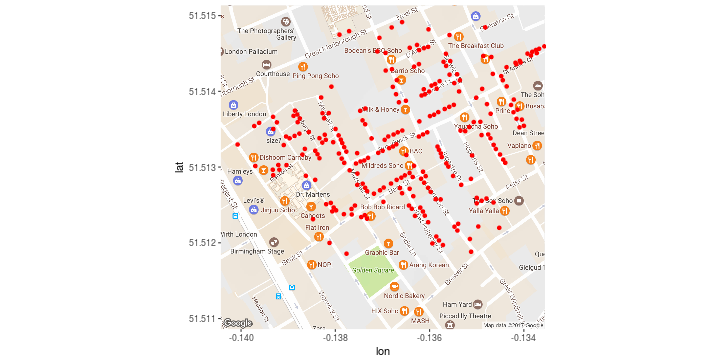london <- london + geom_point(aes(x=coords.x1, y=coords.x2),
data=data.frame(df_deaths@coords),col="red") +
geom_density2d(data = data.frame(df_deaths@coords),
aes(x = coords.x1, y=coords.x2), size = 0.3) +
stat_density2d(data = data.frame(df_deaths@coords),
aes(x = coords.x1, y=coords.x2,fill = ..level.., alpha = ..level..),
size = 0.01, bins = 16, geom = "polygon") + scale_fill_gradient(low = "green", high = "red",
guide = FALSE) + scale_alpha(range = c(0, 0.3), guide = FALSE)

london

## Warning: Removed 9 rows containing non-finite values (stat_density2d).

## Warning: Removed 9 rows containing non-finite values (stat_density2d).

## Warning: Removed 9 rows containing missing values (geom_point).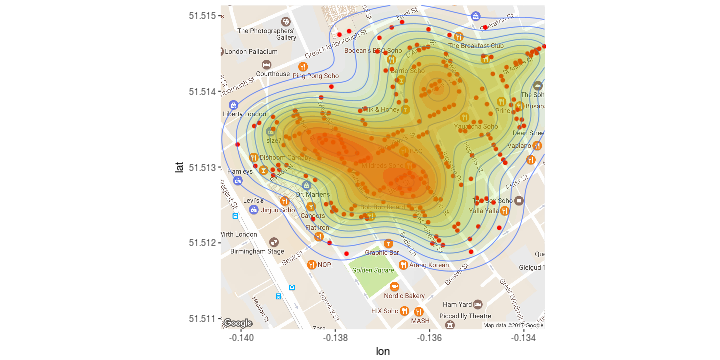require(leaflet)
df_deaths <- data.frame(deaths@coords)
coordinates(df_deaths)=~coords.x1+coords.x2
proj4string(df_deaths)=CRS("+init=epsg:27700")
df_deaths=spTransform(df_deaths,CRS("+proj=longlat +datum=WGS84"))
df=data.frame(df_deaths@coords)
lng=df$coords.x1 lat=df$coords.x2

m = m %>% fitBounds(-.141,  51.511, -.133, 51.516)

rd=.5; op=.8; clr="blue"

X=cbind(lng,lat)
kde2d <- bkde2D(X, bandwidth=c(bw.ucv(X[,1]),bw.ucv(X[,2])))

## Warning in bw.ucv(X[, 1]): minimum occurred at one end of the range

x=kde2d$x1; y=kde2d$x2; z=kde2d$fhat CL=contourLines(x , y , z) m = leaflet() %>% addTiles()  m = m %>% addPolygons(CL[]$x,CL[]$y,fillColor = "red", stroke = FALSE)  m = leaflet() %>% addTiles() m %>% addCircles(lng,lat, radius = rd,opacity=op,col=clr) %>% addPolygons(CL[]$x,CL[]$y,fillColor = "red", stroke = FALSE)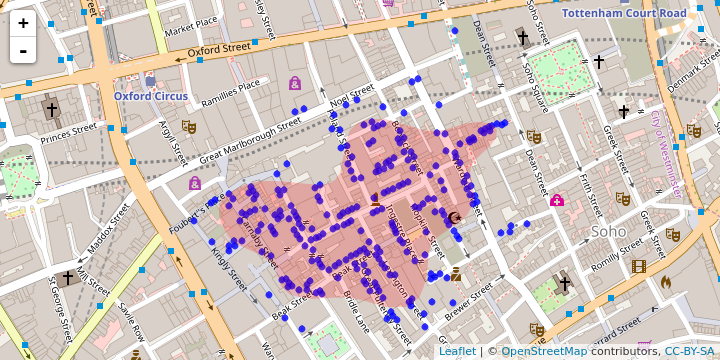m = leaflet() %>% addTiles() m = m %>% addCircles(lng,lat, radius = rd,opacity=op,col=clr) %>% addPolygons(CL[]$x,CL[]$y,fillColor = "red", stroke = FALSE) %>% addPolygons(CL[]$x,CL[]$y,fillColor = "red", stroke = FALSE) %>% addPolygons(CL[]$x,CL[]$y,fillColor = "red", stroke = FALSE) %>% addPolygons(CL[]$x,CL[]$y,fillColor = "red", stroke = FALSE) %>% addPolygons(CL[]$x,CL[]$y,fillColor = "red", stroke = FALSE)  m = leaflet() %>% addTiles() m = m %>% addCircles(lng,lat, radius = rd,opacity=op,col=clr) %>% addPolylines(CL[]$x,CL[]$y,color = "red") %>% addPolylines(CL[]$x,CL[]$y,color = "red") %>% addPolylines(CL[]$x,CL[]$y,color = "red")  library(osmar) s=osmsource_api() city=c((51.511+51.516)/2, -(.133+.141)/2) bb=center_bbox(city,city, 800, 900) ua=get_osm(bb, source = s) bg_ids=find(ua, way(tags(k=="building"))) bg_ids=find_down(ua, way(bg_ids)) bg=subset(ua, ids = bg_ids) bg_poly=as_sp(bg, "polygons")  plot(bg_poly, col = gray.colors(12),border="gray")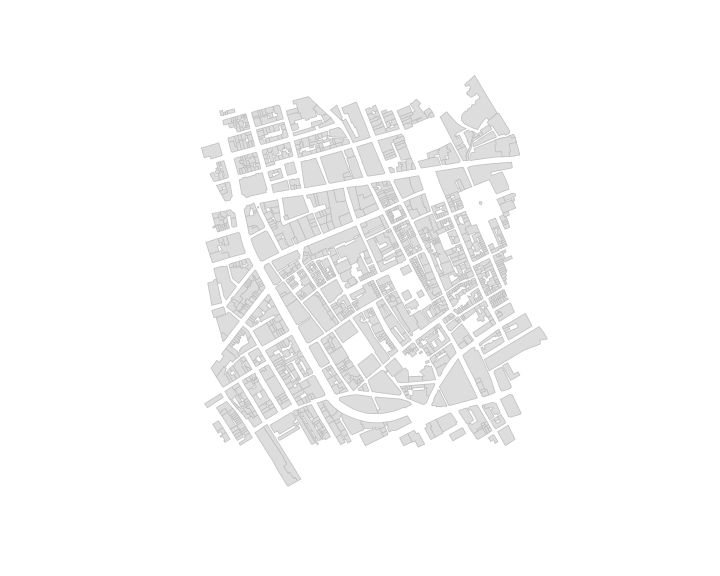cw_ids=find(ua, way(tags(v %in% c("residential","pedestrian")))) cw_ids=find_down(ua, way(cw_ids)) cw=subset(ua, ids = cw_ids) cw_line=as_sp(cw, "lines")  plot(bg_poly, col = gray.colors(12),border="gray") plot(cw_line, add = TRUE, col = gray.colors(12),lwd=3)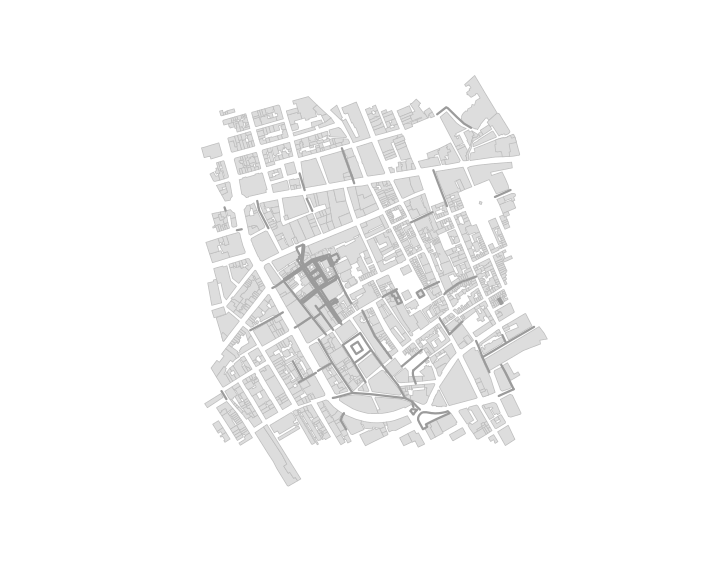# Map with googleVis library(googleVis) df=data.frame(state=c("AU-WA","AU-VIC","AU-NT", "AU-NSW", "AU-SA","AU-QLD","AU-TAS","NZ"), R_users=c(323,425,154,486,201,195,87,123)) Geo <- gvisGeoChart(df, locationvar="state",colorvar=c("R_users"), options=list(region="AU", dataMode="regions",resolution="provinces", width="80%", height="80%"))  # Map with googleVis library(XML,verbose=FALSE,quietly=TRUE,warn.conflicts=FALSE) year <- 2012 loc <- paste("http://weather.unisys.com/hurricane/atlantic/",year,"/index.php",sep="") tabs <- readHTMLTable(htmlParse(loc)) tabs  ##$NULL
##     #                    Name          Date Wind Pres Cat
## 1   1  Tropical Storm ALBERTO     19-23 MAY   50  995   -
## 2   2    Tropical Storm BERYL 25 MAY- 2 JUN   60  992   -
## 3   3       Hurricane-1 CHRIS     17-24 JUN   75  974   1
## 4   4    Tropical Storm DEBBY     23-27 JUN   55  990   -
## 5   5     Hurricane-2 ERNESTO      1-10 AUG   85  973   2
## 6   6 Tropical Storm FLORENCE      3- 8 AUG   50 1002   -
## 7   7   Tropical Storm HELENE      9-19 AUG   40 1004   -
## 8   8      Hurricane-2 GORDON     15-21 AUG   95  965   2
## 9   9       Hurricane-1 ISAAC 20 AUG- 1 SEP   70  965   1
## 10 10    Tropical Storm JOYCE     21-24 AUG   35 1006   -
## 11 11        Hurricane-2 KIRK 28 AUG- 3 SEP   90  970   2
## 12 12      Hurricane-1 LESLIE 28 AUG-12 SEP   70  968   1
## 13 13     Hurricane-3 MICHAEL      2-12 SEP  100  964   3
## 14 14      Hurricane-1 NADINE 10 SEP- 4 OCT   80  978   1
## 15 15    Tropical Storm OSCAR      2- 5 OCT   45  994   -
## 16 16    Tropical Storm PATTY     10-13 OCT   40 1005   -
## 17 17      Hurricane-1 RAFAEL     12-26 OCT   80  966   1
## 18 18       Hurricane-3 SANDY     21-31 OCT  100  940   3
## 19 19     Tropical Storm TONY     21-26 OCT   45 1000   -

storms <- unlist(strsplit(as.character(tabs[]$Name),split=" ")) storms  ##  "Tropical" "Storm" "ALBERTO" "Tropical" "Storm" ##  "BERYL" "Hurricane-1" "CHRIS" "Tropical" "Storm" ##  "DEBBY" "Hurricane-2" "ERNESTO" "Tropical" "Storm" ##  "FLORENCE" "Tropical" "Storm" "HELENE" "Hurricane-2" ##  "GORDON" "Hurricane-1" "ISAAC" "Tropical" "Storm" ##  "JOYCE" "Hurricane-2" "KIRK" "Hurricane-1" "LESLIE" ##  "Hurricane-3" "MICHAEL" "Hurricane-1" "NADINE" "Tropical" ##  "Storm" "OSCAR" "Tropical" "Storm" "PATTY" ##  "Hurricane-1" "RAFAEL" "Hurricane-3" "SANDY" "Tropical" ##  "Storm" "TONY"  index <- storms%in%c("Tropical", "Storm", paste("Hurricane-",1:6,sep=""), "Depression", "Subtropical", "Extratropical", "Low", paste("Storm-",1:6,sep=""), "Xxx") nstorms <- storms[!index] nstorms  ##  "ALBERTO" "BERYL" "CHRIS" "DEBBY" "ERNESTO" "FLORENCE" ##  "HELENE" "GORDON" "ISAAC" "JOYCE" "KIRK" "LESLIE" ##  "MICHAEL" "NADINE" "OSCAR" "PATTY" "RAFAEL" "SANDY" ##  "TONY"  TRACK=NULL  for(i in length(nstorms):1){ if((nstorms[i]=="SIXTEE")&(year==2008)) nstorms[i] <- "SIXTEEN" if((nstorms[i]=="LAUR")&(year==2008)) nstorms[i] <- "LAURA" if((nstorms[i]=="FIFTEE")&(year==2007)) nstorms[i] <- "FIFTEEN" if((nstorms[i]=="CHANTA")&(year==2007)) nstorms[i] <- "CHANTAL" if((nstorms[i]=="ANDR")&(year==2007)) nstorms[i] <- "ANDREA" if((nstorms[i]=="NINETE")&(year==2005)) nstorms[i] <- "NINETEEN" if((nstorms[i]=="JOSEPH")&(year==2002)) nstorms[i] <- "JOSEPHINE" if((nstorms[i]=="FLOY")&(year==1993)) nstorms[i] <- "FLOYD" if((nstorms[i]=="KEIT")&(year==1988)) nstorms[i] <- "KEITH" if((nstorms[i]=="CHARLI")&(year==1972)) nstorms[i] <- "CHARLIE"}  for(i in length(nstorms):1){ loc <- paste("http://weather.unisys.com/hurricane/atlantic/",year,"/",nstorms[i],"/track.dat",sep="") track <- read.fwf(loc,skip=3,widths = c(4,6,8,12,4,6,20)) names(track)=c("ADV","LAT","LON","TIME","WIND","PR","STAT") track$LAT=as.numeric(as.character(track$LAT)) track$LON=as.numeric(as.character(track$LON)) track$WIND=as.numeric(as.character(track$WIND)) track$PR=as.numeric(as.character(track$PR)) track$year=year
track$name=nstorms[i] TRACK=rbind(TRACK,track)} tail(TRACK,10)  ## ADV LAT LON TIME WIND PR STAT year name ## 645 11 30.4 -79.1 05/21/12Z 35 1007 TROPICAL STORM 2012 ALBERTO ## 646 12 30.5 -78.3 05/21/18Z 35 1006 TROPICAL STORM 2012 ALBERTO ## 647 13 30.7 -77.1 05/22/00Z 35 1007 TROPICAL STORM 2012 ALBERTO ## 648 14 31.5 -76.1 05/22/06Z 35 1007 TROPICAL STORM 2012 ALBERTO ## 649 15 32.5 -74.7 05/22/12Z 30 1008 LOW 2012 ALBERTO ## 650 16 33.4 -73.4 05/22/18Z 30 1008 LOW 2012 ALBERTO ## 651 17 34.1 -71.9 05/23/00Z 25 1010 LOW 2012 ALBERTO ## 652 18 34.9 -70.1 05/23/06Z 25 1011 LOW 2012 ALBERTO ## 653 19 35.5 -67.9 05/23/12Z 25 1012 LOW 2012 ALBERTO ## 654 20 35.9 -66.0 05/23/18Z 25 1012 LOW 2012 ALBERTO  extract.track <- function(year=2012,p=TRUE){ loc <- paste("http://weather.unisys.com/hurricane/atlantic/",year,"/index.php",sep="") tabs <- readHTMLTable(htmlParse(loc)) storms <- unlist(strsplit(as.character( tabs[]$Name),split=" "))
index <- storms%in%c("Tropical","Storm",paste("Hurricane-",1:6,sep=""),"Depression","Subtropical","Extratropical","Low",paste("Storm-",1:6,sep=""),
"Xxx")
nstorms  <- storms[!index]
TRACK=NULL
for(i in length(nstorms):1){
loc=paste("http://weather.unisys.com/hurricane/atlantic/",year,"/",nstorms[i],"/track.dat",sep="")
track$LAT=as.numeric(as.character(track$LAT))
track$LON=as.numeric(as.character(track$LON))
track$WIND=as.numeric(as.character(track$WIND))
track$PR=as.numeric(as.character(track$PR))
track$year=year track$name=nstorms[i]
TRACK=rbind(TRACK,track)
if(p==TRUE){  cat(year,i,nstorms[i],nrow(track),"\n")}}
return(TRACK)}

TOTTRACK=NULL
#  for(y in 2012:1851){
#    TOTTRACK=rbind(TOTTRACK,extract.track(y))
#  }

loc="http://freakonometrics.free.fr/TRACK-ATLANTIC.Rdata"
dim(TOTTRACK)

##  26712     9

library(grDevices,verbose=FALSE,quietly=TRUE,warn.conflicts=FALSE)
library(maps,verbose=FALSE,quietly=TRUE,warn.conflicts=FALSE)
map("world", col="light yellow", fill=TRUE)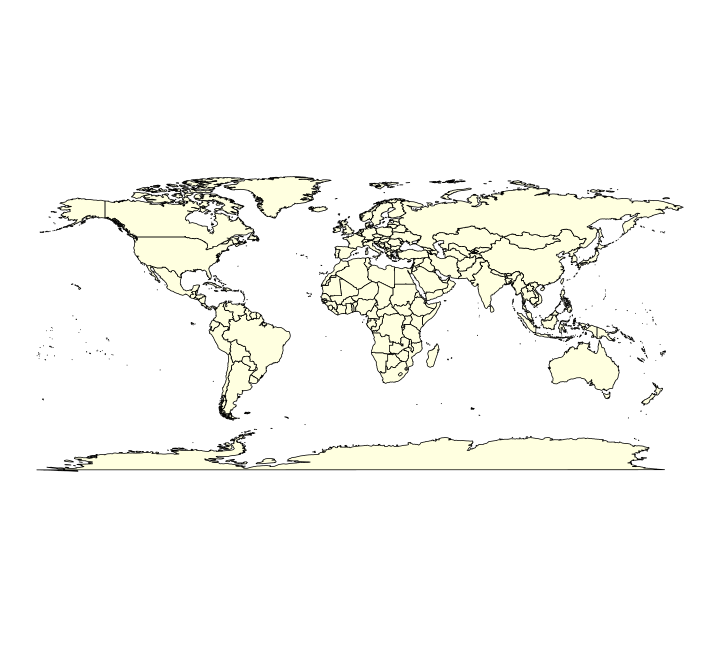map("world", col="light yellow", fill=TRUE)
for(n in unique(TOTTRACK$name)){ lines(TOTTRACK$LON[TOTTRACK$name==n],TOTTRACK$LAT[TOTTRACK$name==n],lwd=.5,col=rgb(1, 0, 0, alpha=.5))}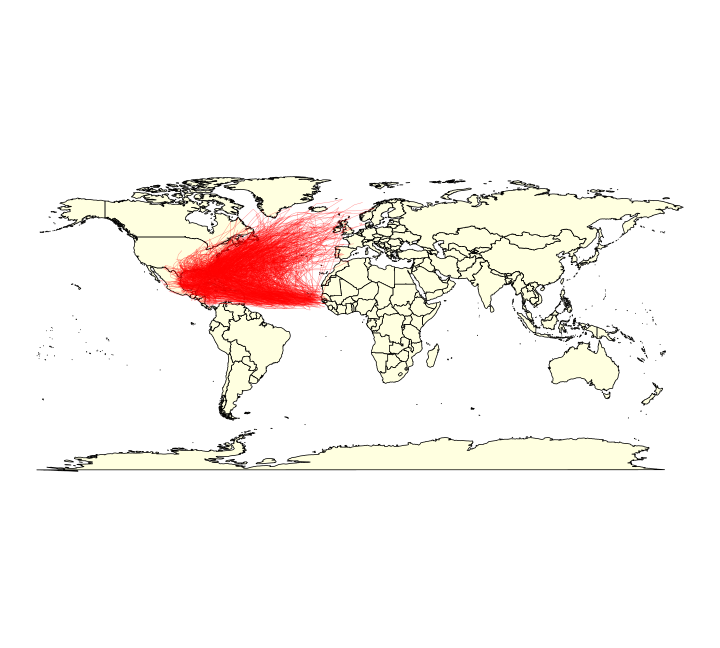# Shapefiles loc="http://freakonometrics.free.fr/Italy.zip" download.file(loc,destfile="Italy.zip") unzip("Italy.zip", exdir="./") library(rgdal,verbose=FALSE,quietly=TRUE,warn.conflicts=FALSE) ita1<-readOGR(dsn="./Italy/",layer="ITA_adm1",verbose=FALSE) names(ita1)  ##  "ID_0" "ISO" "NAME_0" "ID_1" "NAME_1" "NL_NAME_1" ##  "VARNAME_1" "TYPE_1" "ENGTYPE_1"  plot(ita1,col="light green")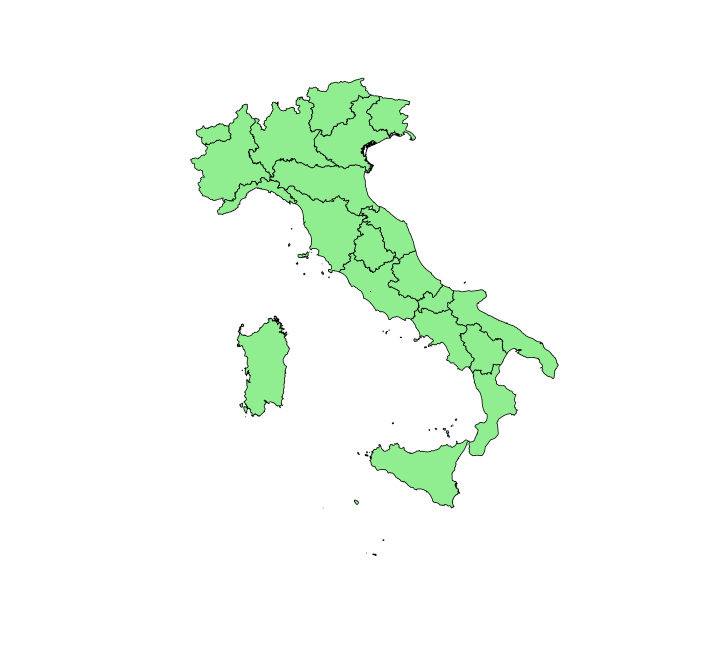ita1@data[,"NAME_1"][1:6]  ##  Abruzzo Apulia Basilicata Calabria Campania ##  Emilia-Romagna ## 20 Levels: Abruzzo Apulia Basilicata Calabria Campania ... Veneto  pos<-which(ita1@data[,"NAME_1"]=="Sicily") Poly_Sicily<-ita1[pos,]  plot(ita1,col="light green") plot(Poly_Sicily,col="yellow",add=TRUE)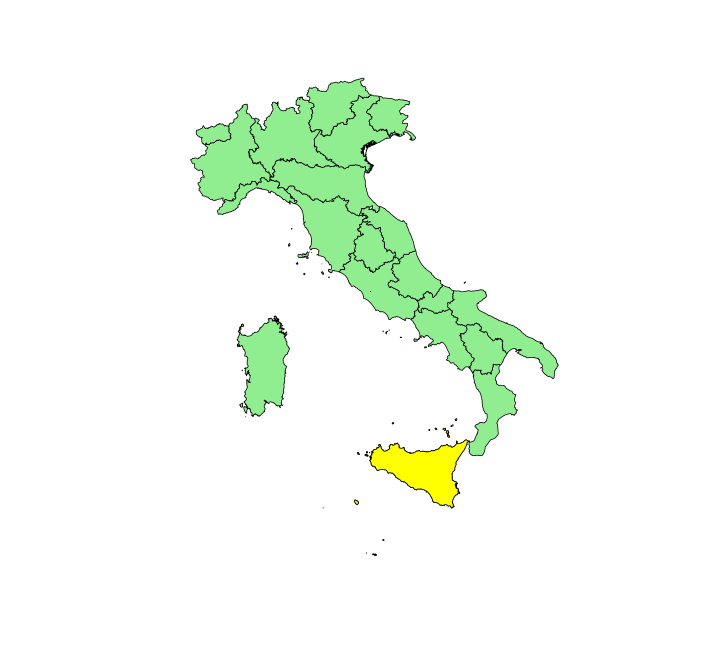plot(ita1,col="light green") plot(Poly_Sicily,col="yellow",add=TRUE) abline(v=5:20,col="light blue") abline(h=35:50,col="light blue") axis(1) axis(2)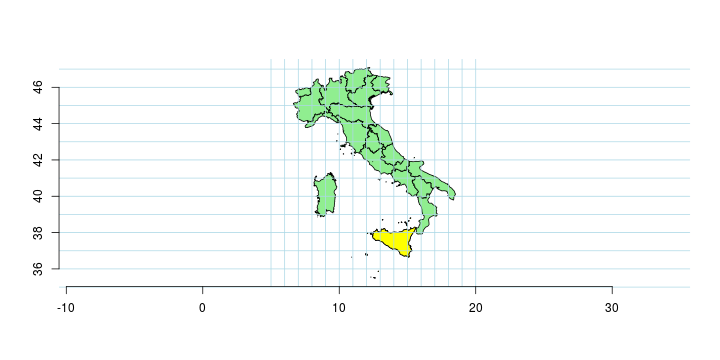pos<-which(ita1@data[,"NAME_1"] %in% c("Abruzzo","Apulia","Basilicata","Calabria","Campania","Lazio","Molise","Sardegna","Sicily")) ita_south <- ita1[pos,] ita_north <- ita1[-pos,] plot(ita1) plot(ita_south,col="red",add=TRUE) plot(ita_north,col="blue",add=TRUE)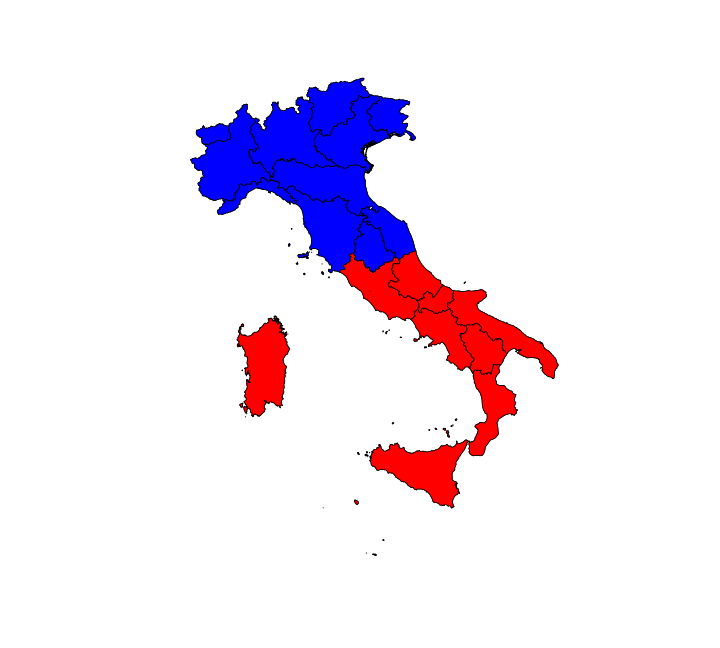library(xlsx,verbose=FALSE,quietly=TRUE,warn.conflicts=FALSE)  ## ## Attaching package: 'rJava'  ## The following object is masked from 'package:RCurl': ## ## clone  loc="http://freakonometrics.free.fr/Italy_codes.xlsx" download.file(loc,destfile="Italy_codes.xlsx") data_codes<-read.xlsx2(file = "Italy_codes.xlsx",sheetName="ITALY", startRow=1, header=TRUE) names(data_codes)="NAME_1" ita2<-merge(ita1, data_codes, by = "NAME_1", all.x=TRUE) library(rgeos,verbose=FALSE,quietly=TRUE,warn.conflicts=FALSE)  ## rgeos version: 0.3-21, (SVN revision 540) ## GEOS runtime version: 3.5.0-CAPI-1.9.0 r4084 ## Linking to sp version: 1.2-3 ## Polygon checking: TRUE  ita_s<-gUnionCascaded(ita_south) ita_n<-gUnionCascaded(ita_north)  plot(ita1) plot(ita_s,col="red",add=TRUE) plot(ita_n,col="blue",add=TRUE)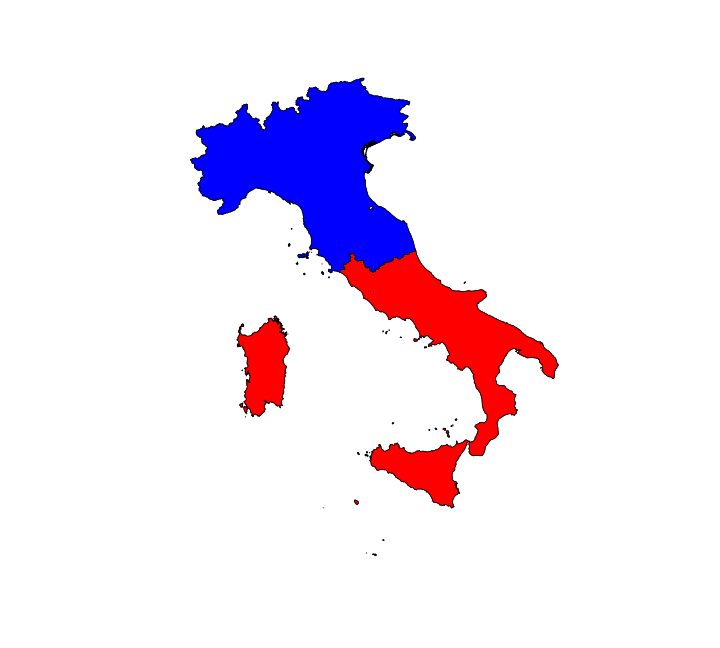plot(ita1,col="light green") plot(Poly_Sicily,col="yellow",add=TRUE) gCentroid(Poly_Sicily,byid=TRUE)  ## class : SpatialPoints ## features : 1 ## extent : 14.147, 14.147, 37.588, 37.588 (xmin, xmax, ymin, ymax) ## coord. ref. : +proj=longlat +datum=WGS84 +no_defs +ellps=WGS84 +towgs84=0,0,0  points(gCentroid(Poly_Sicily,byid=TRUE),pch=19,col="red")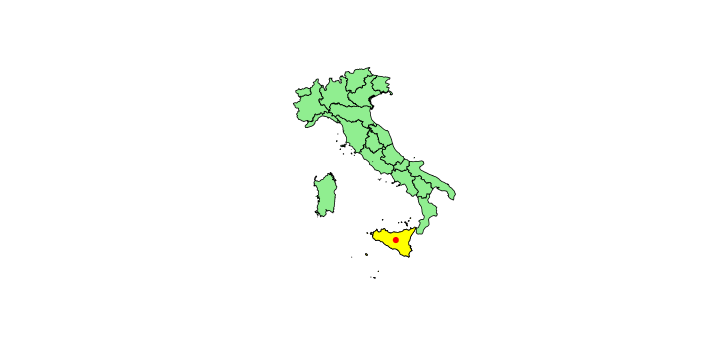G <- as.data.frame(gCentroid(ita1,byid=TRUE)) plot(ita1,col="light green") text(G$x,G$y,1:20)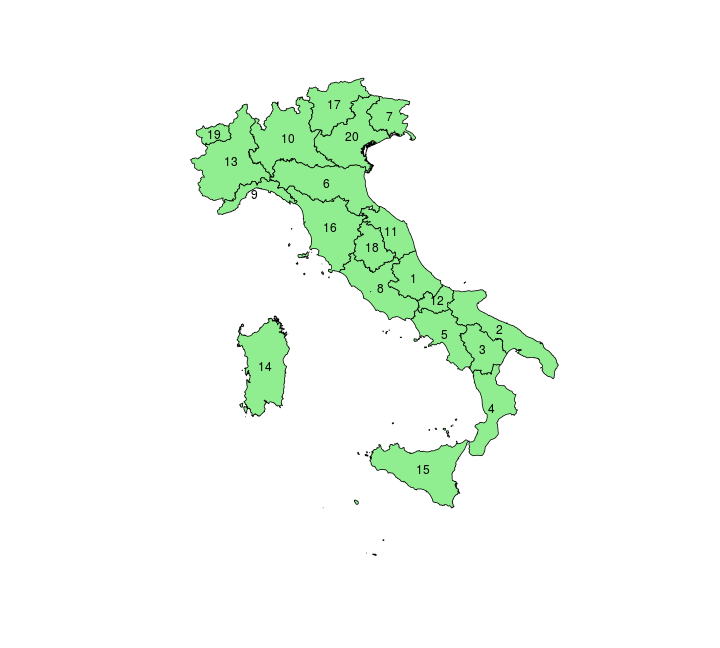c1 <-G[c(17,20,6,16,8,12,2),] c2 <- G[c(13,11,1,5,3,4),] L1<-SpatialLines(list(Lines(list(Line(c1)),"Line1"))) L2<-SpatialLines(list(Lines(list(Line(c2)),"Line2"))) L1@proj4string<-ita1@proj4string L2@proj4string<-ita1@proj4string cross_road <-gIntersection(L1,L2) cross_road@coords  ## x y ## 1 11.069 44.033 ## 1 14.200 41.749  plot(ita1,col="light green") plot(L1,col="red",lwd=2,add=TRUE) plot(L2,col="blue",lwd=2,add=TRUE) plot(cross_road,pch=19,cex=1.5,add=TRUE)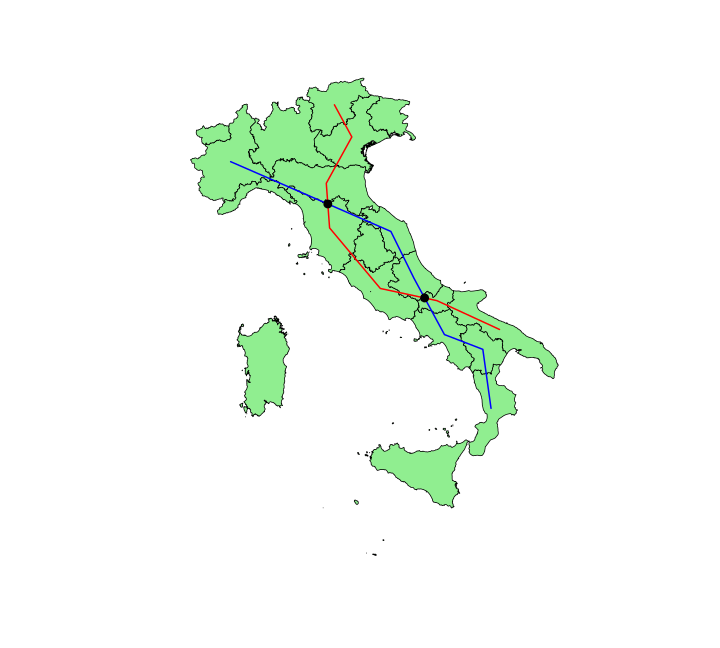## Linear Model $y_i=\boldsymbol{x}_i^{\text{T}}\boldsymbol{\beta}+\varepsilon_i=\beta_0 + [\beta_1 x_{1,i}+\cdots+ \beta_k x_{k,i}]+\varepsilon_i$ See linear model, where $\varepsilon_i\sim\mathcal{N}(0,\sigma^2)$ i.id. $(Y\vert \boldsymbol{X}=\boldsymbol{x})\sim \mathcal{N}(\boldsymbol{x}^{\text{T}}\boldsymbol{\beta}, \sigma^2)$ i.e. $\mathbb{E}[Y\vert\boldsymbol{X}=\boldsymbol{x}]=\boldsymbol{x}^{\text{T}}\boldsymbol{\beta}$ and homoscedastic model, $\text{Var}[Y\vert\boldsymbol{X}=\boldsymbol{x}]=\sigma^2$ Least squares (and maximum likelihood) estimator $\widehat{\boldsymbol{\beta}}=\text{argmin} \left\lbrace \sum_{i=1}^n (y_i-\boldsymbol{x}_i^{\text{T}}\boldsymbol{\beta})^2 \right\rbrace =(\boldsymbol{X}^{\text{T}}\boldsymbol{X})^{-1}\boldsymbol{X}^{\text{T}}\boldsymbol{y}$ ## Linear Model plot(cars)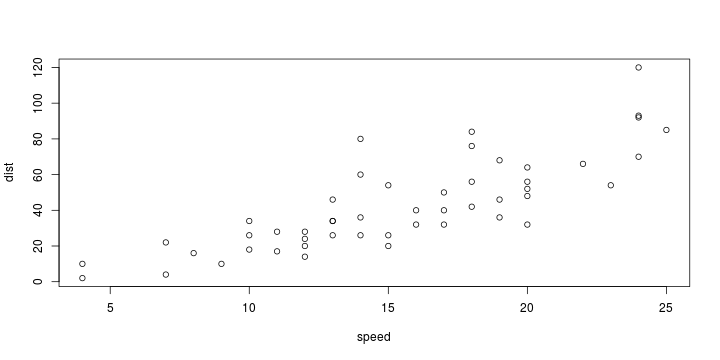model <- lm(dist ~ speed, data=cars)  ## Linear Model summary(model)  ## ## Call: ## lm(formula = dist ~ speed, data = cars) ## ## Residuals: ## Min 1Q Median 3Q Max ## -29.07 -9.53 -2.27 9.21 43.20 ## ## Coefficients: ## Estimate Std. Error t value Pr(>|t|) ## (Intercept) -17.579 6.758 -2.60 0.012 * ## speed 3.932 0.416 9.46 1.5e-12 *** ## --- ## Signif. codes: 0 '***' 0.001 '**' 0.01 '*' 0.05 '.' 0.1 ' ' 1 ## ## Residual standard error: 15.4 on 48 degrees of freedom ## Multiple R-squared: 0.651, Adjusted R-squared: 0.644 ## F-statistic: 89.6 on 1 and 48 DF, p-value: 1.49e-12  ## Linear Model X=cbind(1,cars$speed)
Y=cars$dist solve(crossprod(X,X),crossprod(X,Y))  ## [,1] ## [1,] -17.5791 ## [2,] 3.9324  model$coefficients

## (Intercept)       speed
##    -17.5791      3.9324


summary(model)$sigma^2*solve(crossprod(X,X))  ## [,1] [,2] ## [1,] 45.6765 -2.65882 ## [2,] -2.6588 0.17265  vcov(model)  ## (Intercept) speed ## (Intercept) 45.6765 -2.65882 ## speed -2.6588 0.17265  n=nrow(cars)  ## Linear Model confint(model)  ## 2.5 % 97.5 % ## (Intercept) -31.168 -3.9903 ## speed 3.097 4.7679  model$coefficients+qt(c(.025,.975),n-2)* summary(model)$coefficients[1,2]  ##  -31.1678 -3.9903  ## Linear Model coefficient of determination $\displaystyle{R^2 = \frac{\text{explained variance}}{\text{total variance}}}$ summary(model)$r.squared

##  0.65108

1-deviance(model)/sum((Y-mean(Y))^2)

##  0.65108


$\text{Var}[Y]= \text{Var}[\mathbb{E}[Y\vert X]]+ \mathbb{E}[\text{Var}[Y\vert X]]$

## Linear Model

$\overline{R}^2 = 1-[1-R^2]\cdot \frac{n-1}{n-(k-1)-1}$

summary(model)$adj.r.squared  ##  0.64381  ## Linear Model anova(lm(dist~speed,data=cars),lm(dist~1,data=cars))  ## Analysis of Variance Table ## ## Model 1: dist ~ speed ## Model 2: dist ~ 1 ## Res.Df RSS Df Sum of Sq F Pr(>F) ## 1 48 11354 ## 2 49 32539 -1 -21185 89.6 1.5e-12 *** ## --- ## Signif. codes: 0 '***' 0.001 '**' 0.01 '*' 0.05 '.' 0.1 ' ' 1  ## Linear Model plot(cars) abline(model,col="red")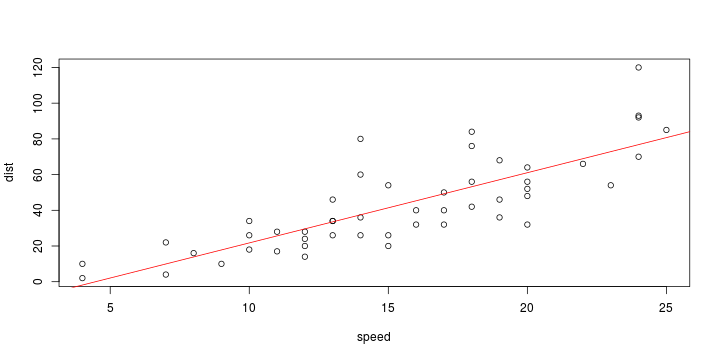## Linear Model plot(cars) abline(model,col="red") x=seq(2,26) y=predict(model, newdata=data.frame(speed=x)) lines(x,y,lwd=2,col="blue")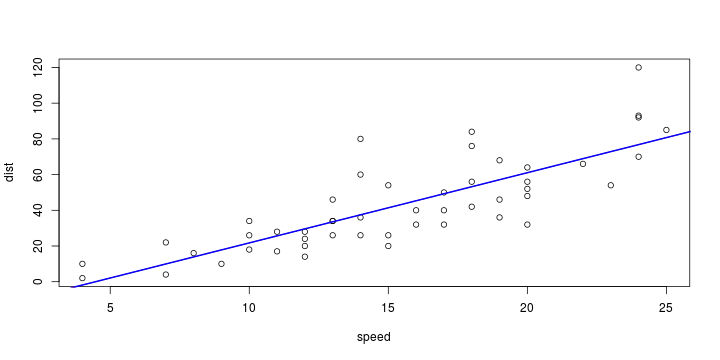## Linear Model y=predict(model, newdata=data.frame(speed=x), interval = "confidence") head(y)  ## fit lwr upr ## 1 -9.7143 -21.7331 2.3045 ## 2 -5.7819 -17.0266 5.4629 ## 3 -1.8495 -12.3295 8.6306 ## 4 2.0829 -7.6442 11.8100 ## 5 6.0154 -2.9733 15.0041 ## 6 9.9478 1.6790 18.2166  $\text{Var}[\widehat{Y}(\boldsymbol{x})]=\text{Var}[\boldsymbol{x}^{\text{T}}\widehat{\boldsymbol{\beta}}]=\boldsymbol{x}^{\text{T}}\text{Var}[\widehat{\boldsymbol{\beta}}]\boldsymbol{x} =\widehat{\sigma}^2 \boldsymbol{x}^{\text{T}}[\boldsymbol{X}^{\text{T}}\boldsymbol{X}]^{-1}\boldsymbol{x}$ ## Linear Model plot(cars) polygon(c(x,rev(x)),c(y[,2],rev(y[,3])),col=rgb(0,0,1,.4),border=NA) lines(x,y[,1],lwd=2,col="blue")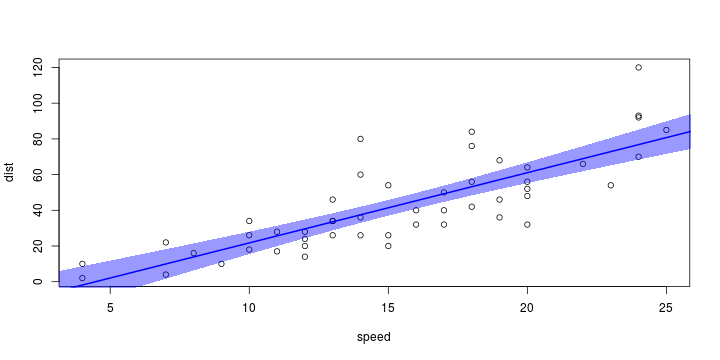## Linear Model and Bagging Method 1 Y=matrix(NA,1000,length(x)) for(b in 1:1000){ idx <- sample(1:nrow(cars),size=nrow(cars),replace=TRUE) modelb <- lm(dist ~ speed, data=cars[idx,]) Y[b,] <- predict(modelb, newdata=data.frame(speed=x)) }  See bootstrap, based on pseudo-sample $\lbrace(\boldsymbol{x}_{i_1},y_{i_1}), \cdots,(\boldsymbol{x}_{i_n},y_{i_n}) \rbrace$ where $(i_1,\cdots,i_n)\in\lbrace 1,2,\cdots,n\rbrace$. ## Linear Model and Bagging Method 1 plot(cars) for(b in 1:100) lines(x,Y[b,],col=rgb(0,0,1,.4))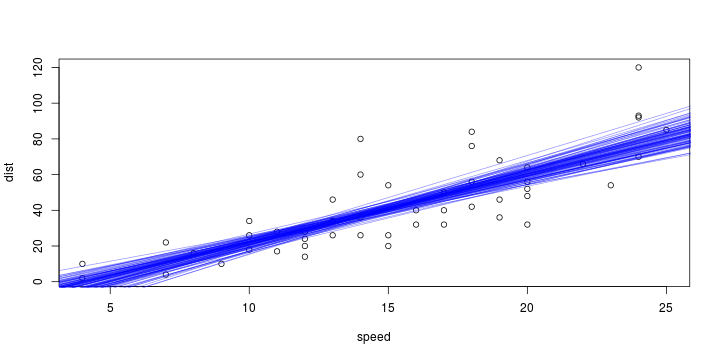## Linear Model and Bagging plot(cars) lines(x,apply(Y,2,mean),col="blue",lwd=2) lines(x,apply(Y,2,function(x) quantile(x,.025)),col="red",lty=2) lines(x,apply(Y,2,function(x) quantile(x,.975)),col="red",lty=2)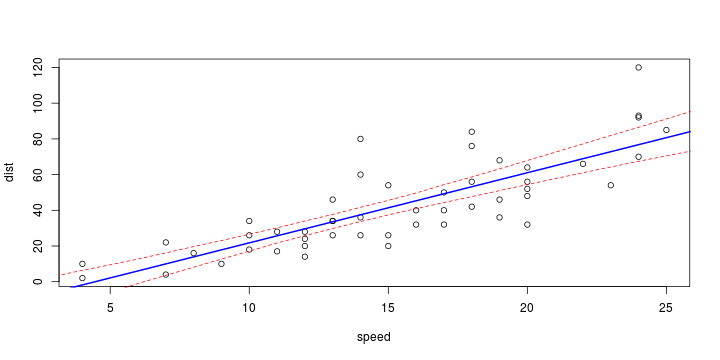## Linear Model and Bagging Method 2 pred_dist=predict(model) epsilon =residuals(model) Y=matrix(NA,1000,length(x)) for(b in 1:1000){ idx <- sample(1:nrow(cars),size=nrow(cars),replace=TRUE) carsb=data.frame(speed=cars$speed,
dist=pred_dist+epsilon[idx])
modelb <- lm(dist ~ speed, data=carsb)
Y[b,] <- predict(modelb, newdata=data.frame(speed=x))
}


See bootstrap, based on pseudo-sample $\lbrace(\boldsymbol{x}_{1},\widehat{y}_{1}+\widehat{\varepsilon}_{i_1}), \cdots,(\boldsymbol{x}_{n},\widehat{y}_{n}+\widehat{\varepsilon}_{i_n}) \rbrace$ where $(i_1,\cdots,i_n)\in\lbrace 1,2,\cdots,n\rbrace$.

## Linear Model and Bagging

Method 2

plot(cars)
for(b in 1:100) lines(x,Y[b,],col=rgb(0,0,1,.4))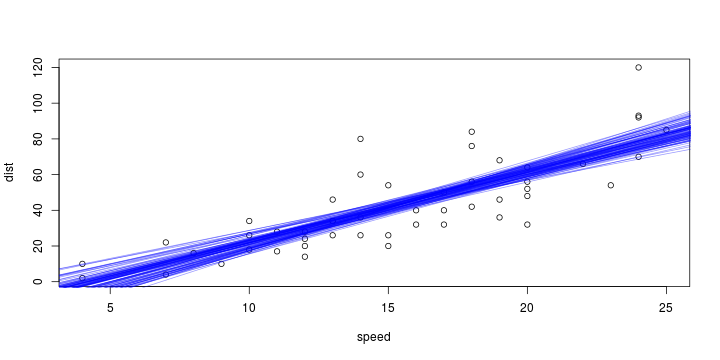## Linear Model and Bagging

plot(cars)
lines(x,apply(Y,2,mean),col="blue",lwd=2)
lines(x,apply(Y,2,function(x) quantile(x,.025)),col="red",lty=2)
lines(x,apply(Y,2,function(x) quantile(x,.975)),col="red",lty=2)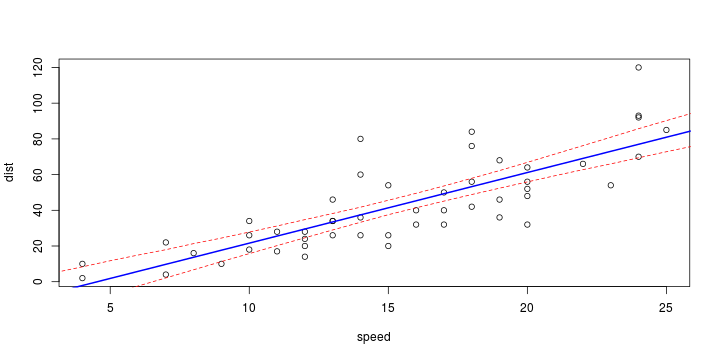## Errors

plot(cars)
abline(model,col="red")
segments(cars$speed,cars$dist,cars$speed,predict(model),col="blue")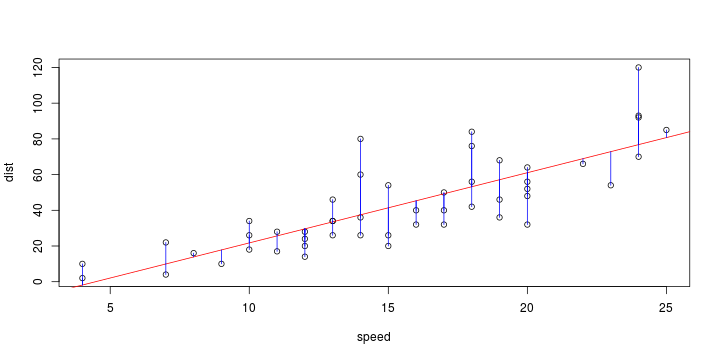## Least Squares? cars2=cars; cars2[,2]=cars[,2]/10 plot(cars2,ylab="dist/10") acp=princomp(cars2) b=acp$loadings[2,1]/acp$loadings[1,1] a=acp$center-b*acp$center abline(a,b,col="red")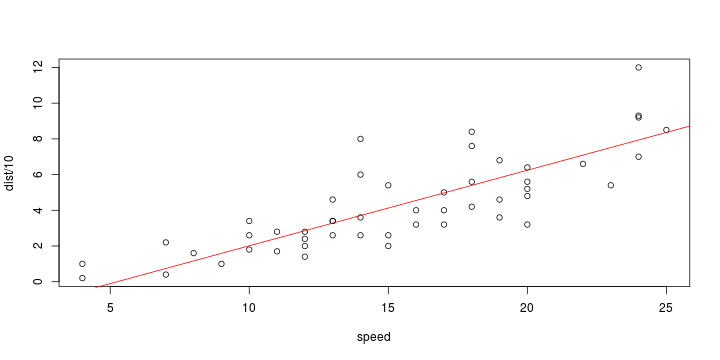## Least Squares? plot(cars2,ylab="dist/10",xlim=c(0,30),ylim=c(-1,12)) abline(a,b,col="red") t <- acp$loadings[,1] %*% (t(cars2)-acp$center) X1 <- acp$center +t * acp$loadings[1,1] X2 <- acp$center +t * acp$loadings[2,1] segments(cars2$speed,cars2$dist,X1,X2,col="blue")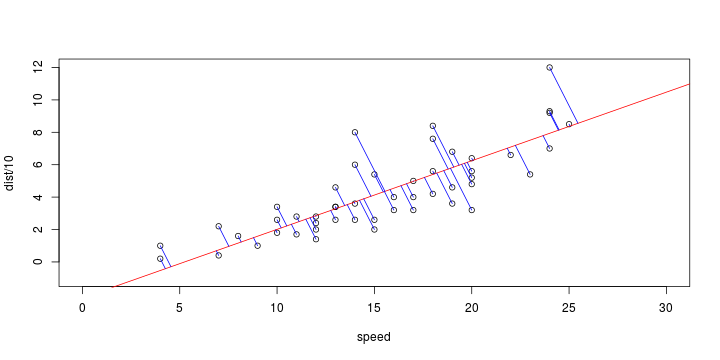## Least Absolute Deviation  f <- function(a) sum(abs(cars$dist-(a+a*cars$speed))) opt <- optim( c(0,0), f )$par
plot(cars)
abline(model, col='red', lty=2)
abline(opt, opt,col="blue")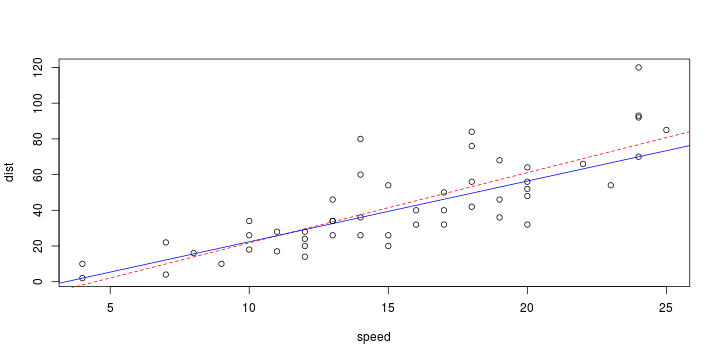## Quantile Regression

library(quantreg,verbose=FALSE,quietly=TRUE,warn.conflicts=FALSE)
plot(cars)
abline(model, col="blue")
abline(rq(dist ~ speed,data=cars, tau=.5),col="red",lty=2)
abline(rq(dist ~ speed,data=cars, tau=.9),col="purple",lty=2)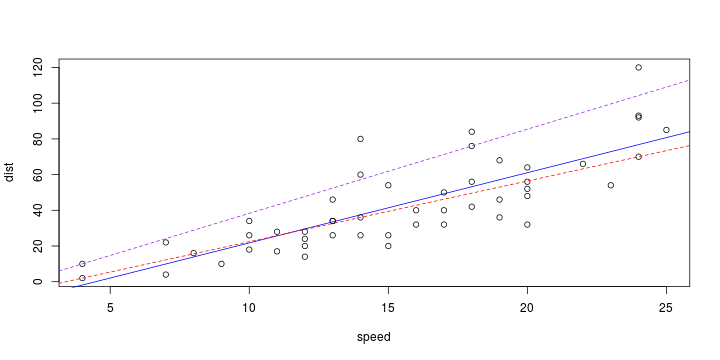## Diagnostic and Errors

plot(model,which=1)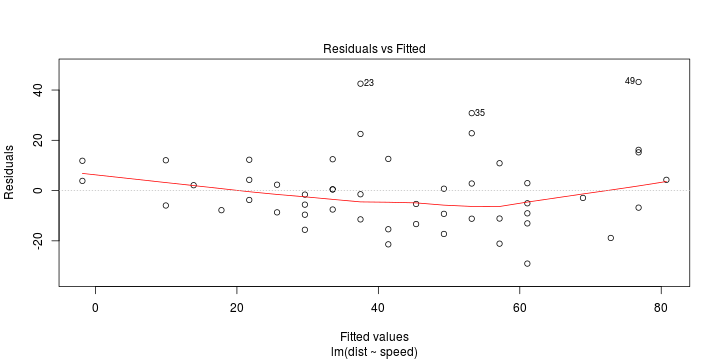Scatterplot $(\widehat{Y}_i,\widehat{\varepsilon}_i)$

## Diagnostic and Errors

plot(model,which=2)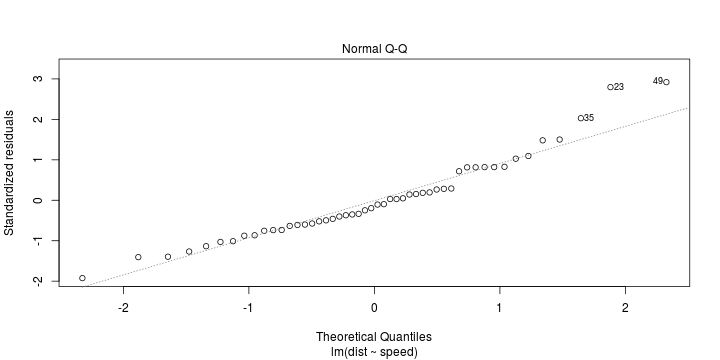Scatterplot $\displaystyle{\left(\widehat{\varepsilon}_{i:n},\Phi^{-1} \left(\frac{i}{n}\right)\right)}$

## Diagnostic and Errors

plot(model,which=3)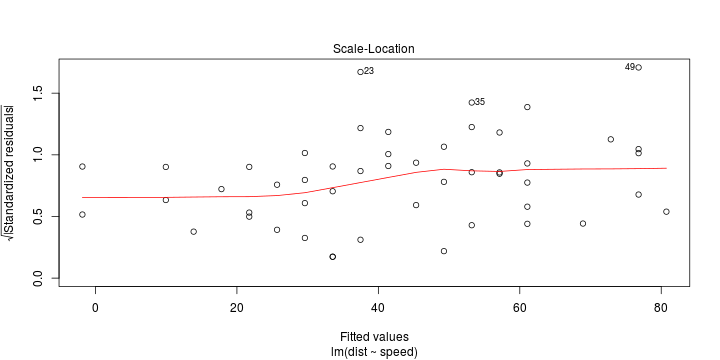Scatterplot $(\widehat{Y}_i,\sqrt{\vert\widehat{\varepsilon}_i\vert})$

## Diagnostic and Errors

plot(model,which=4)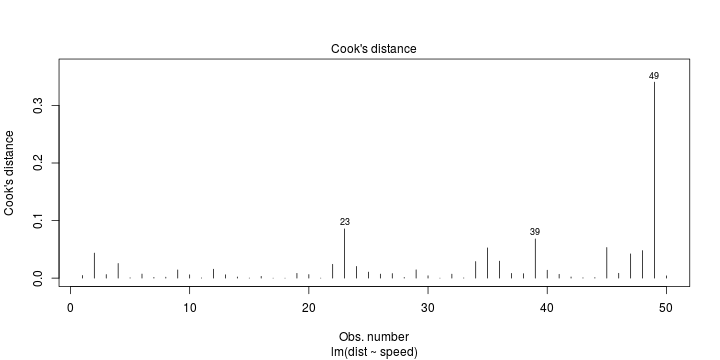Cook distance, $\displaystyle{C_i=\frac{\widehat{\varepsilon}_i^2}{p\cdot\text{MSE}}\cdot\left(\frac{H_{i,i}}{(1-H_{i,i})^2}\right)}$ with $\boldsymbol{H}=\boldsymbol{X}(\boldsymbol{X}^{\text{T}}\boldsymbol{X})^{-1}\boldsymbol{X}^{\text{T}}=[H_{i,i}]$

C=cooks.distance(model)


## Diagnostic and Errors

$H_{i,i}$ are leverages, and define Studentized residuals as $\widehat{r}_i=\frac{\widehat{\varepsilon}_i}{ \widehat{\sigma} \sqrt{1-H_{i,i}}}$

rstudent(model)

##         1         2         3         4         5         6         7         8
##  0.263450  0.816078 -0.397812  0.810353  0.140703 -0.517161 -0.246246  0.279834
##         9        10        11        12        13        14        15        16
##  0.810904 -0.570047  0.152092 -1.030378 -0.629925 -0.366705 -0.105093 -0.492517
##        17        18        19        20        21        22        23        24
##  0.029817  0.029817  0.817162 -0.750784 -0.095921  1.499720  3.022829 -1.420977
##        25        26        27        28        29        30        31        32
## -1.012276  0.824408 -0.874115 -0.347522 -1.139035 -0.605535  0.047371 -0.734220
##        33        34        35        36        37        38        39        40
##  0.182229  1.521459  2.098482 -1.409292 -0.731459  0.713309 -1.982389 -0.862936
##        41        42        43        44        45        46        47        48
## -0.596376 -0.332475  0.192085 -0.193933 -1.274939 -0.455573  1.027735  1.097019
##        49        50
##  3.184993  0.287745


## Diagnostic and Errors

plot(model,which=5)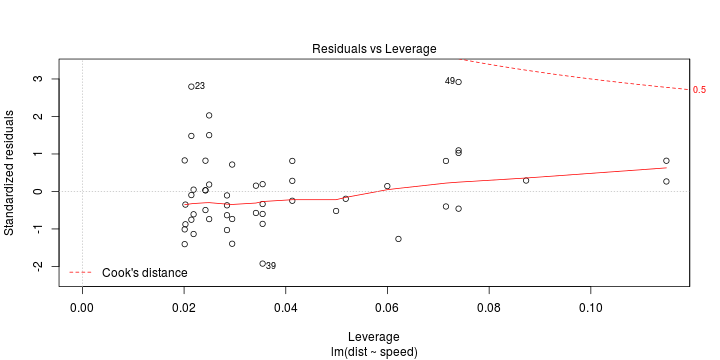Scatterplot $(H_{i,i},\widehat{r}_i)$

## Diagnostic and Errors

plot(model,which=6)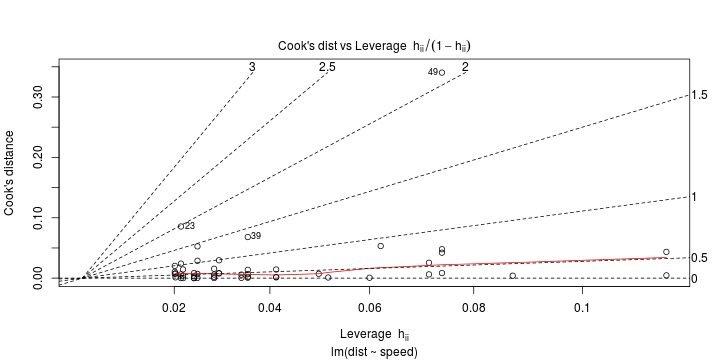Scatterplot $(H_{i,i},C_i)$

## Diagnostic and Errors

hist(residuals(model),probability=TRUE, col="light green")
lines(density(residuals(model)),lwd=2,col="red")
pars=list(boxwex=.004),col=rgb(0,0,1,.25))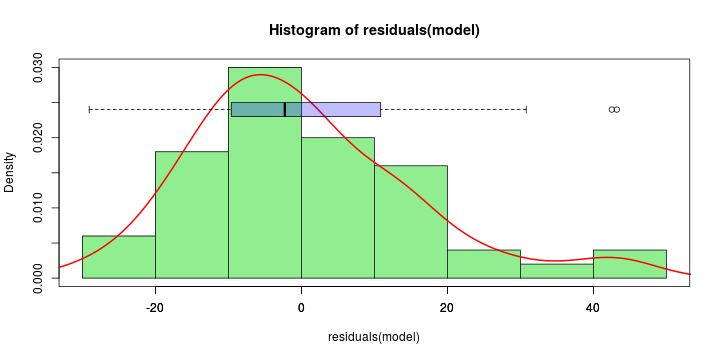sigma=summary(model)$sigma plot(ecdf(residuals(model)/sigma)) lines(seq(-3,3,by=.1),pnorm(seq(-3,3,by=.1)),lwd=2,col="red")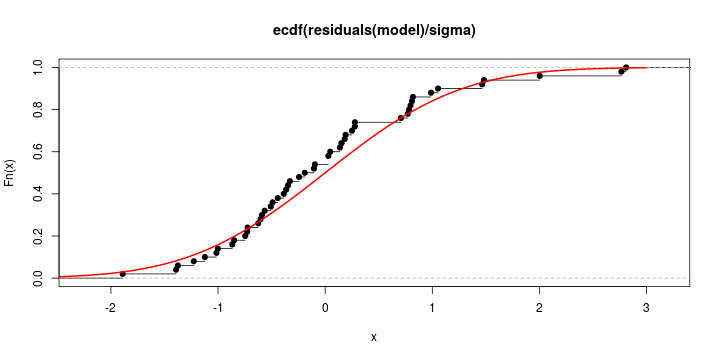## Diagnostic and Errors Kolmogorov-Smirnov$ d=\sup_{x\in\mathbb{R}}\lbrace \vert\Phi(x)-\widehat{F}_n(x)\vert \rbrace $ks.test(residuals(model)/sigma,"pnorm")  ## Warning in ks.test(residuals(model)/sigma, "pnorm"): ties should not be present ## for the Kolmogorov-Smirnov test  ## ## One-sample Kolmogorov-Smirnov test ## ## data: residuals(model)/sigma ## D = 0.131, p-value = 0.36 ## alternative hypothesis: two-sided  ## Diagnostic and Errors library(nortest,verbose=FALSE,quietly=TRUE,warn.conflicts=FALSE) ad.test(residuals(model))  ## ## Anderson-Darling normality test ## ## data: residuals(model) ## A = 0.794, p-value = 0.037  cvm.test(residuals(model))  ## ## Cramer-von Mises normality test ## ## data: residuals(model) ## W = 0.126, p-value = 0.048  ## Model Choice AIC $AIC = 2k - 2\log(\mathcal{L}) = 2k + n\left[\log\left(2\pi \frac{1}{n}\sum_{i=1}^n \widehat{\varepsilon}_i^2 \right) + 1\right]$ BIC $BIC = { k \log(n) -2 \log(\mathcal{L}) } = k \log(n) + n\left[\log\left(2\pi \frac{1}{n}\sum_{i=1}^n \widehat{\varepsilon}_i^2 \right) + 1\right]$ AIC(model)  ##  419.16  AIC(model,k=log(n))  ##  424.89  ## Testing Linear Assumptions library(splines,verbose=FALSE,quietly=TRUE,warn.conflicts=FALSE) model_brk <- lm(dist ~ bs(speed,degree=1,knots=(c(4,15,25))), data=cars) x=seq(4,25,by=.1) y=predict(model_brk, newdata=data.frame(speed=x))  ## Warning in predict.lm(model_brk, newdata = data.frame(speed = x)): prediction ## from a rank-deficient fit may be misleading  see $b$-splines, $y_i=\beta_0+\beta_1 x_i + \beta_2 (x_i-15)_+ + \varepsilon_i$ ## Testing Linear Assumptions plot(cars) lines(x,y,lwd=2,col="blue")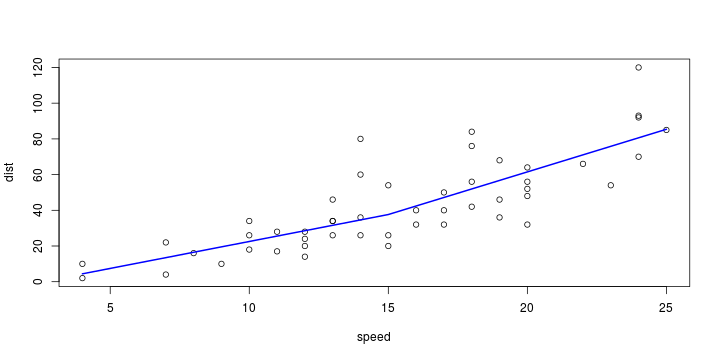## Testing Linear Assumptions positive=function(x,s) ifelse(x>s,x-s,0) model_brk <- lm(dist ~ speed + positive(speed,15), data=cars) x=seq(4,25,by=.1) y=predict(model_brk, newdata=data.frame(speed=x)) plot(cars) abline(model,col="red",lty=2) lines(x,y,lwd=2,col="blue")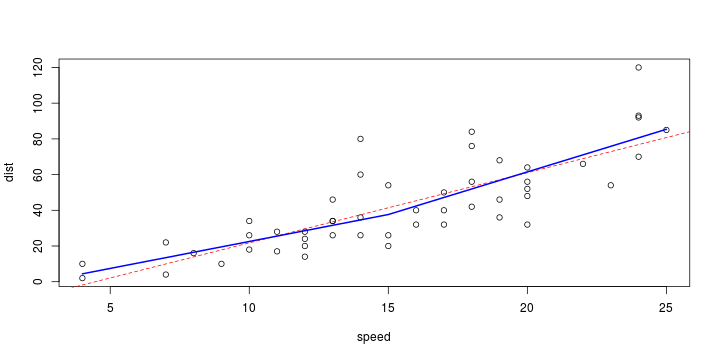## Testing Linear Assumptions plot(cars) abline(model,col="red",lty=2) lines(x,y,lwd=2,col="blue") abline(coefficients(model_brk),coefficients(model_brk),col="blue",lty=2)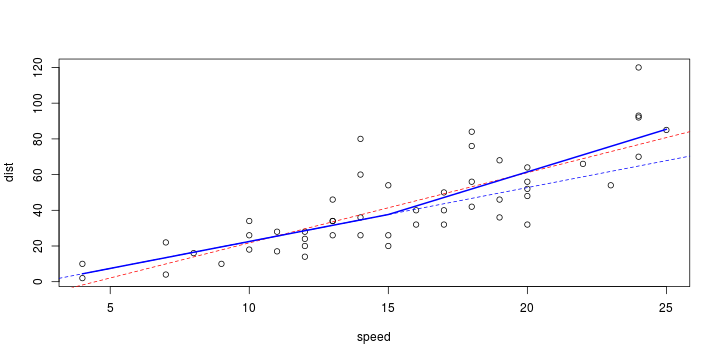summary(model_brk)$coefficients

##                     Estimate Std. Error  t value  Pr(>|t|)
## (Intercept)          -7.6519   10.62536 -0.72016 0.4749955
## speed                 3.0186    0.86268  3.49916 0.0010324
## positive(speed, 15)   1.7562    1.45513  1.20694 0.2334961


## Testing Linear Assumptions

library(strucchange,verbose=FALSE,quietly=TRUE,warn.conflicts=FALSE)
plot(Fstats(dist ~ speed,data=cars,from=7/50))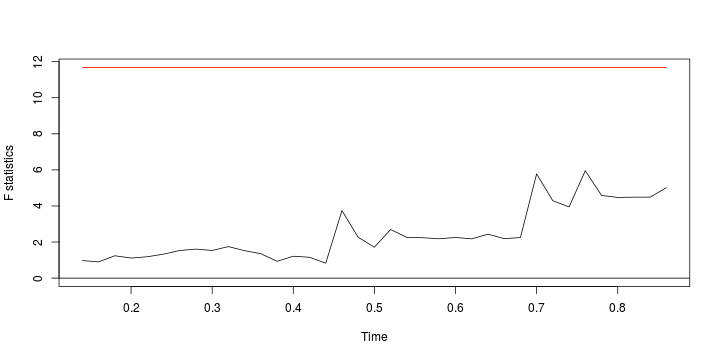see Chow Test

## Testing Linear Assumptions

$W_t=\frac{1}{\widehat{\sigma}\sqrt{n}}\sum_{i=1}^{ \lfloor nt \rfloor} \widehat{\varepsilon}_i$

cusum <- efp(dist ~ speed, type = "OLS-CUSUM",data=cars)
plot(cusum,ylim=c(-2,2))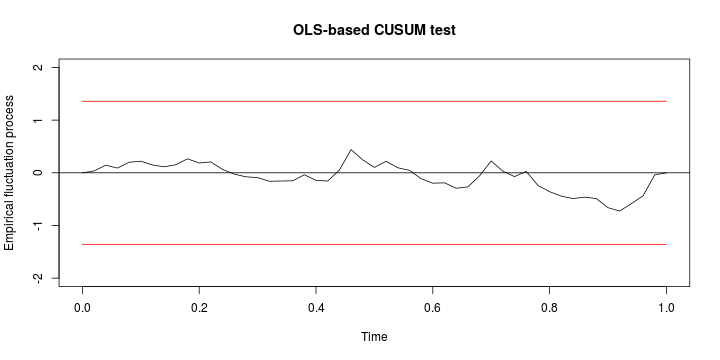## Testing Linear Assumptions

plot(cusum, alpha = 0.05, alt.boundary = TRUE,ylim=c(-2,2))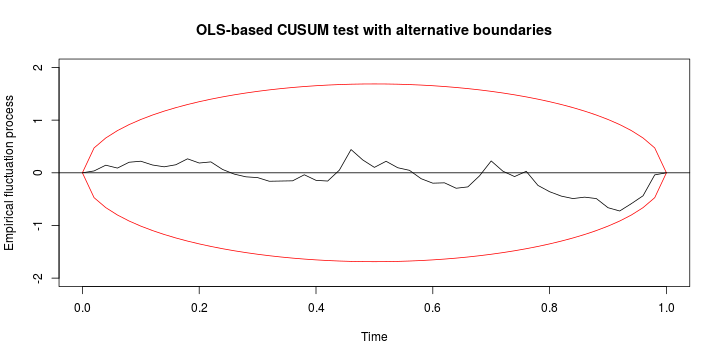see CUSUM test

## Model with Categorical Covariates

model_cut=lm(dist~ cut(speed, breaks=c(0,10,15,20,25)),data=cars)
y=predict(model_cut, newdata=data.frame(speed=x))
plot(cars)
abline(model,col="red",lty=2)
lines(x,y,lwd=2,col="blue",type="s")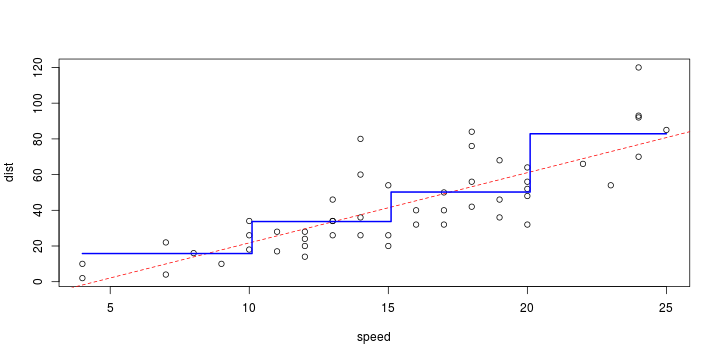## Model with Categorical Covariates

library(rpart,verbose=FALSE,quietly=TRUE,warn.conflicts=FALSE)
tree=rpart(dist~speed,data=cars)
y=predict(tree,newdata=data.frame(speed=x))
plot(cars)
abline(model,col="red",lty=2)
lines(x,y,lwd=2,col="blue",type="s")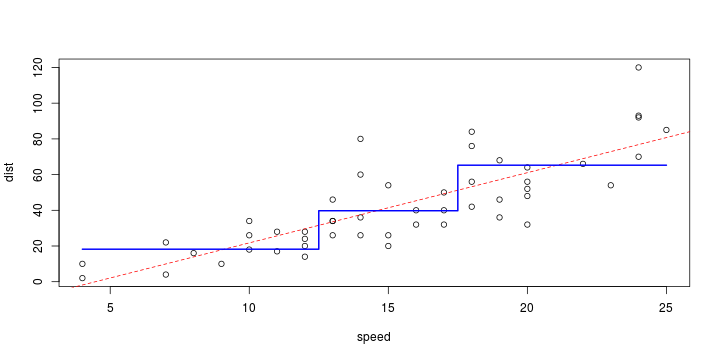## Smoothing : Polynomial Regression

model_poly=lm(dist~ poly(speed, df=3),data=cars)
y=predict(model_poly, newdata=data.frame(speed=x))
plot(cars)
abline(model,col="red",lty=2)
lines(x,y,lwd=2,col="blue")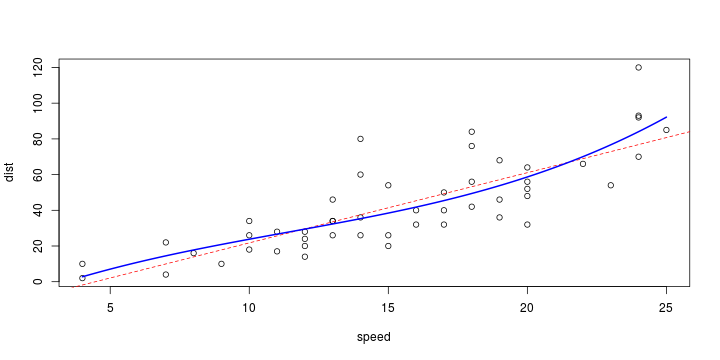## Smoothing : Local Regression

library(KernSmooth,verbose=FALSE,quietly=TRUE,warn.conflicts=FALSE)
plot(cars)
bw <- dpill(cars$speed,cars$dist)
lines( locpoly(cars$speed,cars$dist,degree=0, bandwidth=bw), col='red' )
lines( locpoly(cars$speed,cars$dist,degree=1, bandwidth=bw), col='green' )
lines( locpoly(cars$speed,cars$dist,degree=2, bandwidth=bw), col='blue' )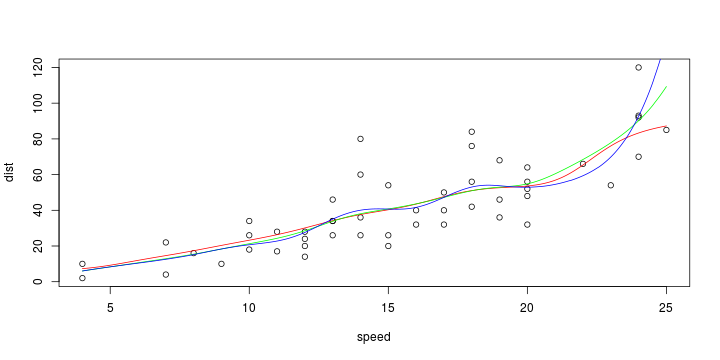## Smoothing : $k$-Nearest Neighboors

library(FNN,verbose=FALSE,quietly=TRUE,warn.conflicts=FALSE)
knn=knn.reg(train=cars$speed,y=cars$dist,k=5)
plot(cars)
lines(cars$speed,knn$pred,col="red")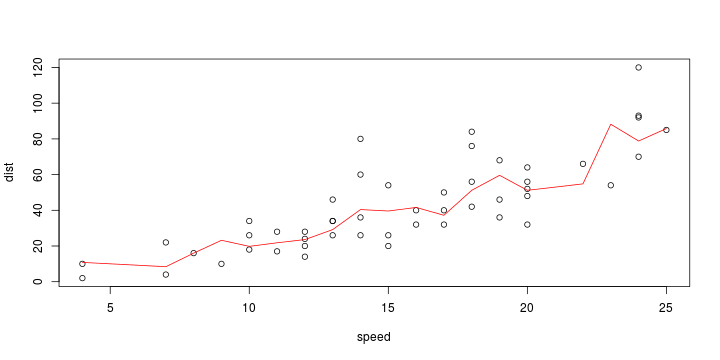## Smoothing : $b$ Splines

library(splines,verbose=FALSE,quietly=TRUE,warn.conflicts=FALSE)
model_bs <- lm(dist ~ bs(speed), data=cars)
y=predict(model_bs, newdata=data.frame(speed=x))
plot(cars)
abline(model,col="red",lty=2)
lines(x,y,lwd=2,col="blue")## Smoothing Linear Model

Tuning parameter selection, Silverman's Rule $b^\star=0.9 \cdot\frac{ \min\lbrace \sigma,F^{-1}(.75)-F^{-1}(.25)\rbrace}{1.34 \cdot n^{1/5}}$

bw.nrd0(cars$speed)  ##  2.15  ## Smoothing Linear Model Tuning parameter selection, Cross Validation $b^\star=\text{argmin}\left\lbrace \sum_{i=1}^n \left(y_i - \widehat{m}_{(i)}(\boldsymbol{x}_i)\right)^2\right\rbrace$ bw.ucv(cars$speed)

## Warning in bw.ucv(cars$speed): minimum occurred at one end of the range  ##  2.7489  ## Smoothing Linear Model library(KernSmooth,verbose=FALSE,quietly=TRUE,warn.conflicts=FALSE) Nadaraya_Watson =with(cars,ksmooth(speed, dist, "normal",bandwidth=2.75)) plot(cars) abline(model,col="red",lty=2) lines(Nadaraya_Watson,lwd=2,col="blue")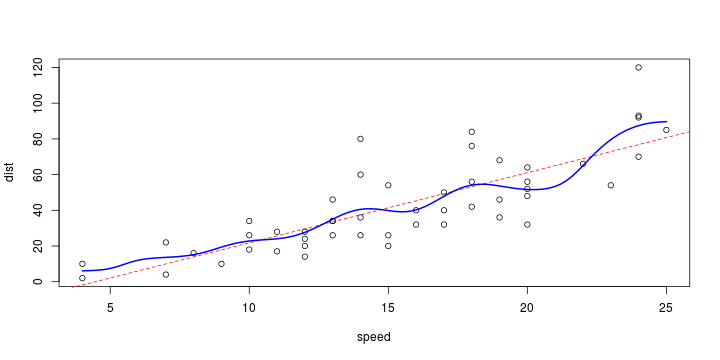## Smoothing Linear Model library(KernSmooth,verbose=FALSE,quietly=TRUE,warn.conflicts=FALSE) model_loess=loess(dist~ speed, data=cars,degree=1, family="gaussian") y=predict(model_loess, newdata=data.frame(speed=x)) plot(cars) abline(model,col="red",lty=2) lines(x,y,lwd=2,col="blue")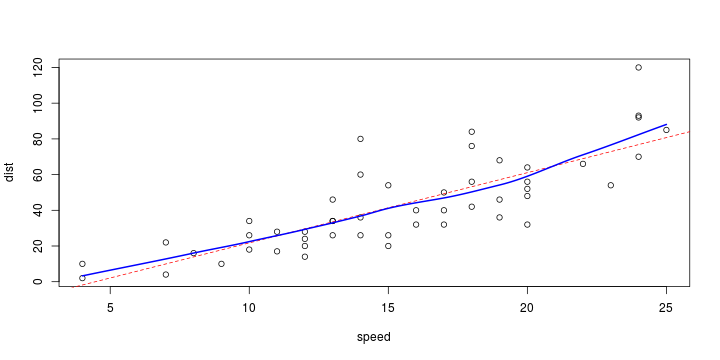## Multiple Regression Life Expectancy (1), Homicide Rate (2), Illiteracy Rate (3) chicago=read.table("http://freakonometrics.free.fr/chicago.txt",header=TRUE,sep=";") model_c = lm(Fire~X_2+X_3,data=chicago) y=function(x2,x3) predict(model_c,newdata=data.frame(X_2=x2,X_3=x3)) VX2=seq(0,80,length=26); VX3=seq(5,25,length=26) VY=outer(VX2,VX3,y) persp(VX2,VX3,VY,xlab="Homicide",ylab="Illiteracy",zlab="Fire",theta=20)## Multiple Regression VX2=seq(0,80,length=251); VX3=seq(5,25,length=251) VY=outer(VX2,VX3,y) image(VX2,VX3,VY,xlab="Homicide",ylab="Illiteracy") contour(VX2,VX3,VY,add=TRUE)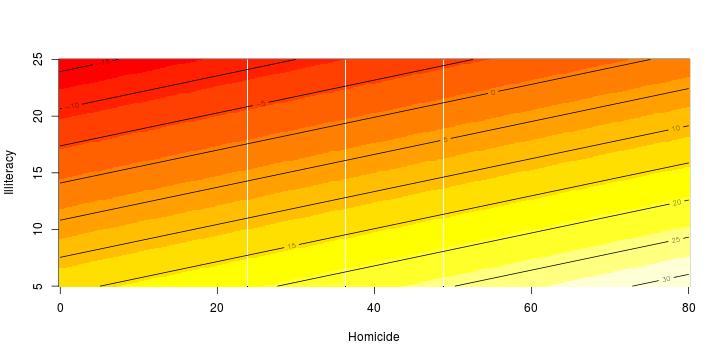## Model selection model_c = lm(Fire~.,data=chicago) summary(model_c)$r.squared

##  0.44167

summary(model_c)$adj.r.squared  ##  0.40272  ## Model selection logLik(model_c)  ## 'log Lik.' -152.77 (df=5)  AIC(model_c, k=2) # AIC  ##  315.54  AIC(model_c, k=log(nrow(cars))) # BIC  ##  325.1  ## Penalization $\widehat{\boldsymbol{\beta}} = \text{argmin}\left\lbrace \sum_{i=1}^n \left[y_i- \left(\beta_0+\sum_{j=1}^k \beta_j x_{j,i} \right)\right] + \color{red}{\lambda} \sum_{j=1}^k \beta_j ^2\right\rbrace$ with an explicit solution $\widehat{\boldsymbol{\beta}}=(\boldsymbol{X}^{\text{T}}\boldsymbol{X}-\color{red}{\lambda} \mathbb{I})^{-1} \boldsymbol{X}^{\text{T}}\boldsymbol{Y}$. library(MASS,verbose=FALSE,quietly=TRUE,warn.conflicts=FALSE) model_ridge <- lm.ridge(Fire ~ ., data=chicago, lambda=1)  see more generally Tikhonov regularization ## Optimal Penalization mse <- NULL n=100 v <- matrix(c(0,coefficients(model_c)[-1]), nr=n, nc=4, byrow=TRUE) kl <- c(1e-4, 2e-4, 5e-4, 1e-3, 2e-3, 5e-3, 1e-2, 2e-2, 5e-2, .1, .2, .3, .4, .5, .6, .7, .8, .9, 1, 1.2, 1.4, 1.6, 1.8, 2) for (k in kl) { r <- matrix(NA, nr=n, nc=4) for (i in 1:n) { boot_c <- chicago[sample(1:nrow(chicago),nrow(chicago),replace=TRUE),] r[i,2:4] <- model_ridge <- lm.ridge(Fire ~ ., data=boot_c, lambda=k)$coef
r[i,1] <- mean(boot_c[,"Fire"])
}
mse <- append(mse, apply( (r-v)^2, 2, mean ))
}


## Optimal Penalization

plot( mse ~ kl, type='l' )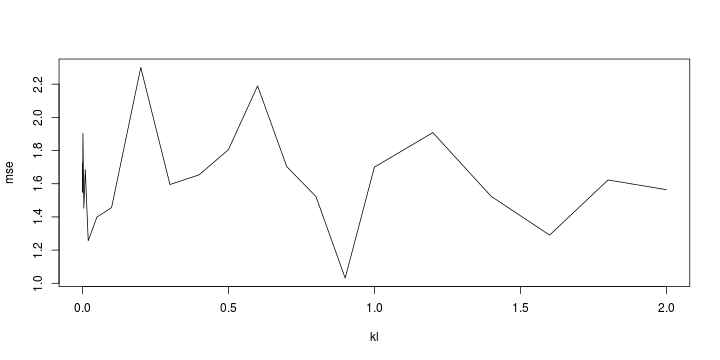## Variable Selection

step(lm(Fire ~ .,data=chicago))

## Start:  AIC=180.16
## Fire ~ X_1 + X_2 + X_3
##
##        Df Sum of Sq  RSS AIC
## - X_1   1         1 1832 178
## <none>              1832 180
## - X_2   1       562 2394 191
## - X_3   1       702 2534 193
##
## Step:  AIC=178.17
## Fire ~ X_2 + X_3
##
##        Df Sum of Sq  RSS AIC
## <none>              1832 178
## - X_2   1       621 2453 190
## - X_3   1      1004 2836 197

##
## Call:
## lm(formula = Fire ~ X_2 + X_3, data = chicago)
##
## Coefficients:
## (Intercept)          X_2          X_3
##      21.496        0.221       -1.525


## Variable Selection

LASSO (least absolute shrinkage and selection operator) $\widehat{\boldsymbol{\beta}} = \text{argmin}\left\lbrace \sum_{i=1}^n \left[y_i- \left(\beta_0+\sum_{j=1}^k \beta_j x_{j,i} \right)\right] + \color{blue}{\lambda} \sum_{j=1}^k \vert\beta_j\vert\right\rbrace$

library(glmnet,verbose=FALSE,quietly=TRUE,warn.conflicts=FALSE)
fit = glmnet(x = as.matrix(chicago[,2:4]), y = chicago[,1], family = "gaussian", alpha = 1)

plot(fit, xvar="lambda", label = TRUE )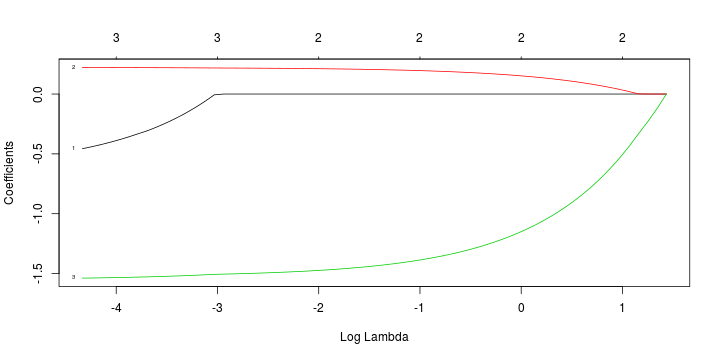## Variable Selection

step(model)

## Start:  AIC=275.26
## dist ~ speed
##
##         Df Sum of Sq   RSS AIC
## <none>               11354 275
## - speed  1     21185 32539 326

##
## Call:
## lm(formula = dist ~ speed, data = cars)
##
## Coefficients:
## (Intercept)        speed
##      -17.58         3.93


model_acp=lm(Fire ~ princomp(cbind(X_1,X_2,X_3))$scores[,1:3],data=chicago) predict(model_c)[1:5]  ## 1 2 3 4 5 ## 9.9755 16.9851 14.2446 13.4120 18.3372  predict(model_acp)[1:5]  ## 1 2 3 4 5 ## 9.9755 16.9851 14.2446 13.4120 18.3372  ## Dimension Reduction model_acp=lm(Fire ~ princomp(cbind(X_1,X_2,X_3))$scores[,1:2],data=chicago)
predict(model_c)[1:5]

##       1       2       3       4       5
##  9.9755 16.9851 14.2446 13.4120 18.3372

predict(model_acp)[1:5]

##      1      2      3      4      5
## 10.010 17.021 14.300 13.439 18.394


## Logistic Regression

$\mathbb{E}[Y\vert \boldsymbol{X}=\boldsymbol{x}]= p(\boldsymbol{x})=\frac{\exp[\boldsymbol{x}^{\text{ T}}\boldsymbol{\beta}]}{1+\exp[\boldsymbol{x}^{\text{ T}}\boldsymbol{\beta}]}=H(\boldsymbol{x}^{\text{ T}}\boldsymbol{\beta})$

logistic regression

## Logistic Regression

myocarde <-read.table("http://freakonometrics.free.fr/myocarde.csv", head=TRUE,sep=";")
model_bernoulli = glm(PRONO~., data=myocarde, family=binomial)
coefficients(summary(model_bernoulli))

##                Estimate Std. Error   z value Pr(>|z|)
## (Intercept) -10.1876417 11.8952271 -0.856448  0.39175
## FRCAR         0.1381781  0.1141122  1.210897  0.22593
## INCAR        -5.8624290  6.7487855 -0.868664  0.38503
## INSYS         0.7170840  0.5614447  1.277212  0.20153
## PRDIA        -0.0736682  0.2916360 -0.252603  0.80057
## PAPUL         0.0167565  0.3419423  0.049004  0.96092
## PVENT        -0.1067760  0.1105501 -0.965861  0.33411
## REPUL        -0.0031542  0.0048909 -0.644907  0.51899


## Logistic Regression

X=cbind(1,as.matrix(myocarde[,1:7]))
Y=myocarde$PRONO=="Survival" beta=as.matrix(lm(Y~0+X)$coefficients,ncol=1)
for(s in 1:9){
pi=exp(X%*%beta[,s])/(1+exp(X%*%beta[,s]))
omega=matrix(0,nrow(X),nrow(X));diag(omega)=(pi*(1-pi))
Hessian=-t(X)%*%omega%*%X


## Logistic Regression

 beta[,1]

##      X XFRCAR XINCAR XINSYS XPRDIA XPAPUL XPVENT XREPUL
##      0      0      0      0      0      0      0      0

 beta[,9]

##           X      XFRCAR      XINCAR      XINSYS      XPRDIA      XPAPUL
## -9.2027e+00  4.7726e-14 -5.7554e-13  4.5408e-14  4.9960e-16  2.6645e-15
##      XPVENT      XREPUL
##  1.3878e-15 -2.0817e-17

# -solve(Hessian)
sqrt(-diag(solve(Hessian)))

##                 FRCAR      INCAR      INSYS      PRDIA      PAPUL      PVENT
## 180.183682   1.877488  93.030245   6.520984   6.346283   5.471882   3.059004
##      REPUL
##   0.054721


## Logistic Regression

GLM <- glm(PRONO ~ PVENT + REPUL, data = myocarde, family = binomial)
pred_GLM = function(p,r){
return(predict(GLM, newdata =
data.frame(PVENT=p,REPUL=r), type="response"))}
vp=seq(min(myocarde$PVENT),max(myocarde$PVENT),length=251)
vr=seq(min(myocarde$REPUL),max(myocarde$REPUL),length=251)
vd=outer(vp,vr,pred_GLM)
library(RColorBrewer,verbose=FALSE,quietly=TRUE,warn.conflicts=FALSE)
CL=colorRampPalette(brewer.pal(name="RdYlBu", 11))(100)


## Logistic Regression

image(vp,vr,vd,col=CL,xlab="PVENT",ylab="REPUL")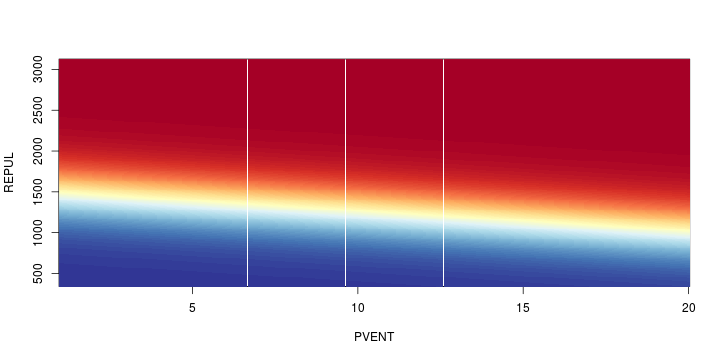## Logistic Regression

probabilities <- predict(GLM, myocarde, type="response")
predictions <- levels(myocarde$PRONO)[(probabilities>.5)+1] table(predictions, myocarde$PRONO)

##
## predictions DECES SURVIE
##      DECES     24      4
##      SURVIE     5     38


## Residuals and GLM

Pearson's residuals $\widehat{\varepsilon}_i=\frac{y_i - \widehat{\pi}_i}{ \sqrt{\widehat{\pi}_i\cdot[1-\widehat{\pi}_i]}}$ Deviance residuals $\widehat{\varepsilon}_i=\pm \left( y_i \log[\widehat{\pi}_i]+ (1-y_i) \log[1-\widehat{\pi}_i]\right)$

E1=residuals(model_bernoulli,type="pearson")
E2=residuals(model_bernoulli,type="deviance")


## Residuals and GLM

plot(1:nrow(myocarde),E1)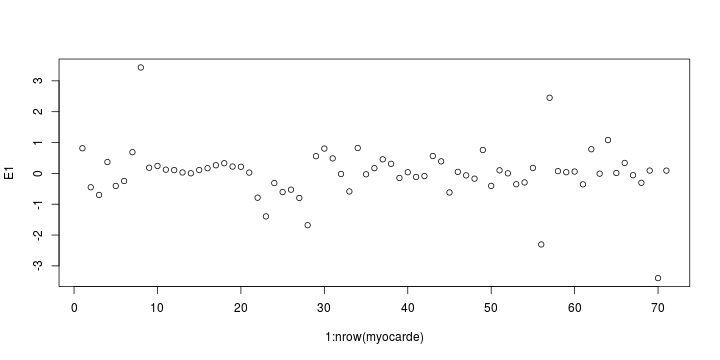## Multinomial Regression

Binomial case, $Y\in\lbrace 0,1\rbrace$,

$\mathbb{P}[Y=1\vert \boldsymbol{X}=\boldsymbol{x}]\propto\exp[\boldsymbol{x}^{\text{ T}}\boldsymbol{\beta}]$

$\mathbb{P}[Y=0\vert \boldsymbol{X}=\boldsymbol{x}]\propto1$

Multinomial case, $Y\in\lbrace A,B,C\rbrace$,

$\mathbb{P}[Y=\color{red}{A}\vert \boldsymbol{X}=\boldsymbol{x}]\propto\exp[\boldsymbol{x}^{\text{ T}}\color{red}{\boldsymbol{\beta}_A}]$

$\mathbb{P}[Y=\color{blue}{B}\vert \boldsymbol{X}=\boldsymbol{x}]\propto\exp[\boldsymbol{x}^{\text{ T}}\color{blue}{\boldsymbol{\beta}_B}]$

$\mathbb{P}[Y=\color{green}{C}\vert \boldsymbol{X}=\boldsymbol{x}]\propto1$

## Multinomial Regression

library(VGAM,verbose=FALSE,quietly=TRUE,warn.conflicts=FALSE)
model_multi = vglm(PRONO~., data=myocarde, family="multinomial")
model_multi

## Call:
## vglm(formula = PRONO ~ ., family = "multinomial", data = myocarde)
##
## Coefficients:
## (Intercept)       FRCAR       INCAR       INSYS       PRDIA       PAPUL
##  10.1876411  -0.1381781   5.8624289  -0.7170840   0.0736682  -0.0167565
##       PVENT       REPUL
##   0.1067760   0.0031542
##
## Degrees of Freedom: 71 Total; 63 Residual
## Residual deviance: 41.043
## Log-likelihood: -20.522
##
## This is a multinomial logit model with 2 levels


## Generalized Linear Models

Poisson and Gamma distributions in the exponential family

base=data.frame(x=c(1,2,3,4,5),y=c(1,2,4,2,6))


## Generalized Linear Models

visuel=function(regression,titre=""){
plot(base$x,base$y,pch=19,cex=1.5,main=titre,xlab="",ylab="")
abs <- seq(0,7,by=.1)
yp <- predict(regression,newdata=data.frame(x=abs),se.fit = TRUE, type="response")
polygon(c(abs,rev(abs)),c(yp$fit+2*yp$se.fit,rev(yp$fit-2*yp$se.fit)), col="light green",border=NA)
points(base$x,base$y,pch=19,cex=1.5)
lines(abs,yp$fit,lwd=2,col="red") lines(abs,yp$fit+2*yp$se.fit,lty=2) lines(abs,yp$fit-2*yp\$se.fit,lty=2)}


## Generalized Linear Models

visuel(regNId,"Gaussienne, lien identité")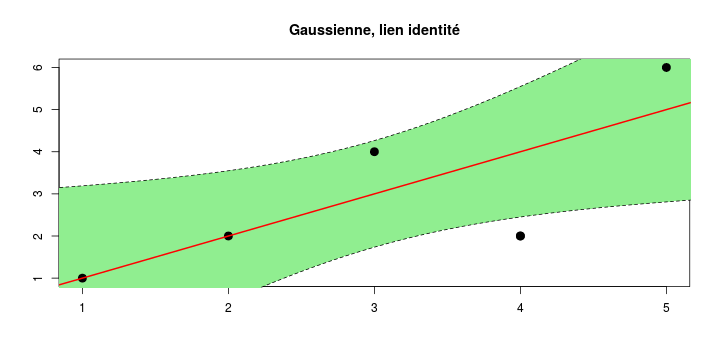## Generalized Linear Models

visuel(regPId,"Poisson, lien identité")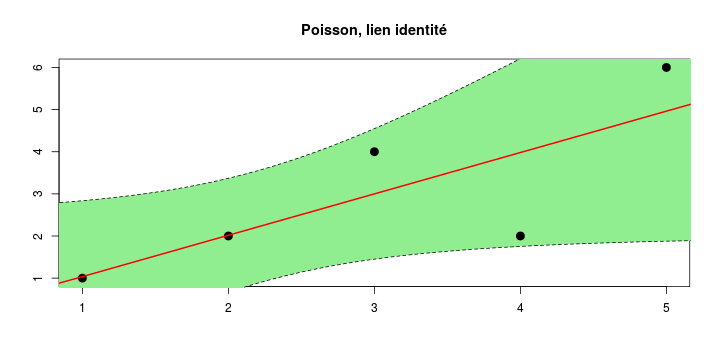## Generalized Linear Models

visuel(regGId,"Gamma, lien identité")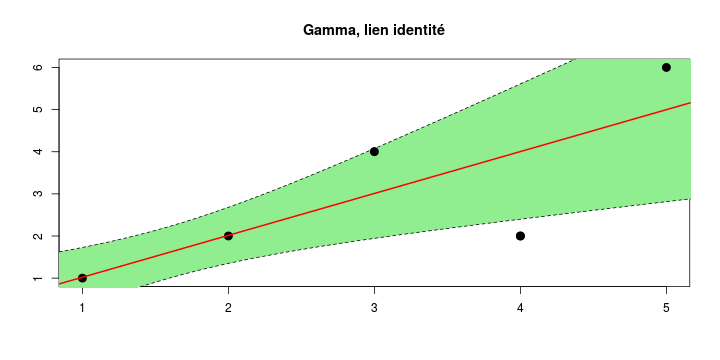## Generalized Linear Models

visuel(regNlog,"Gaussienne, lien logarithmique")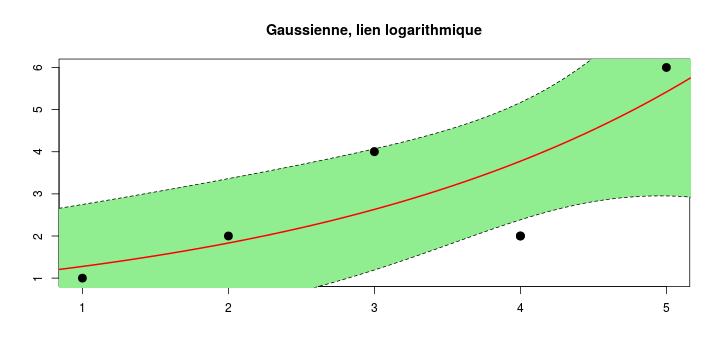## Generalized Linear Models

visuel(regPlog,"Poisson, lien logarithme")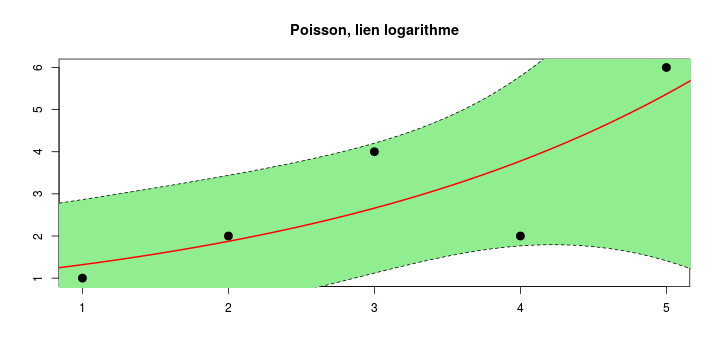## Generalized Linear Models

visuel(regGlog,"Gamma, lien logarithme")